# R S Aggarwal Solutions for Class 11 Maths Chapter 8- Permutations

R S Aggarwal Solutions for Class 11 Maths Chapter 8 Permutations are provided here. It is crucial to understand the concepts taught in Class 11 as these concepts pick up the threads in Class 12. It is advised to solve questions provided in each exercise across all the chapters in the book by R S Aggarwal publication to score exceptionally well in Class 11 Mathematics examination. The R S Aggarwal solutions for Class 11 Maths helps students in mastering all the concepts in an effective way. Download pdf of Class 11 Maths Chapter 8 R S Aggarwal Solutions from the link given below.

### Download PDF of R S Aggarwal Solutions for Class 11 Maths Chapter 8- Permutations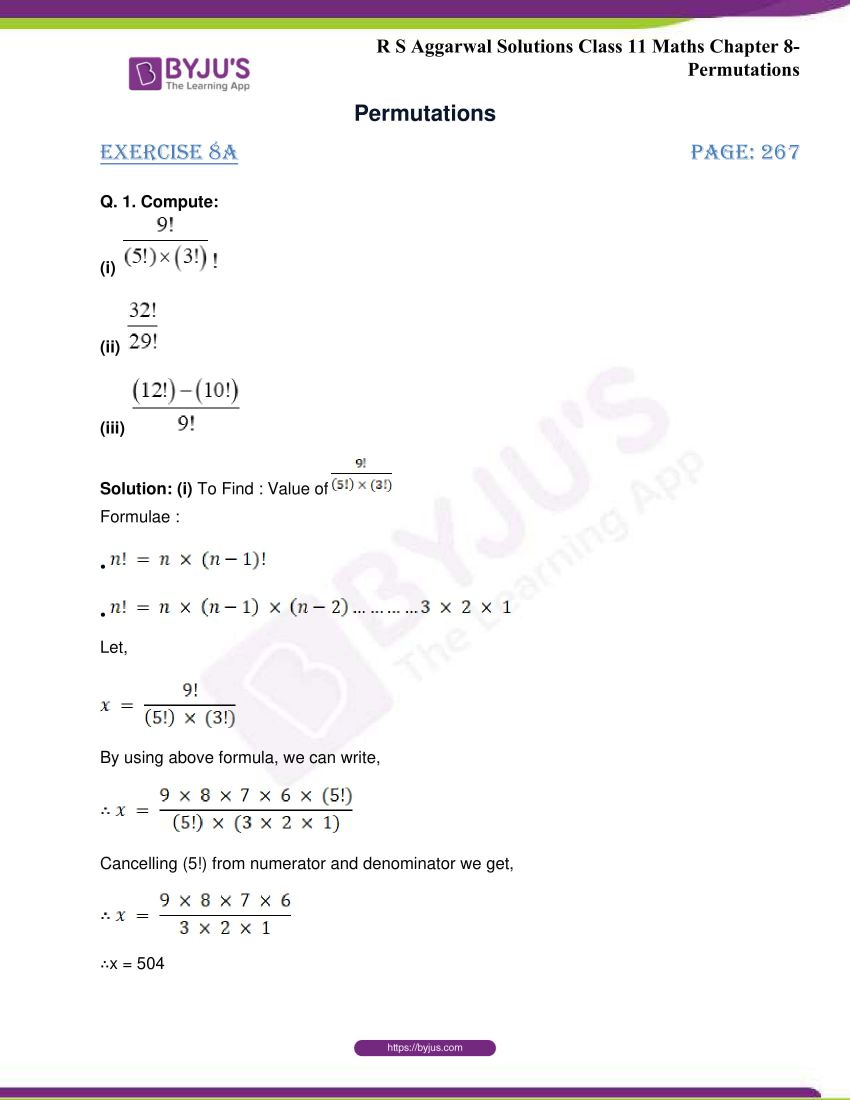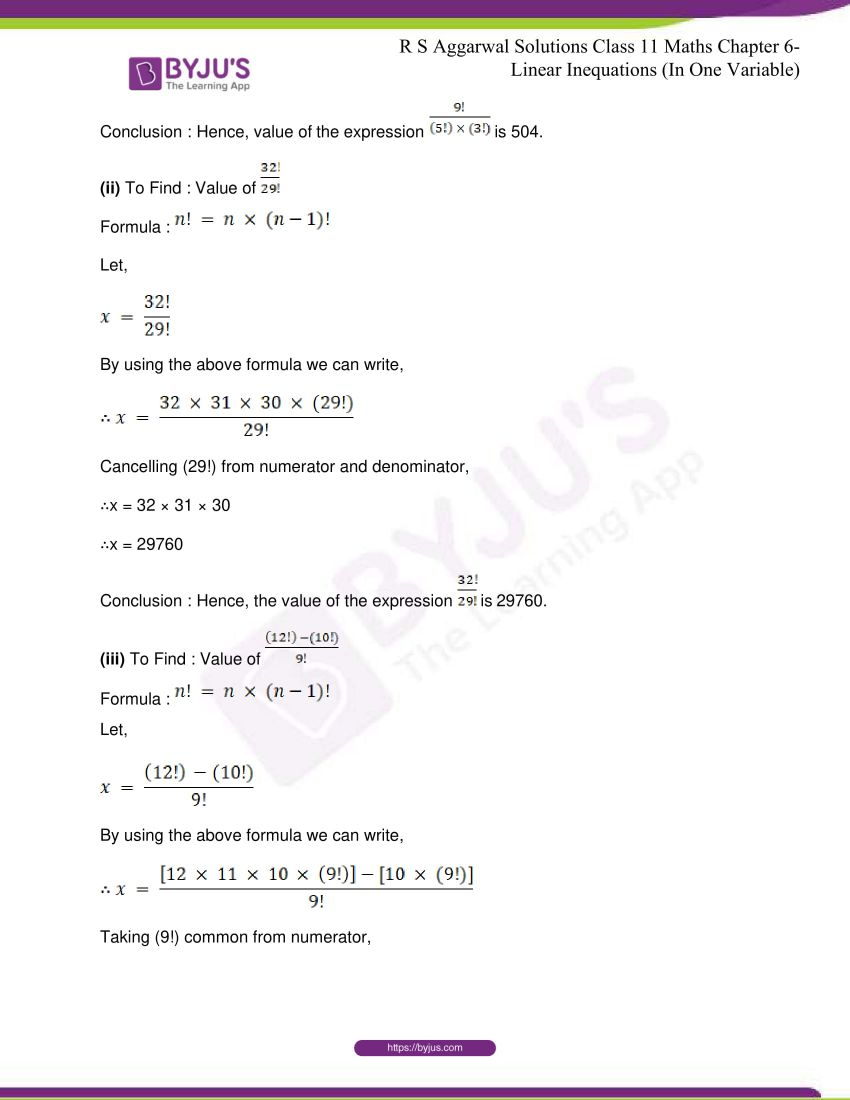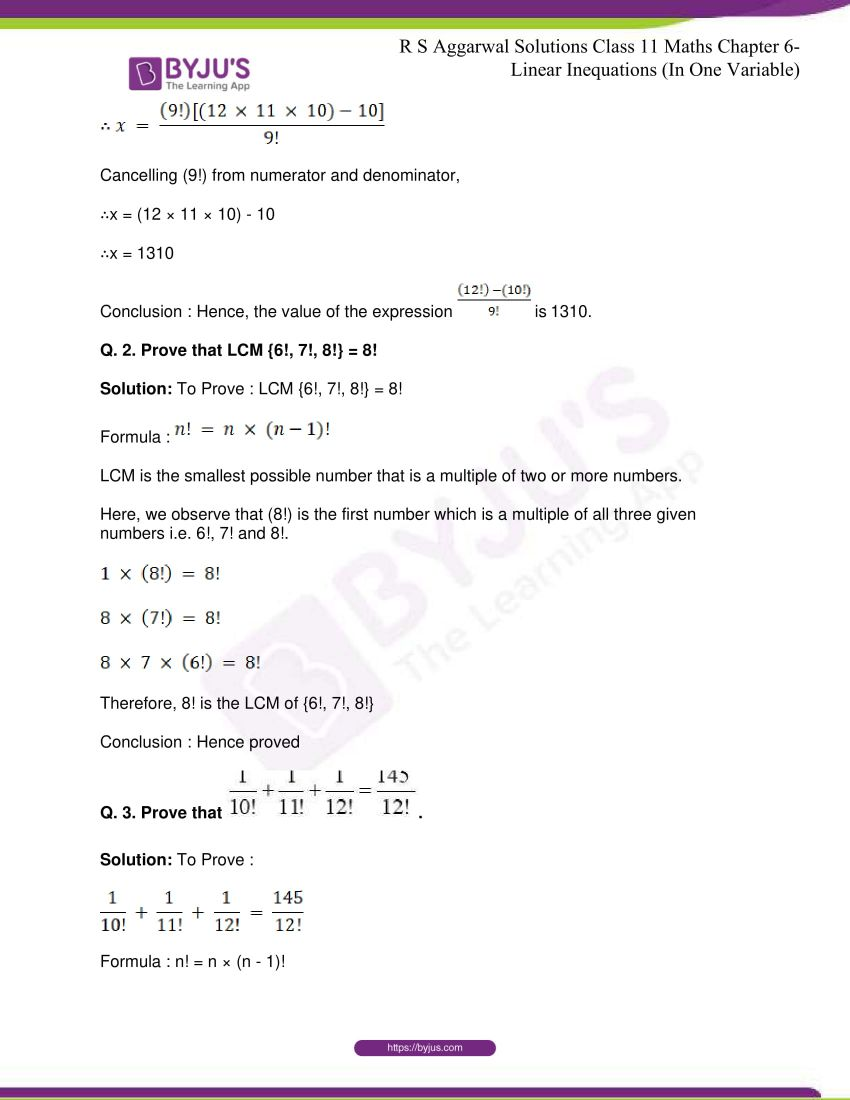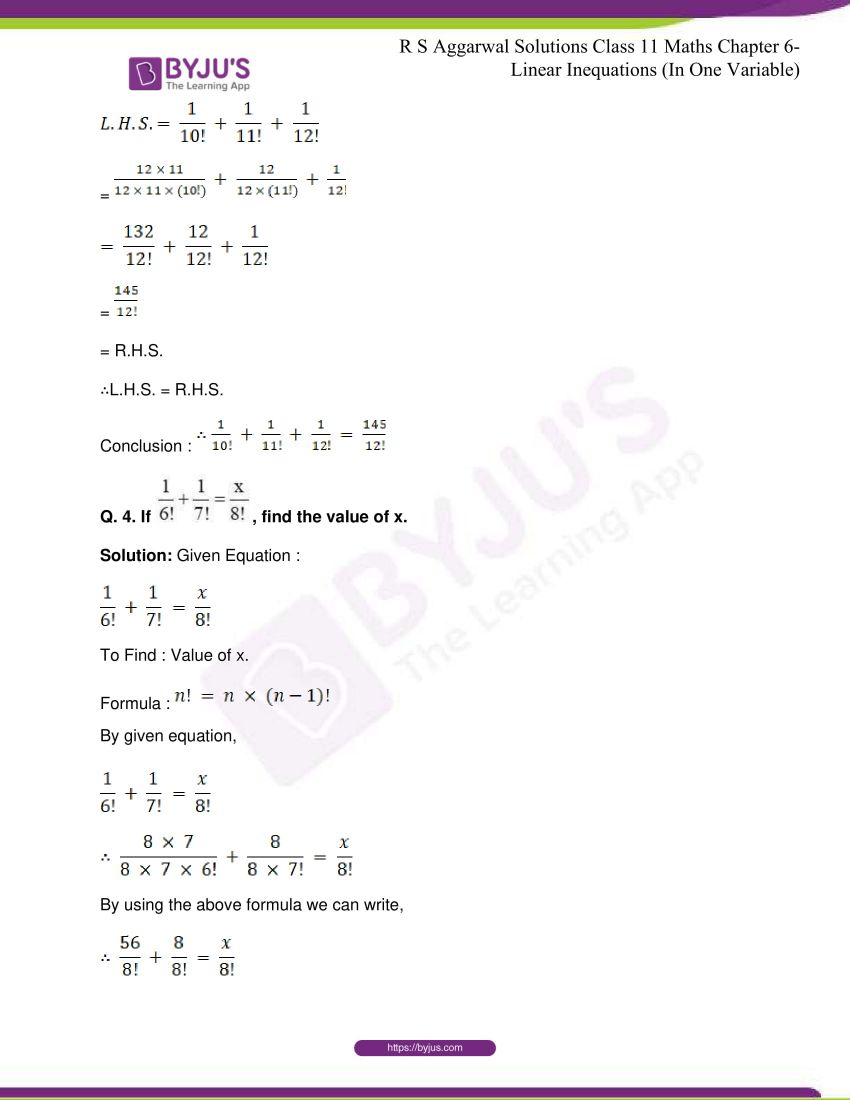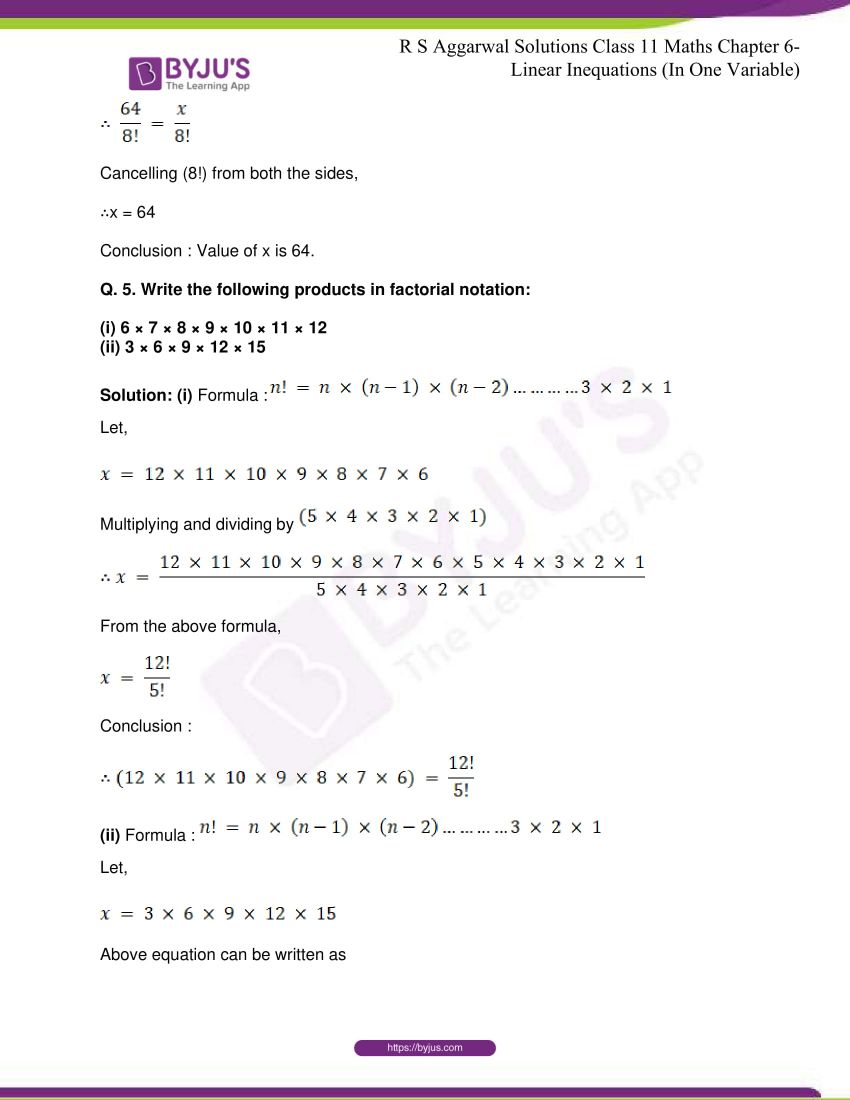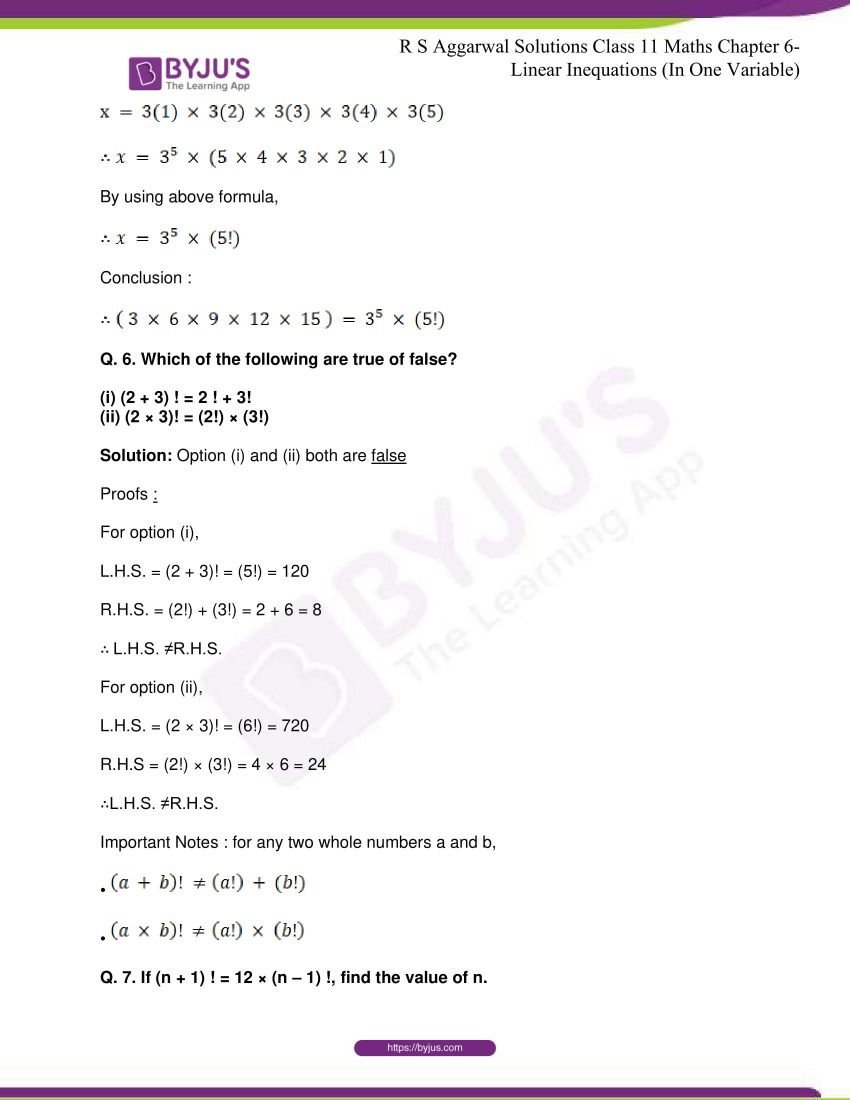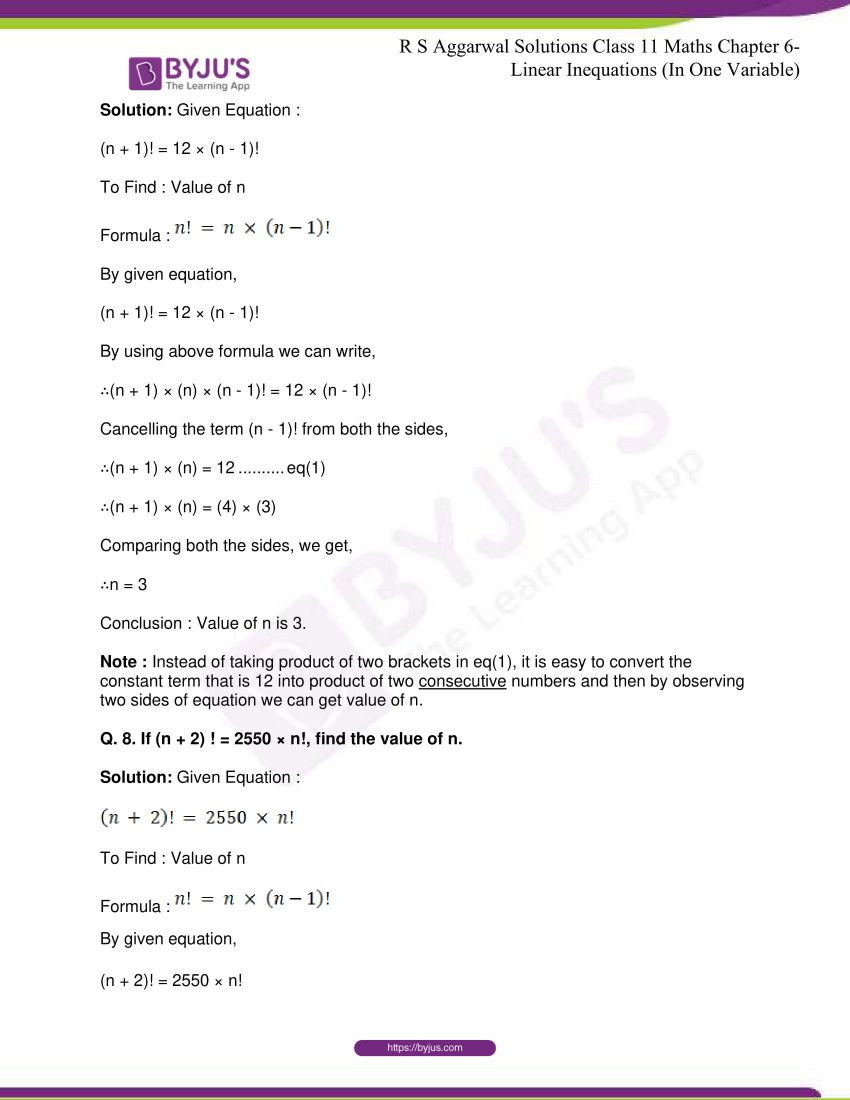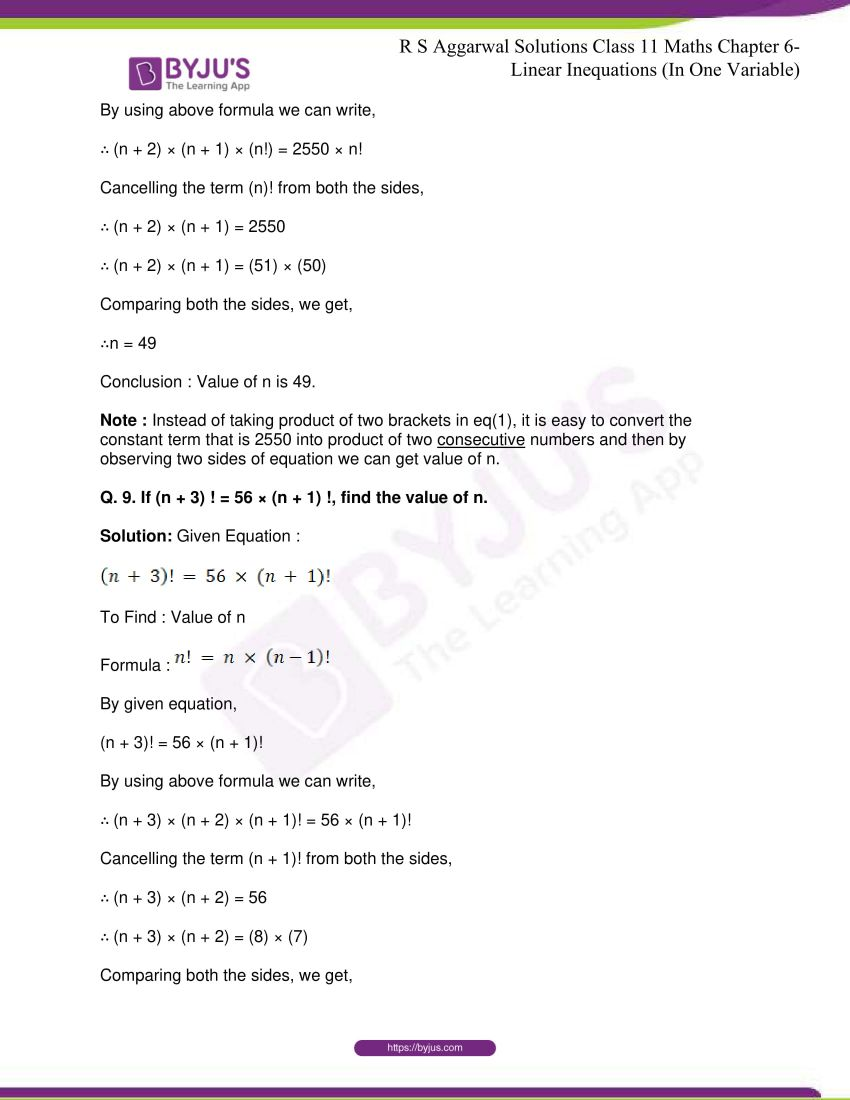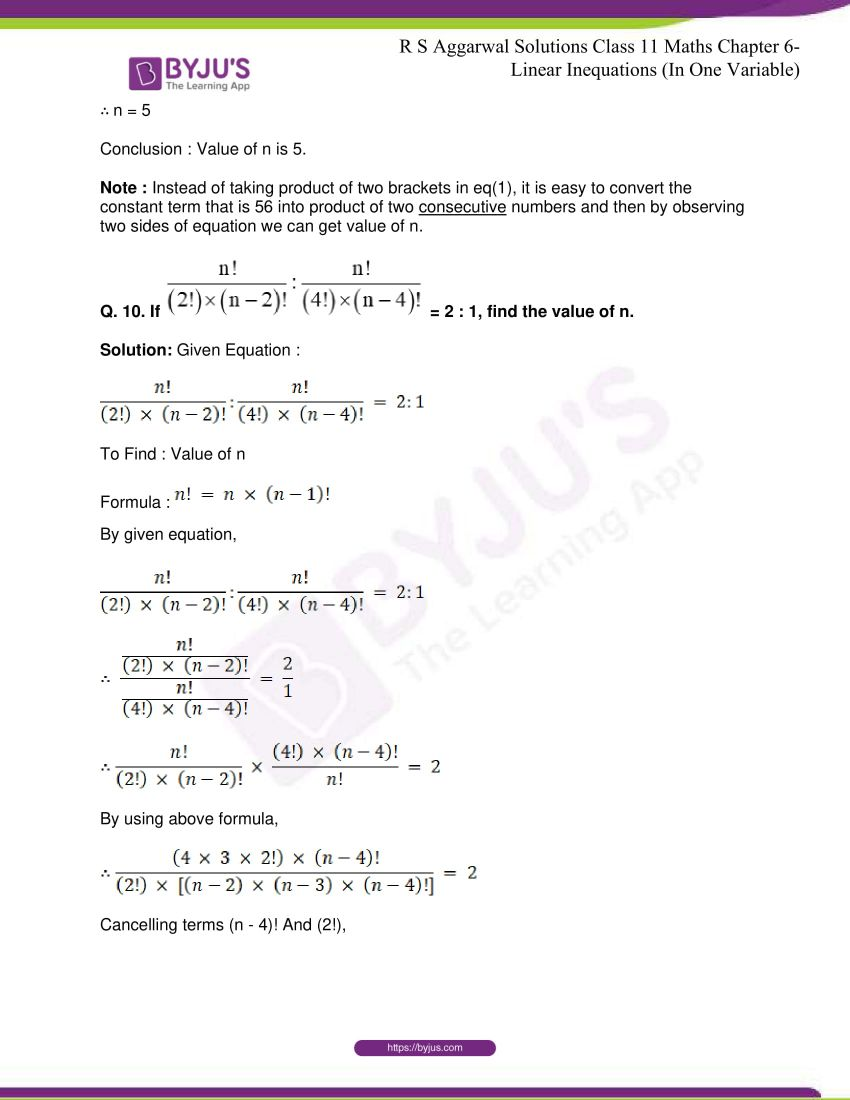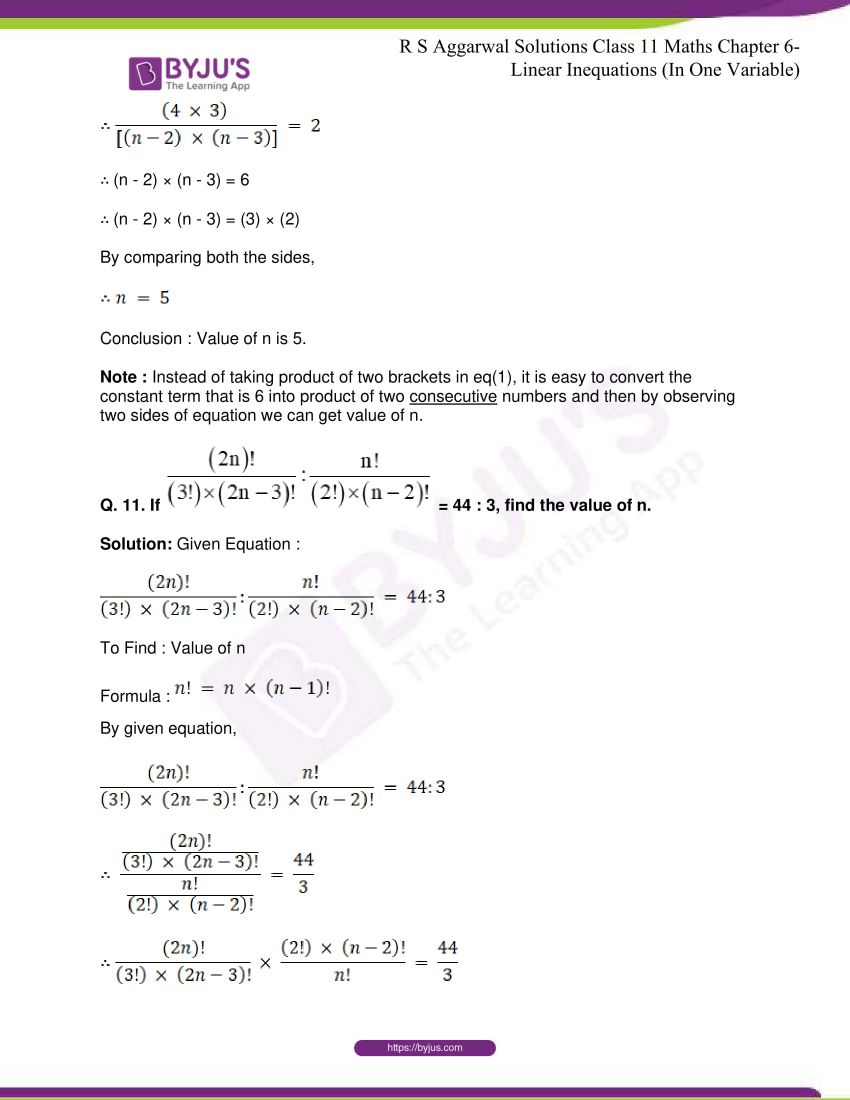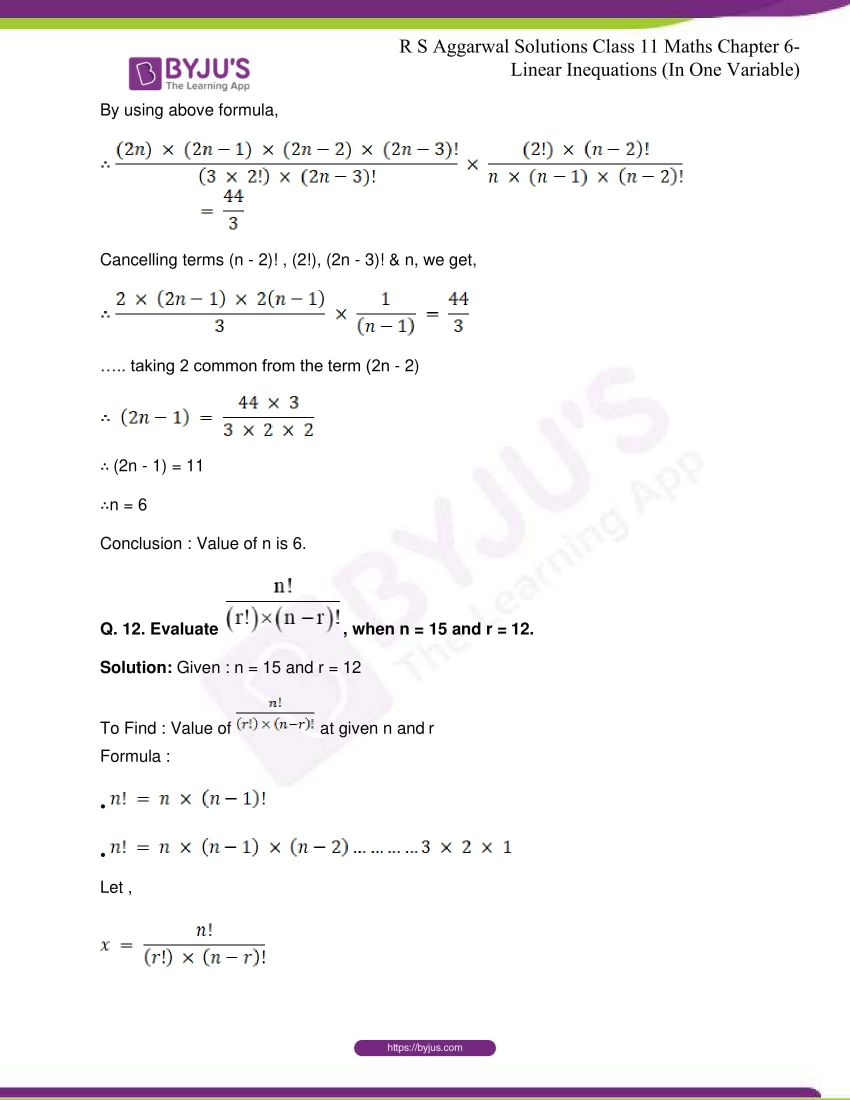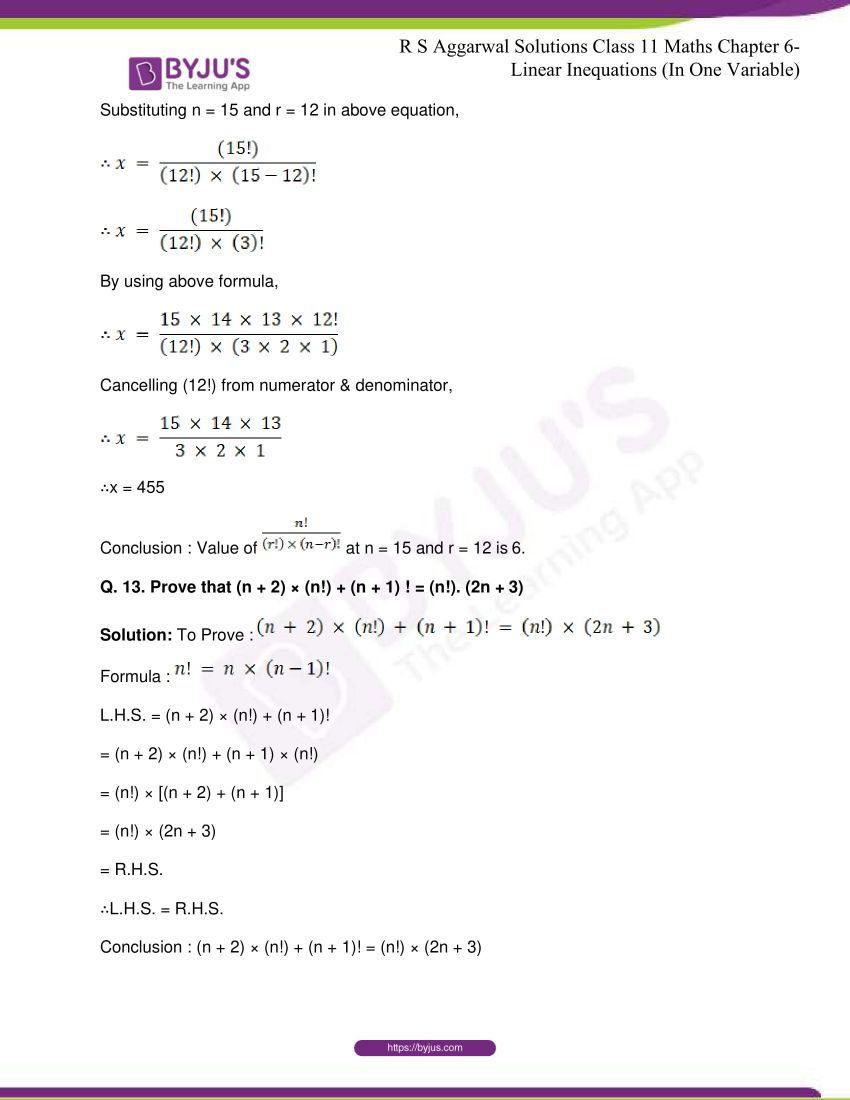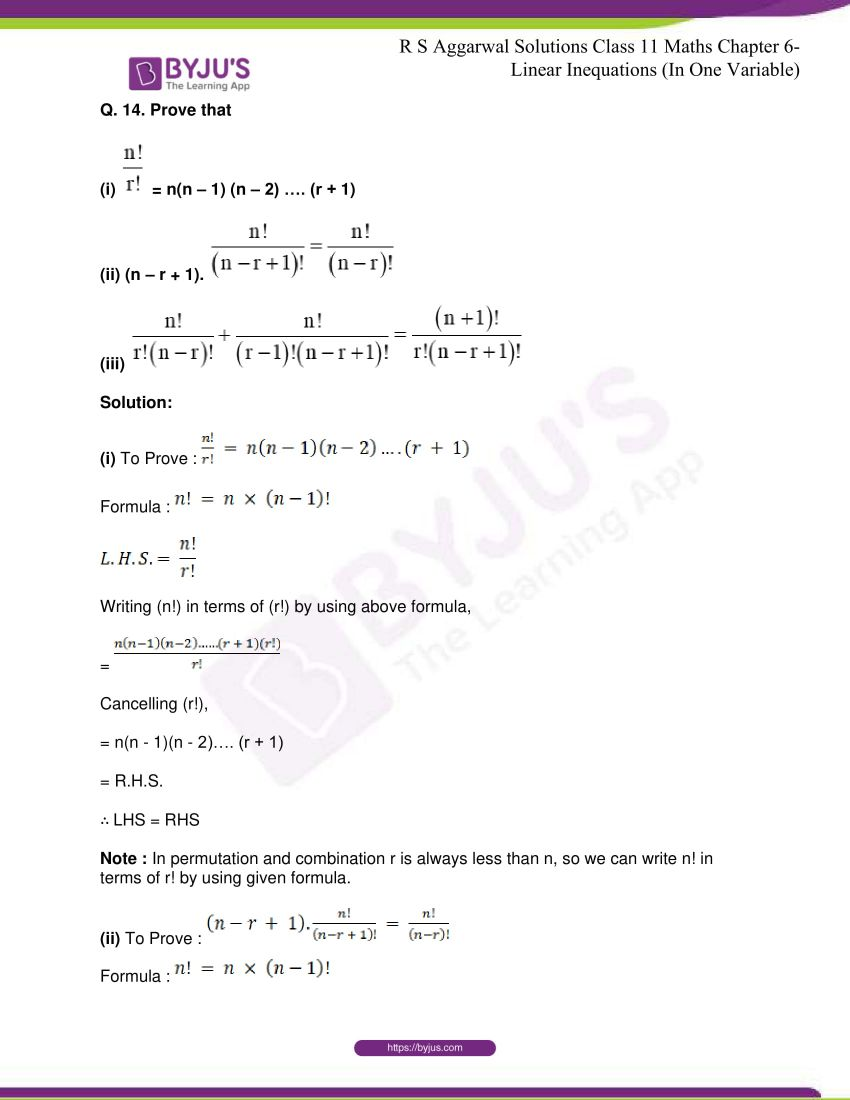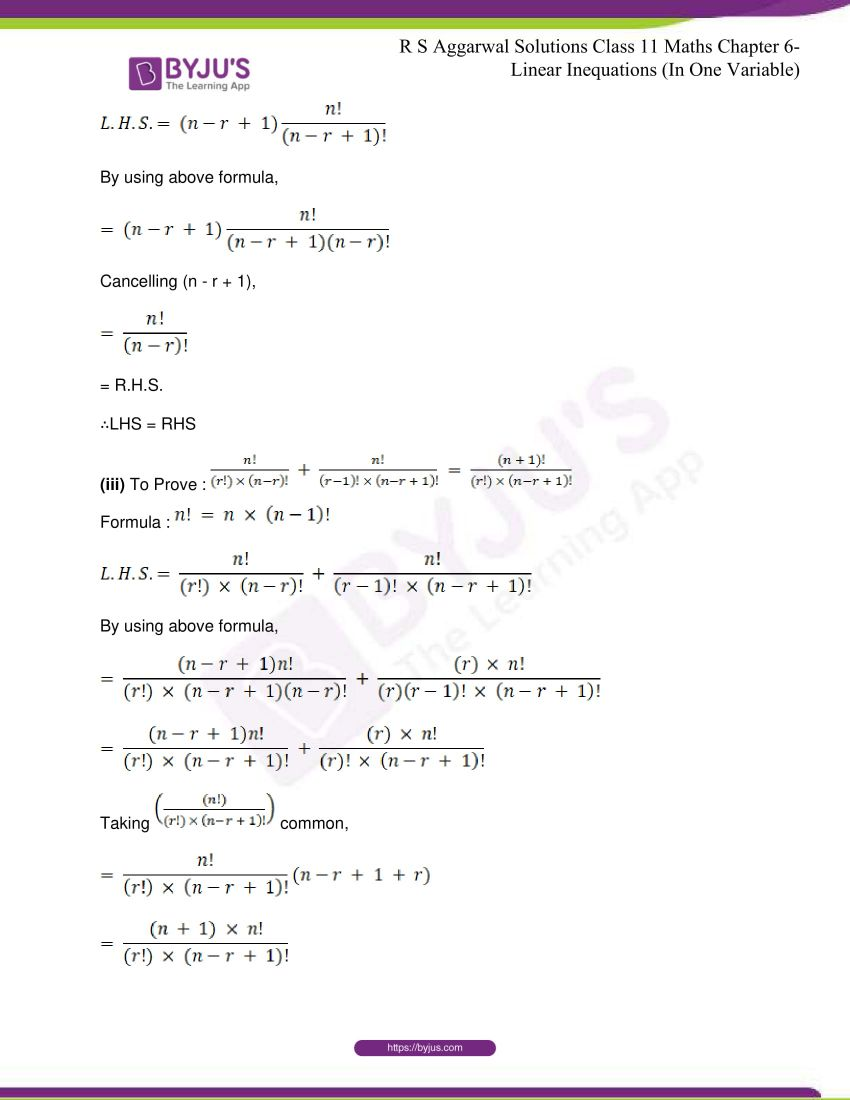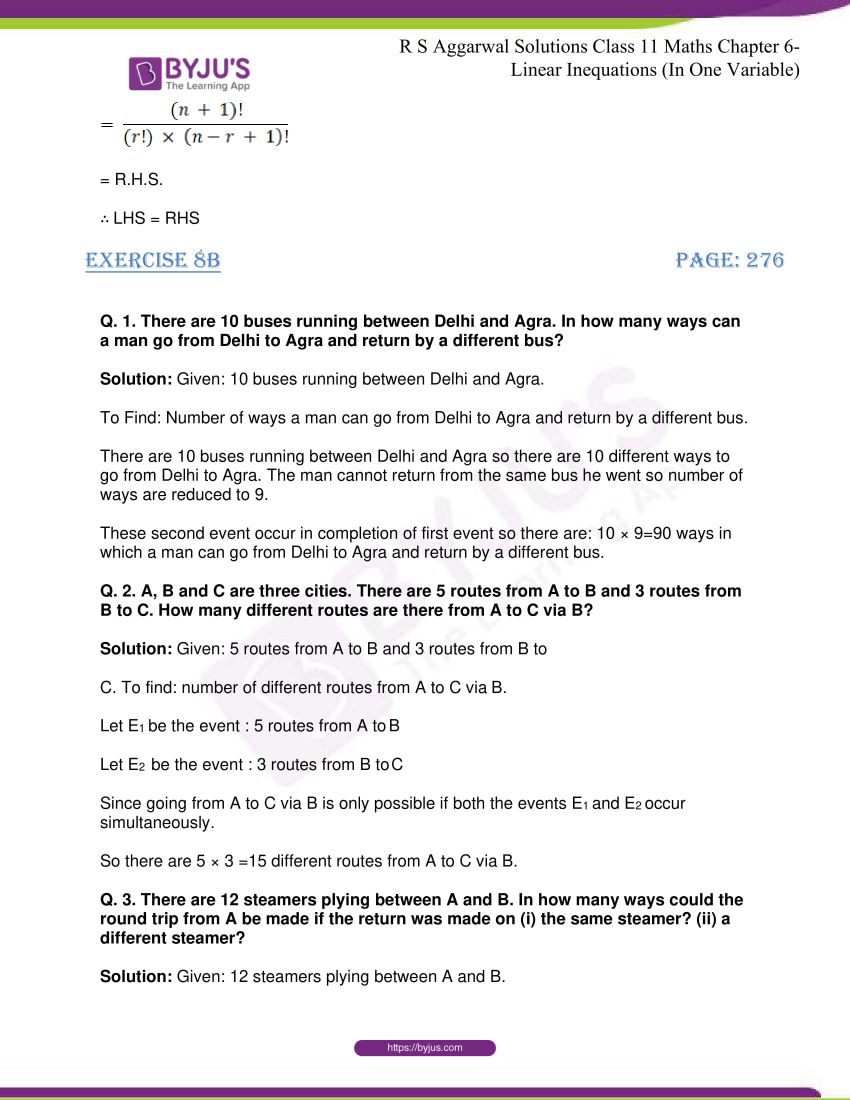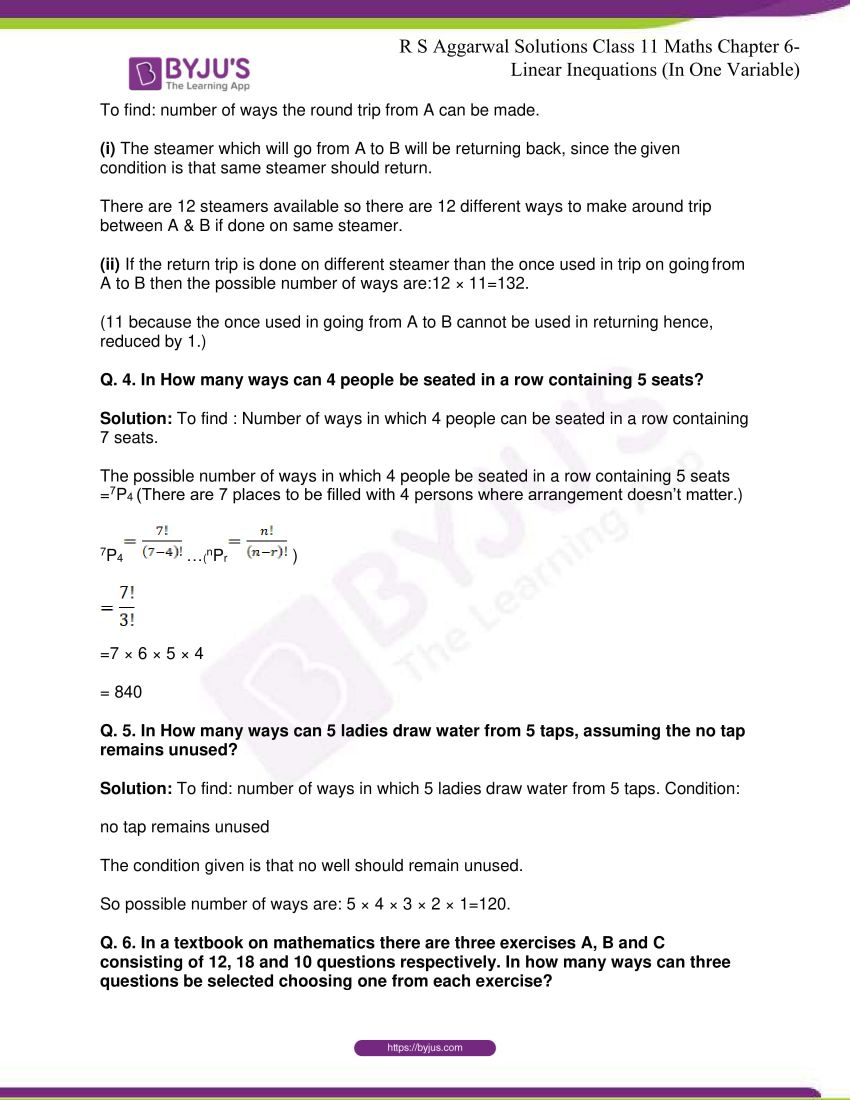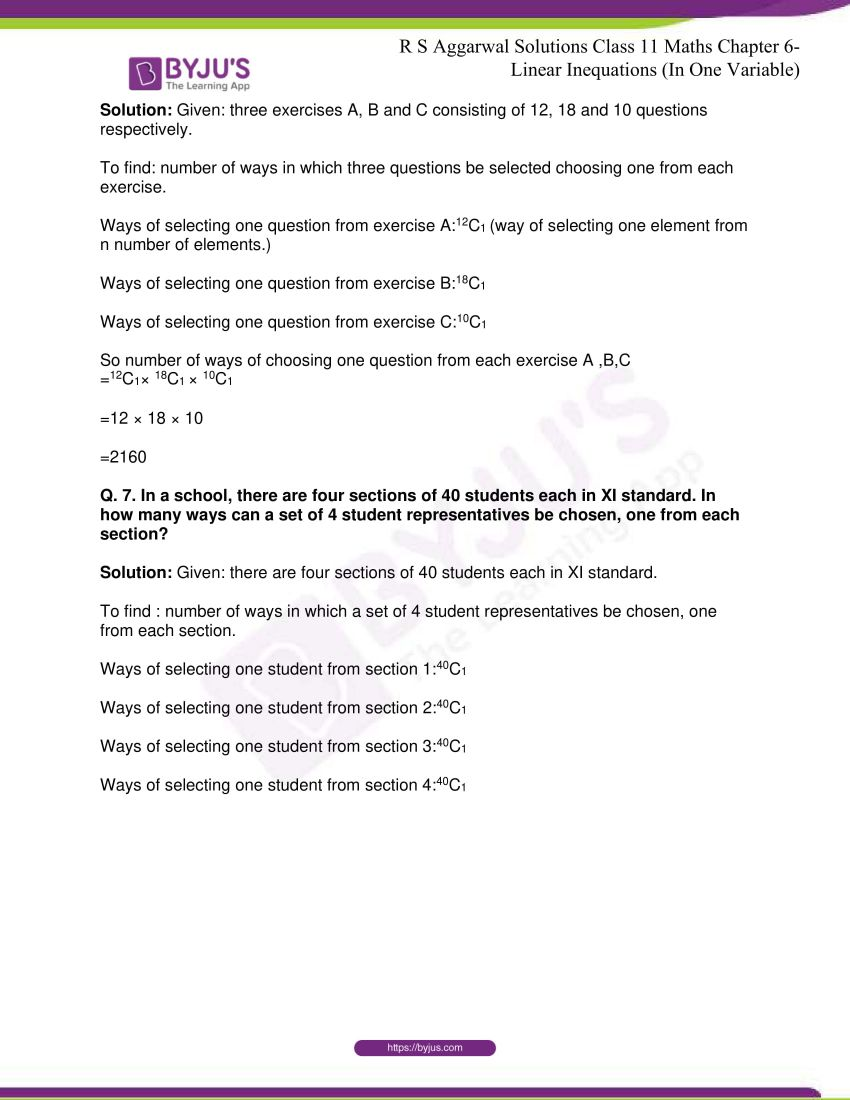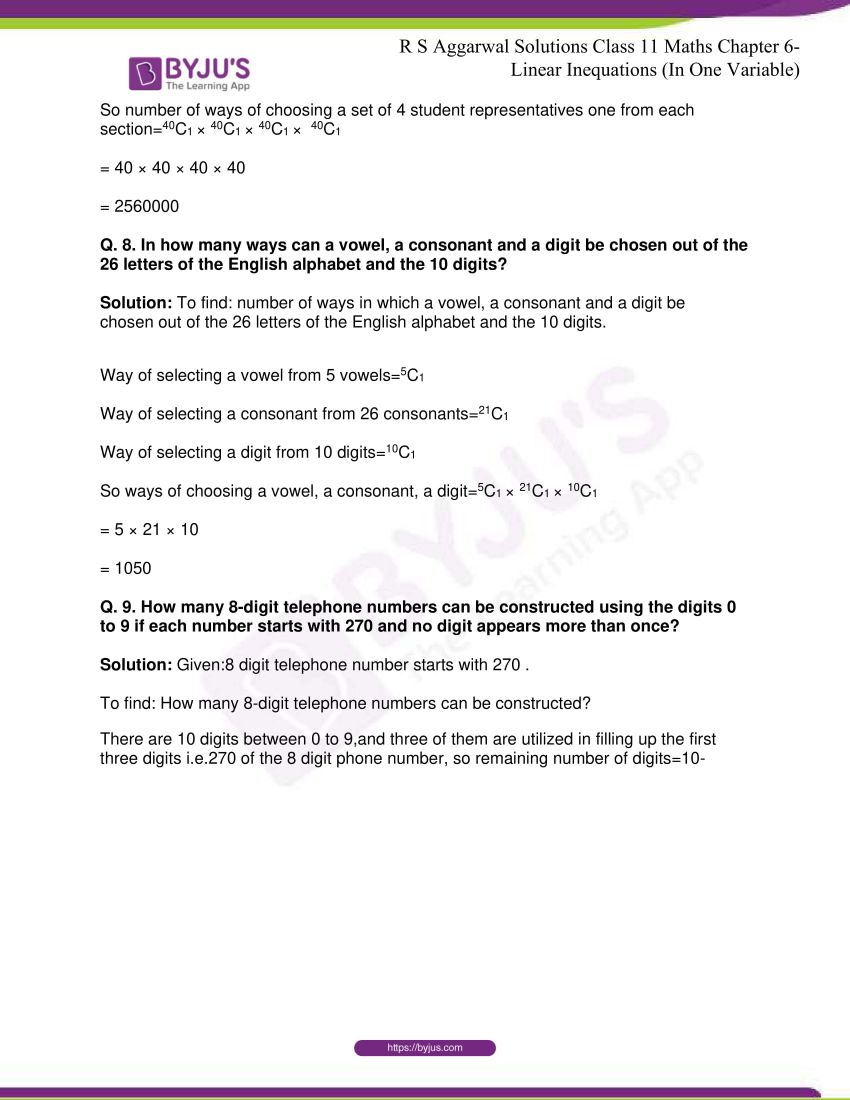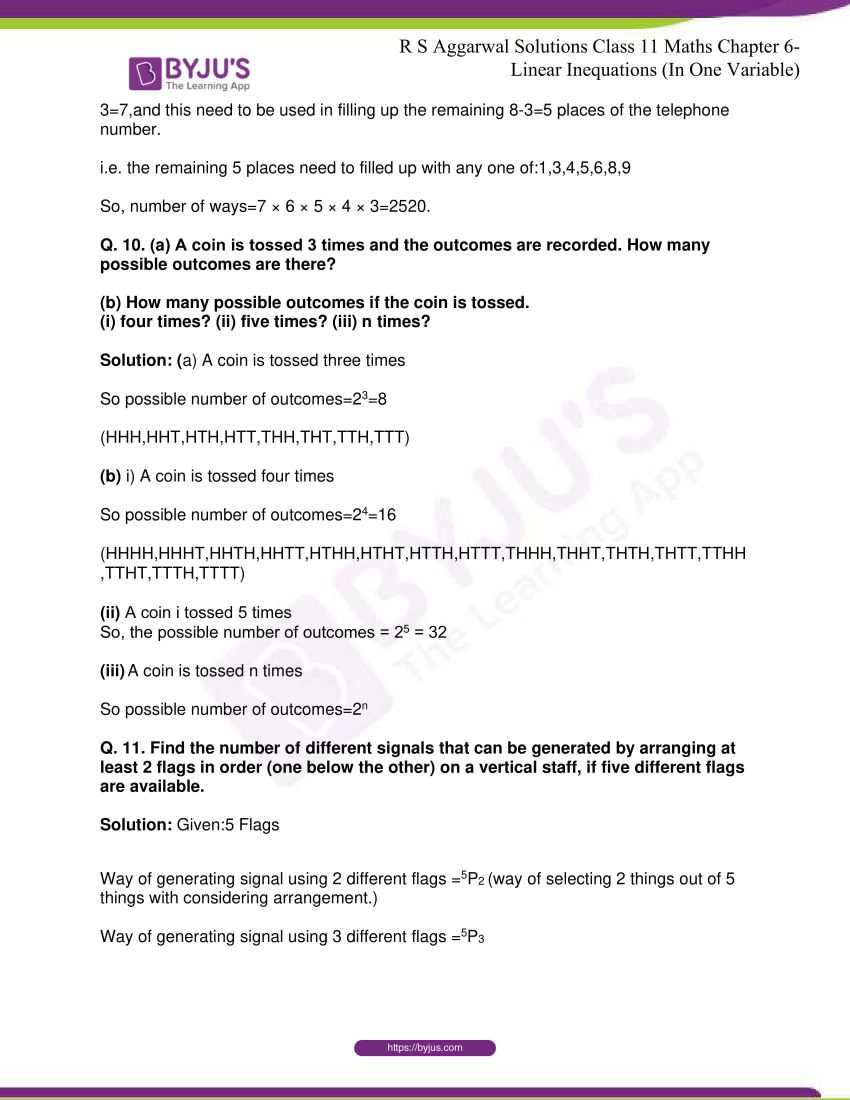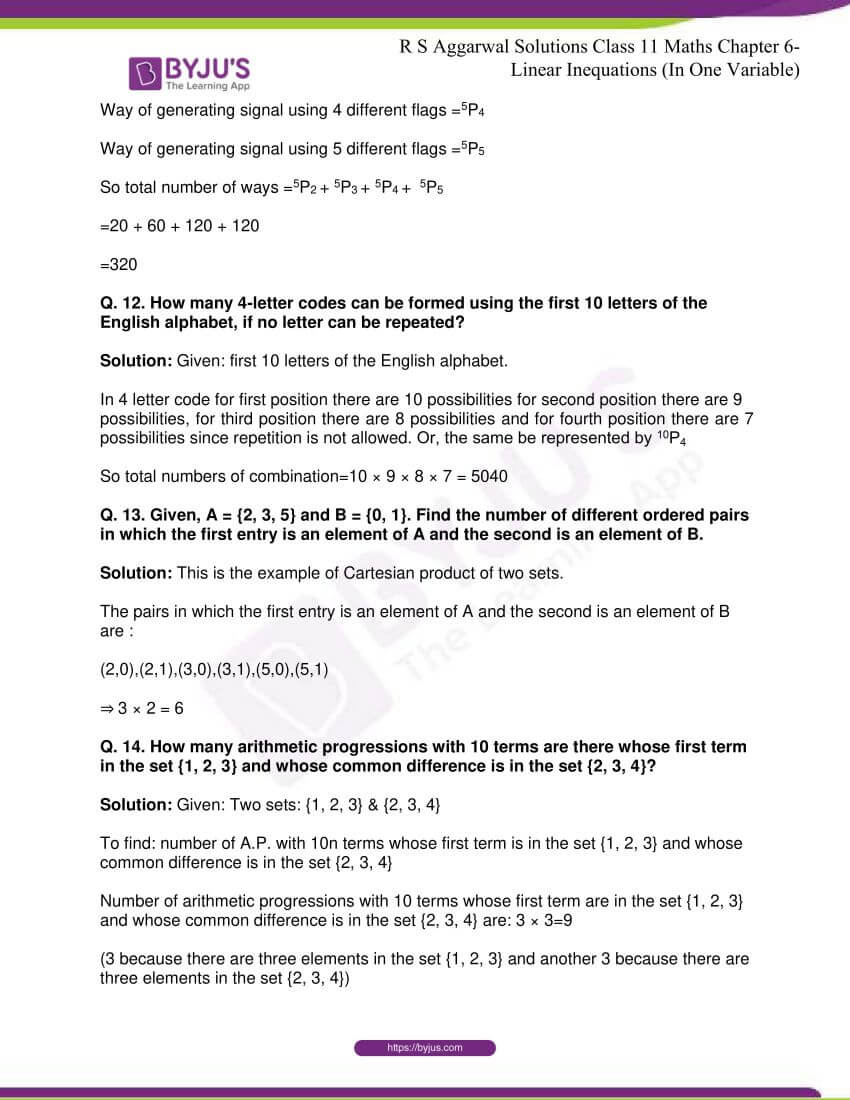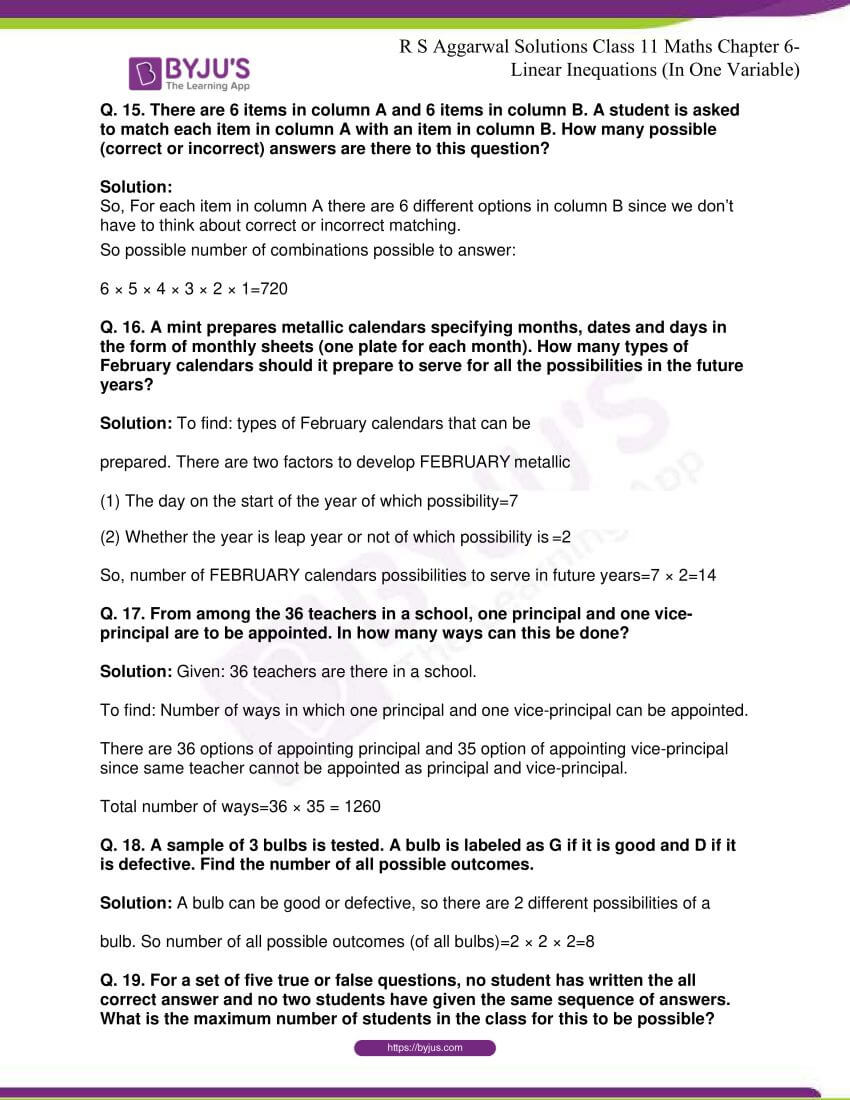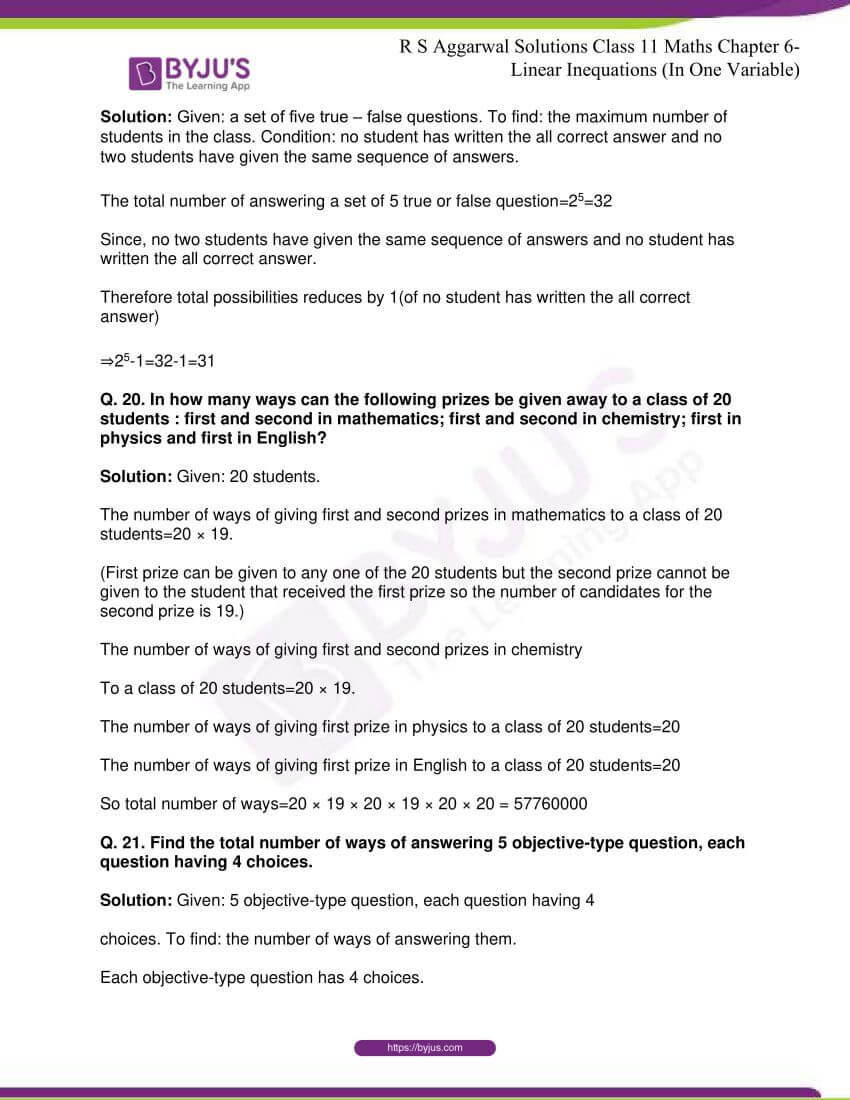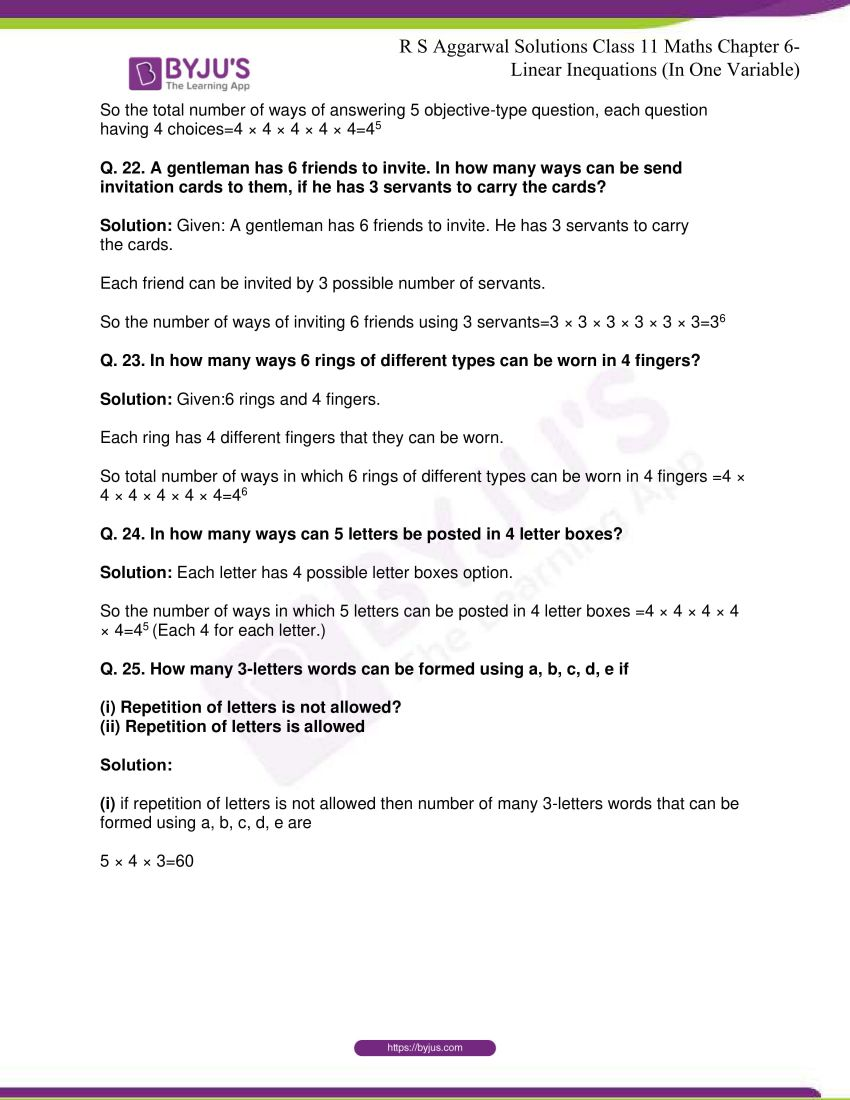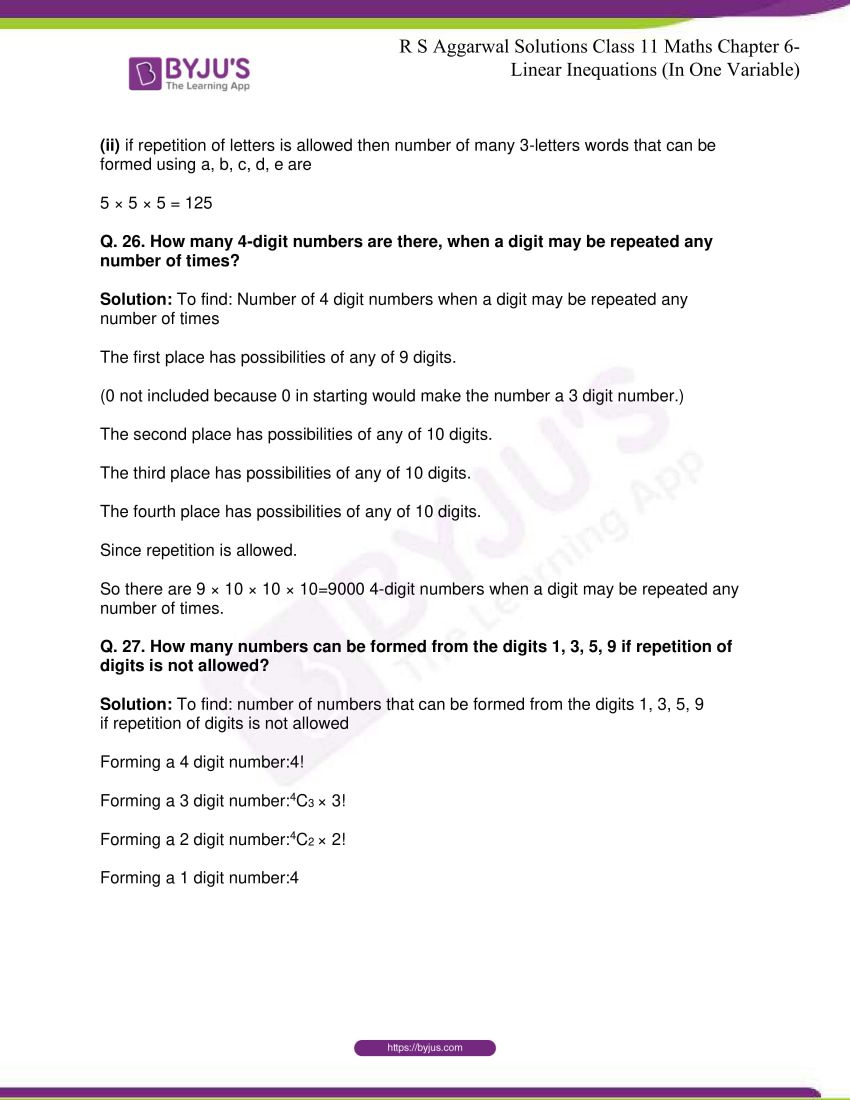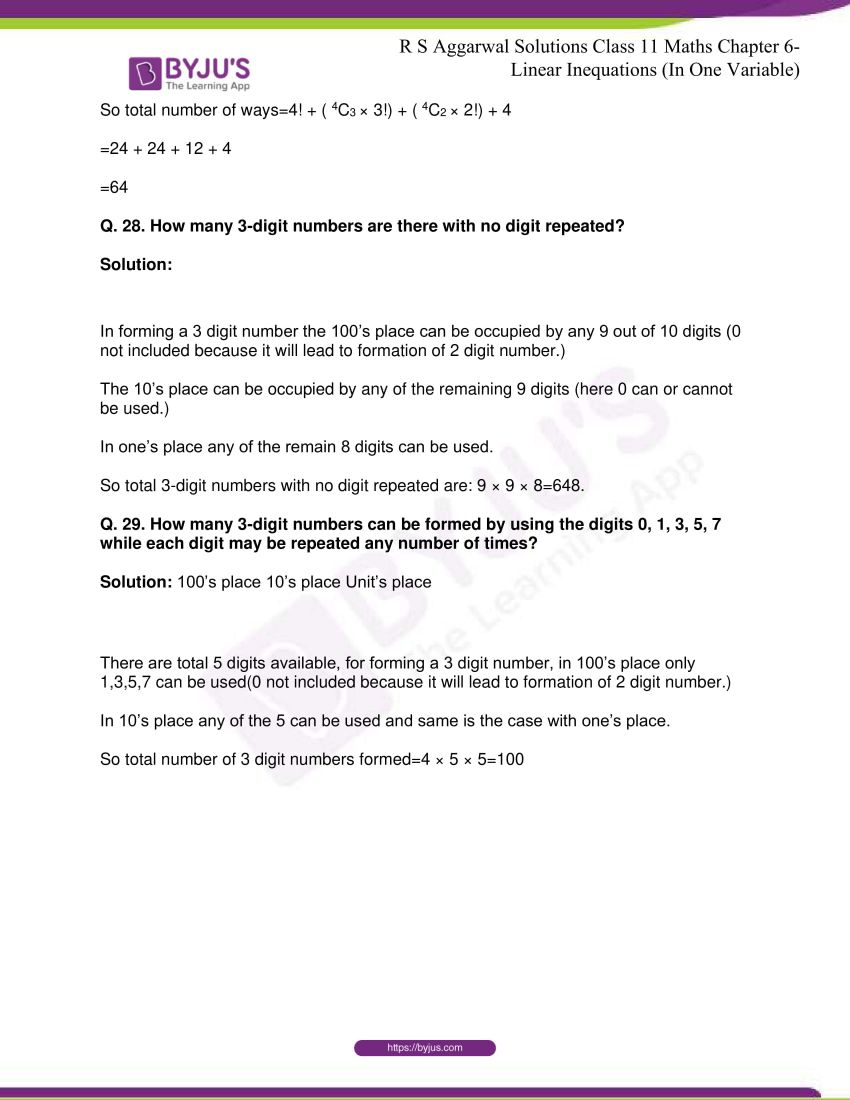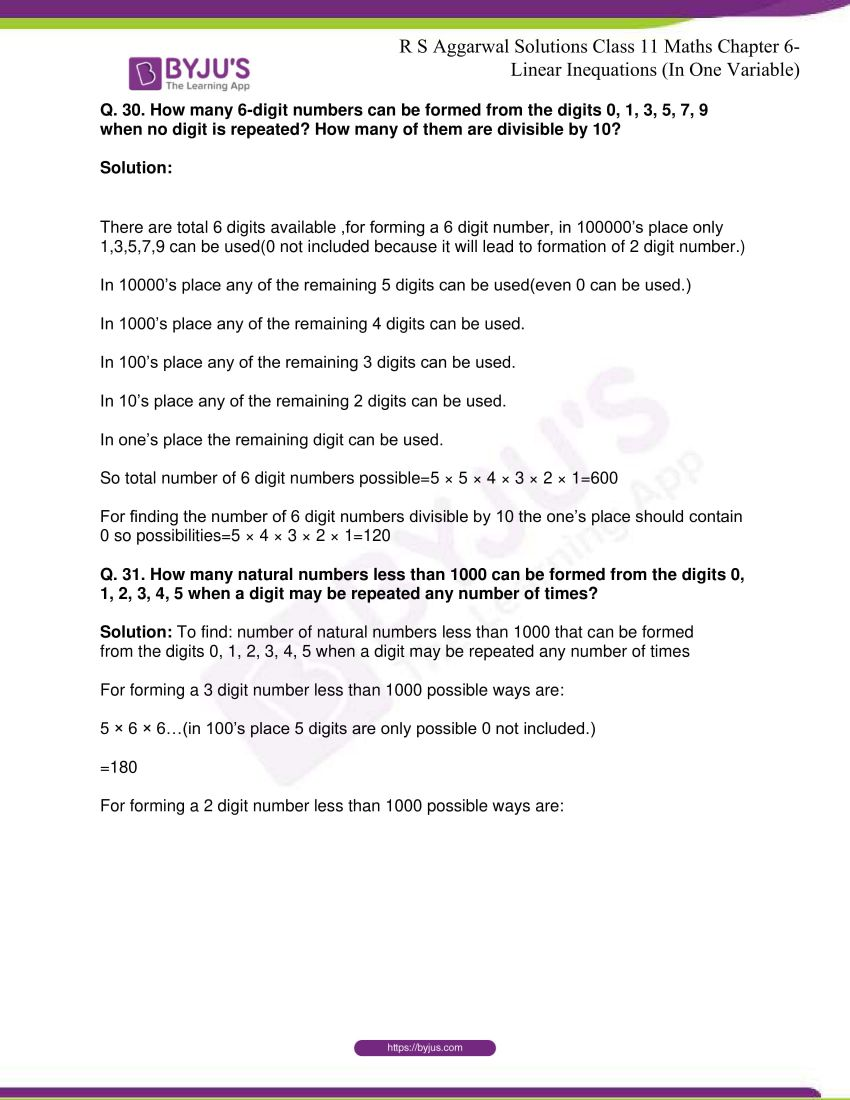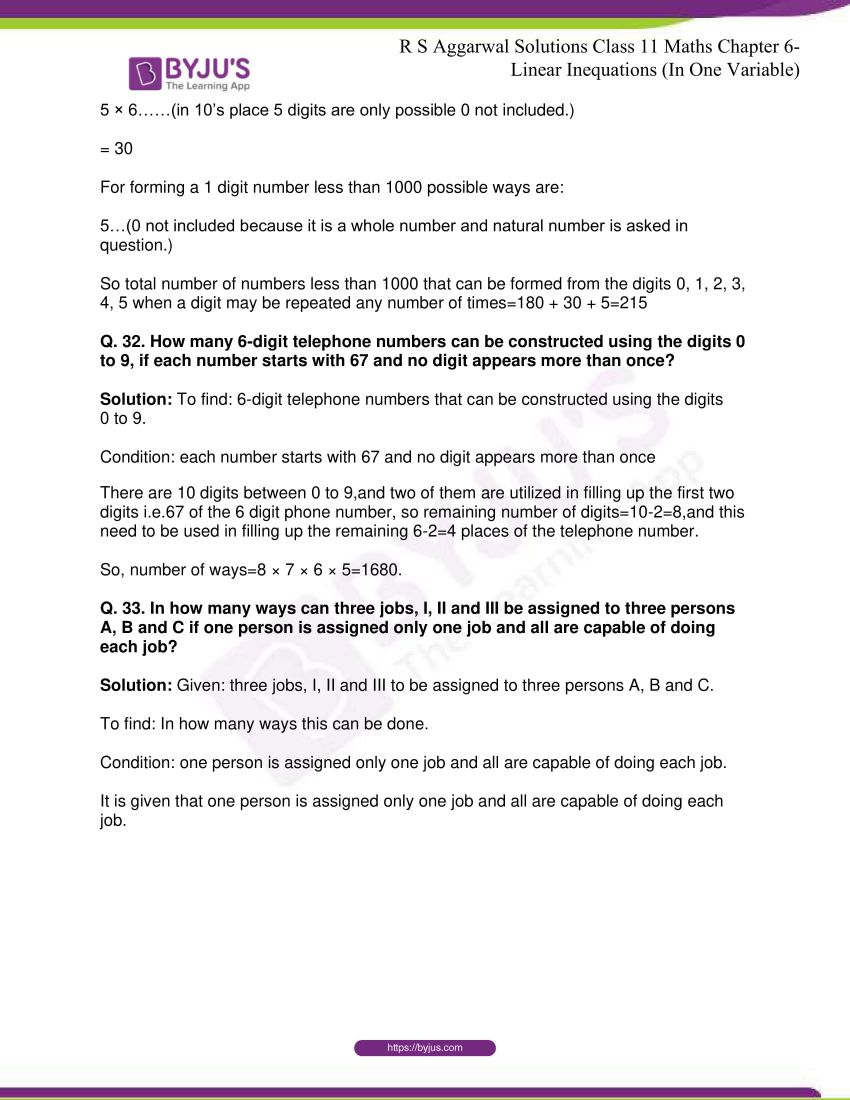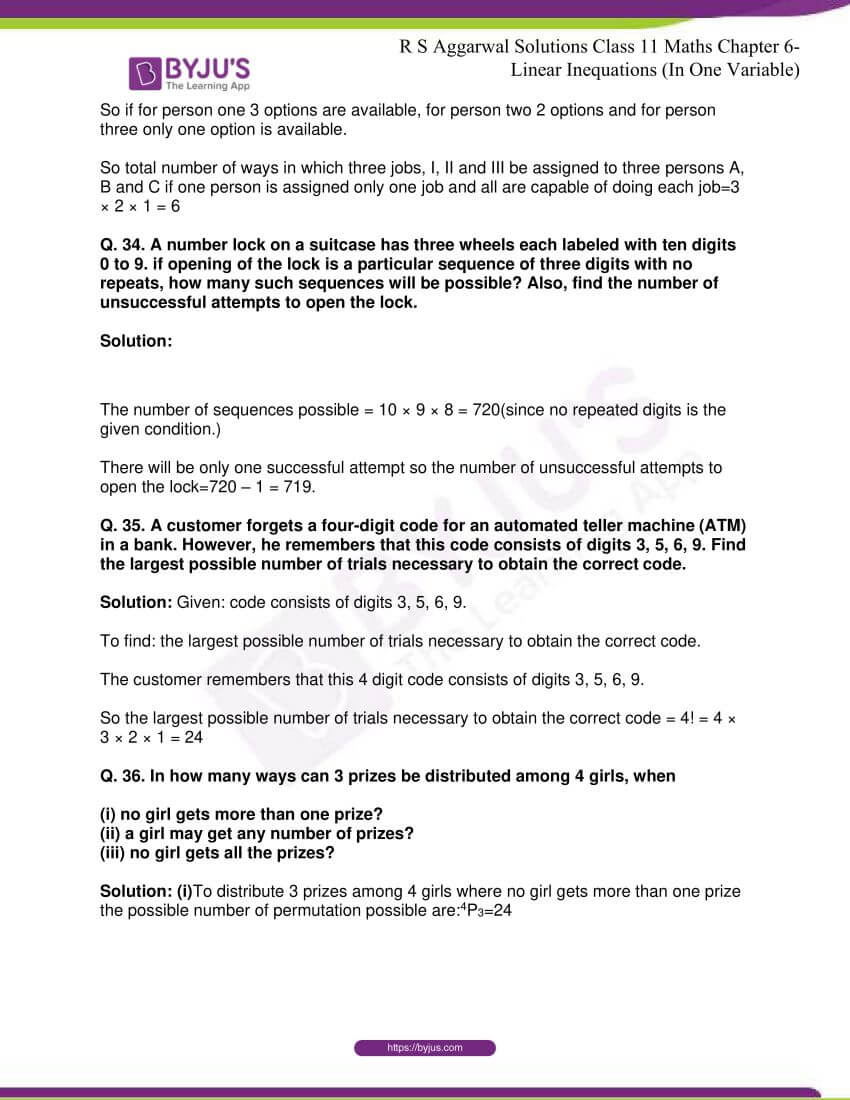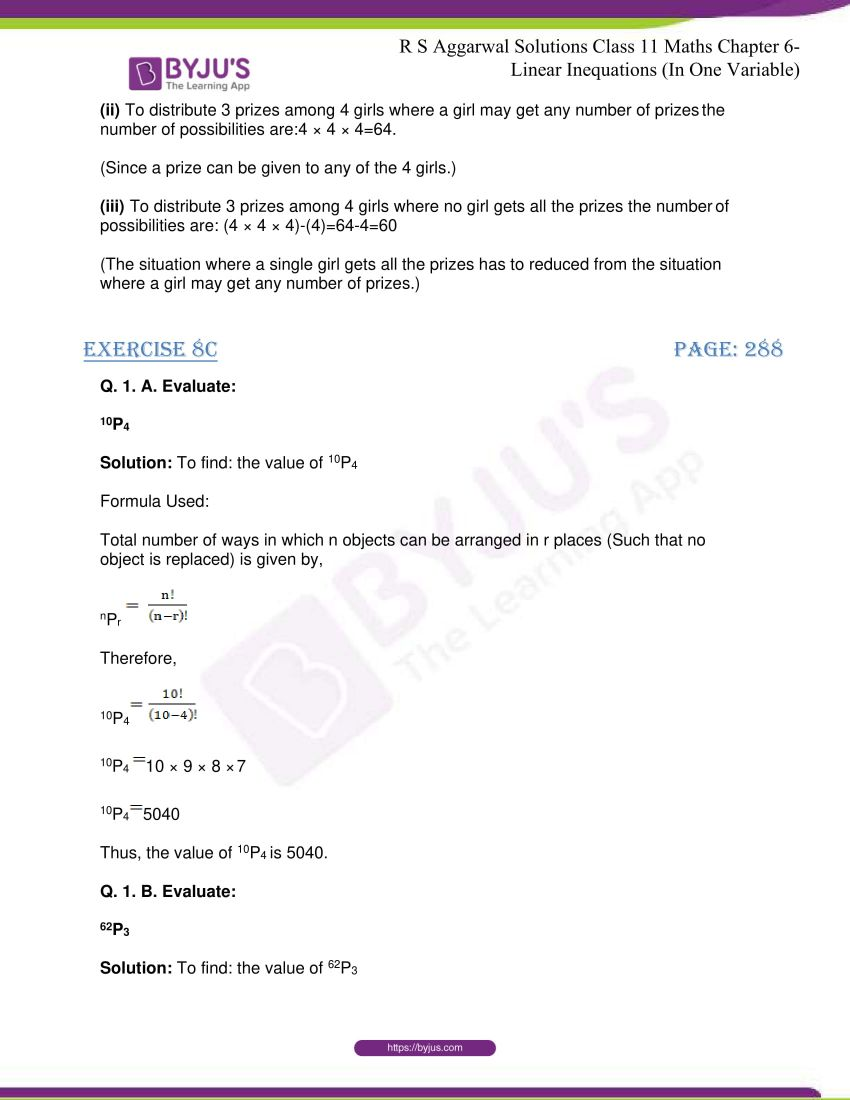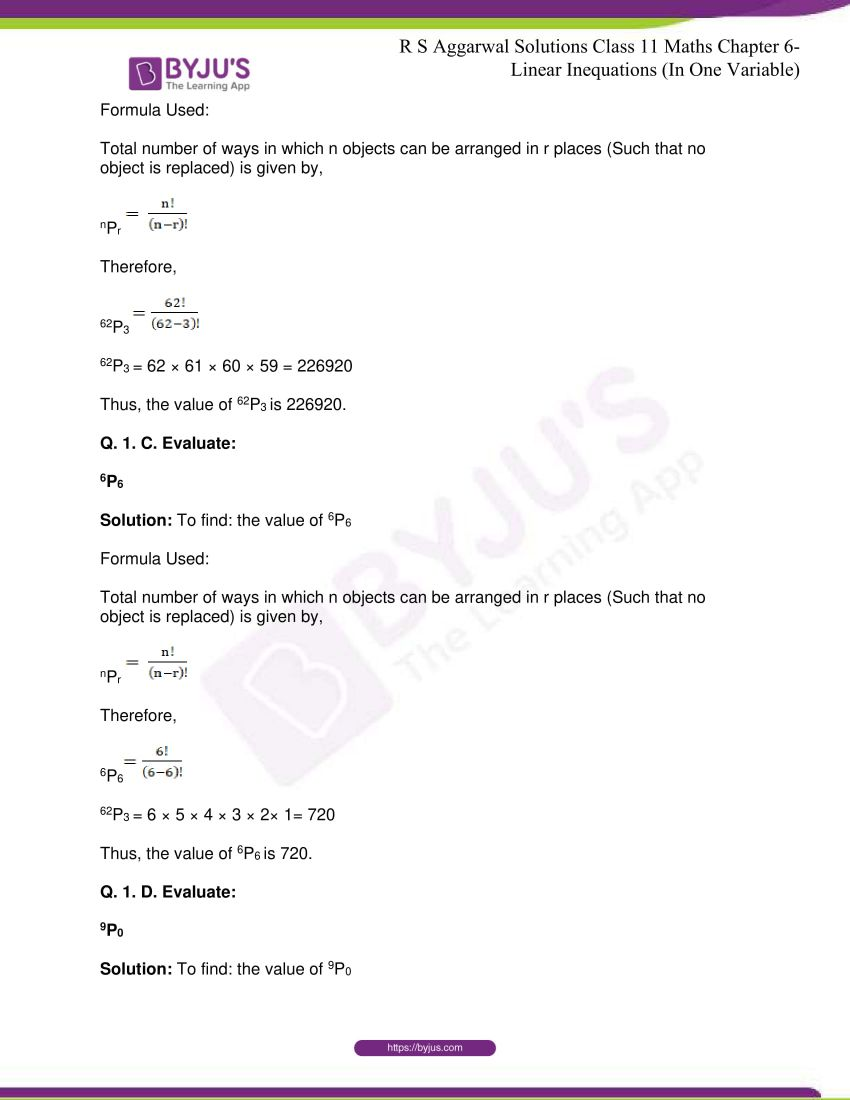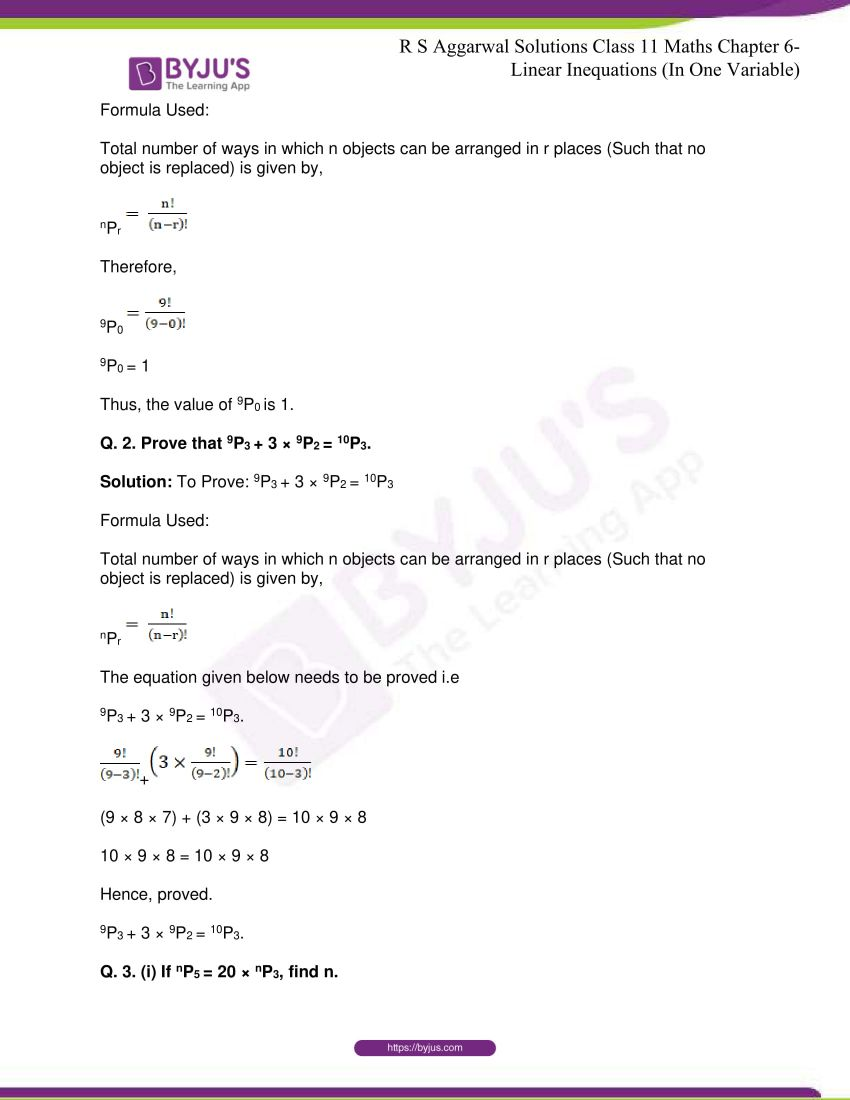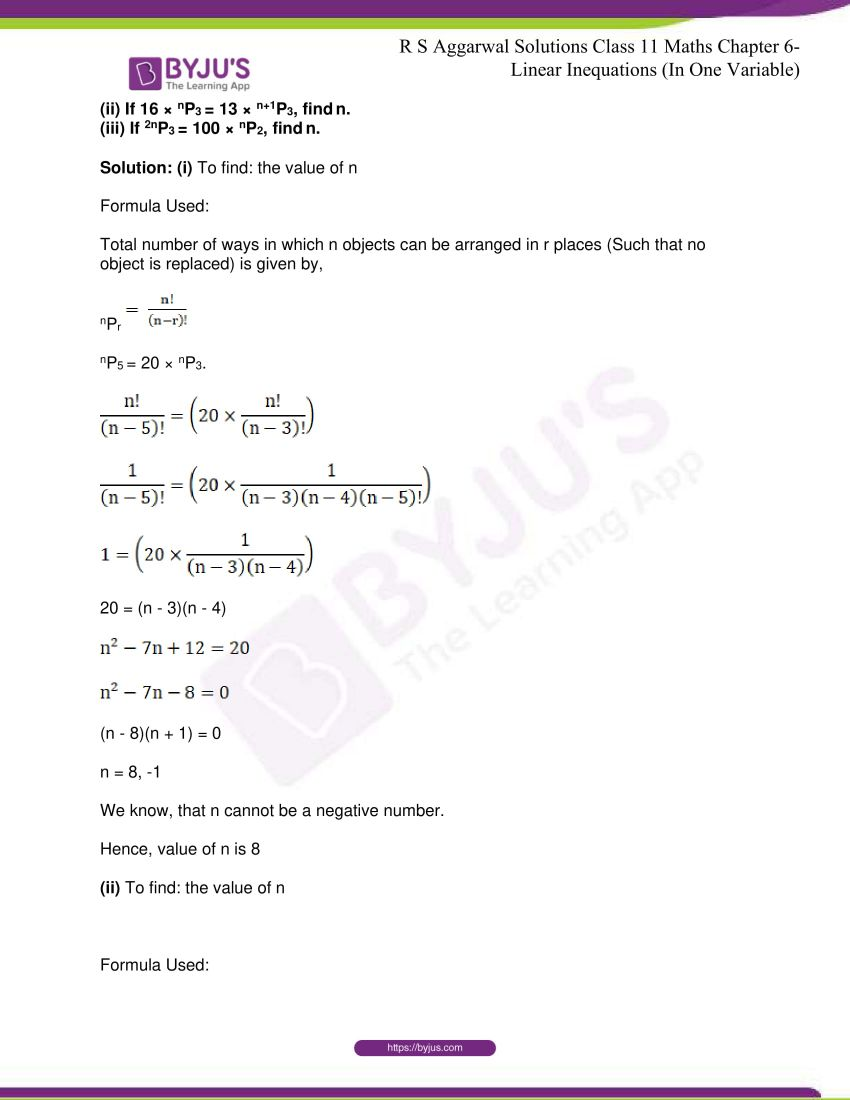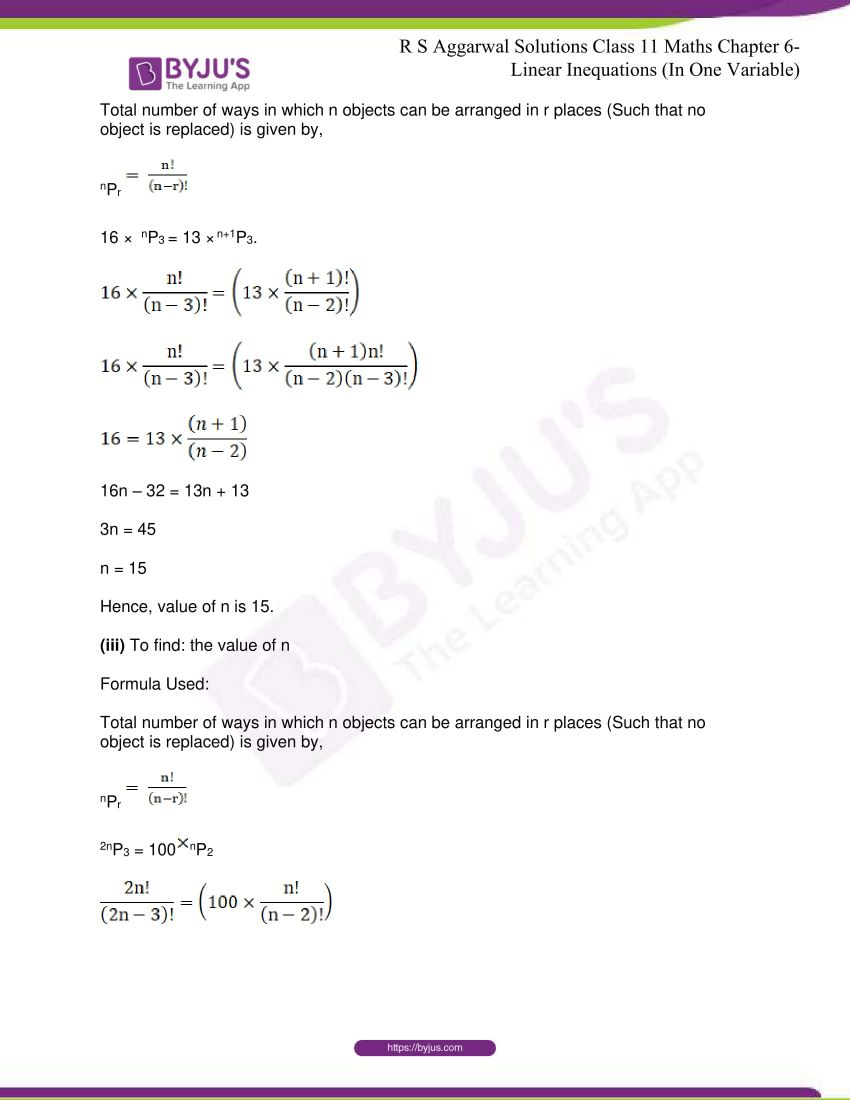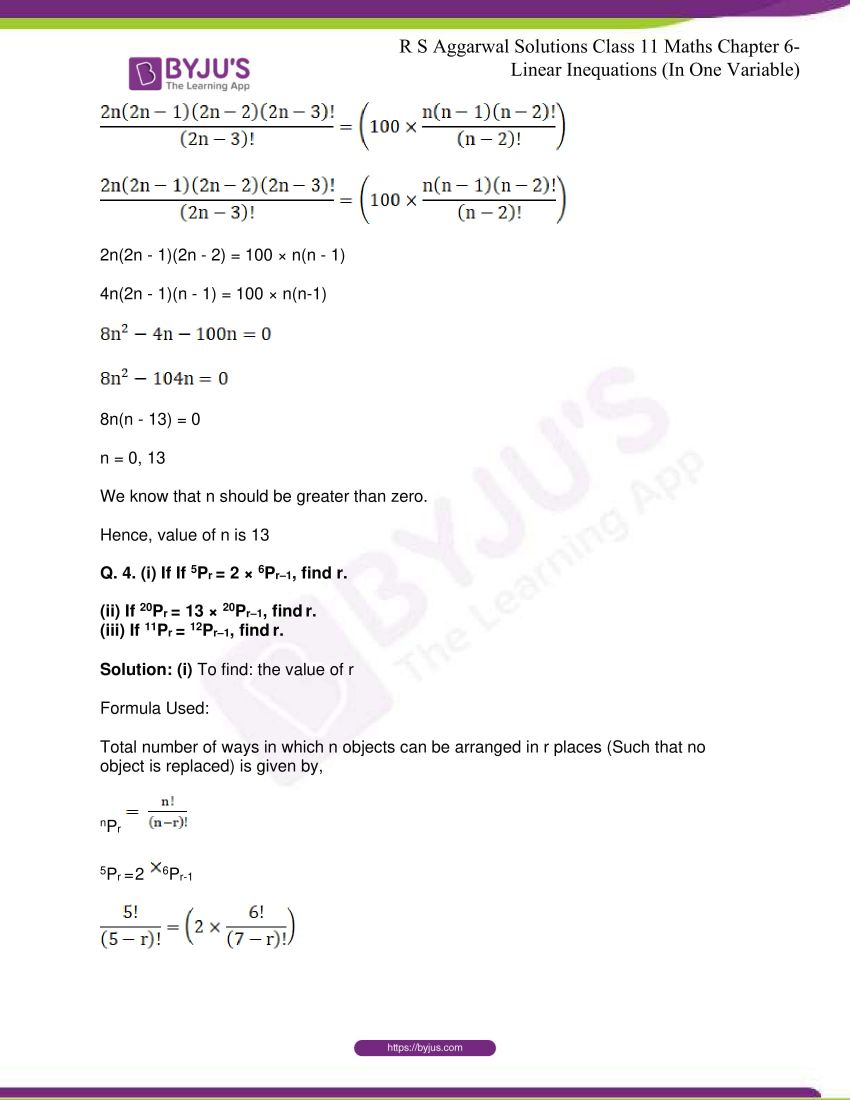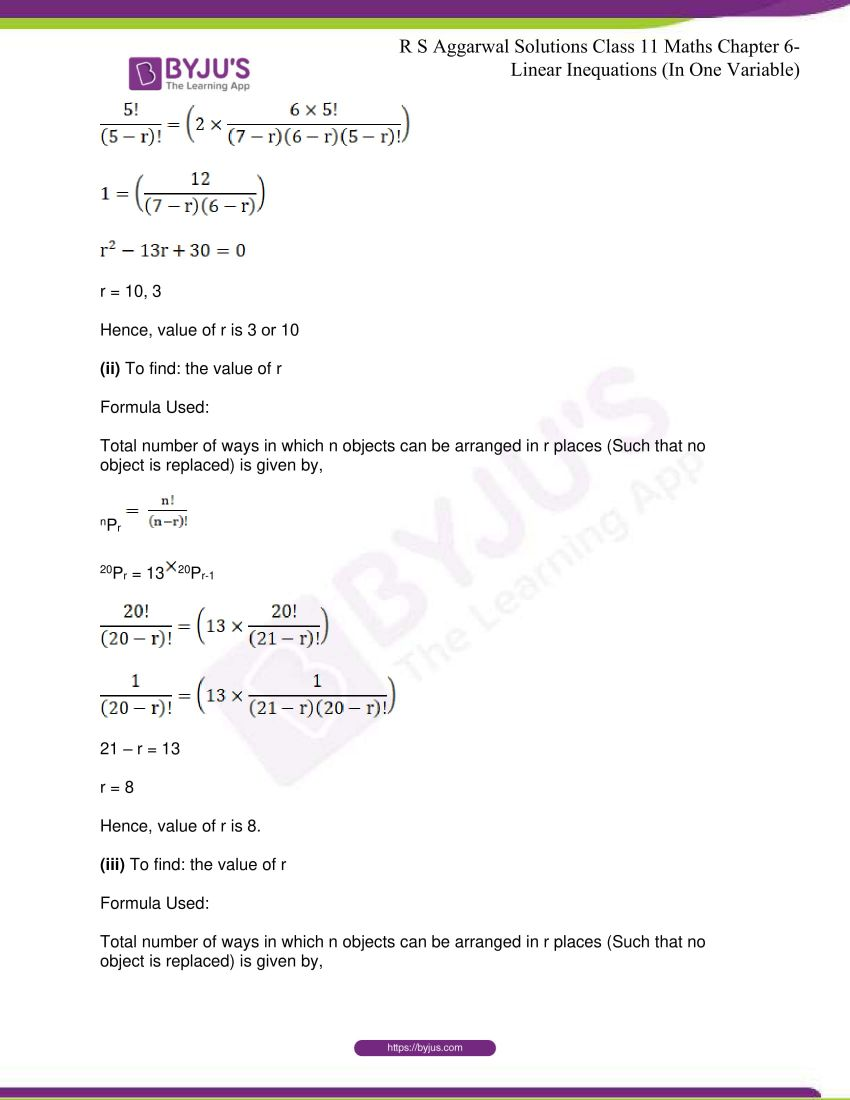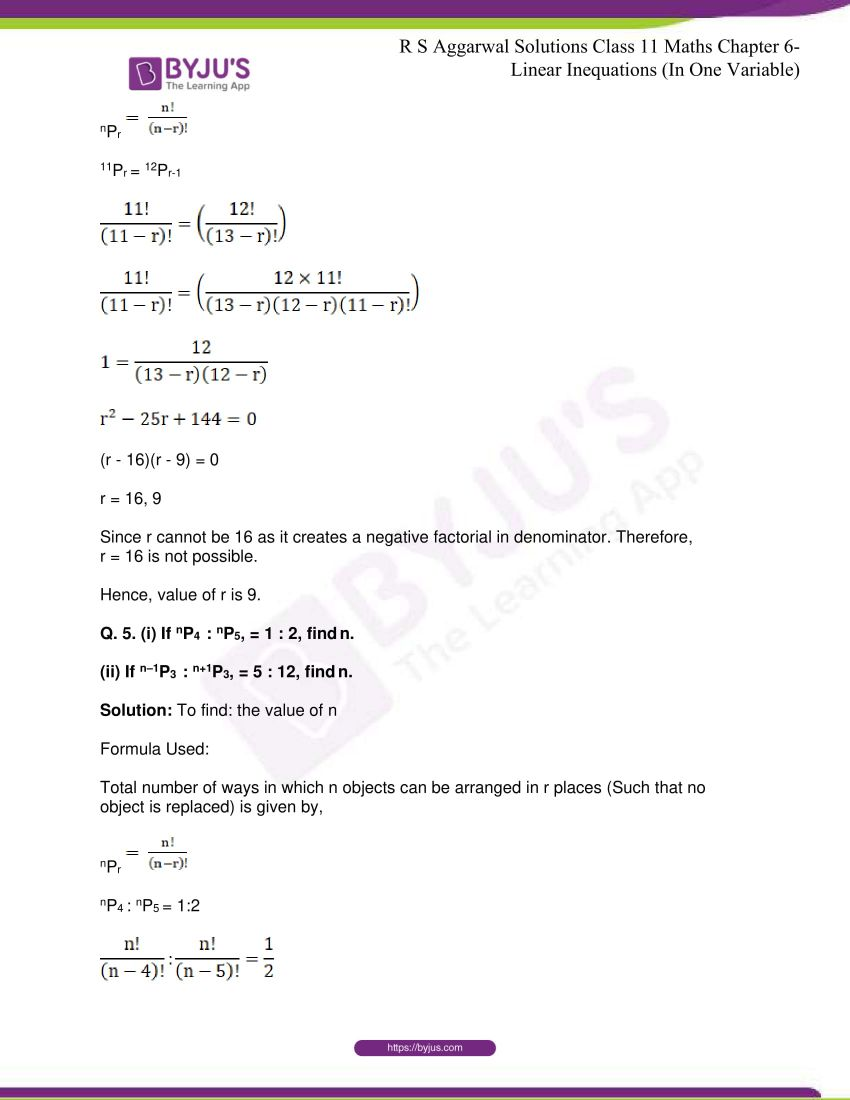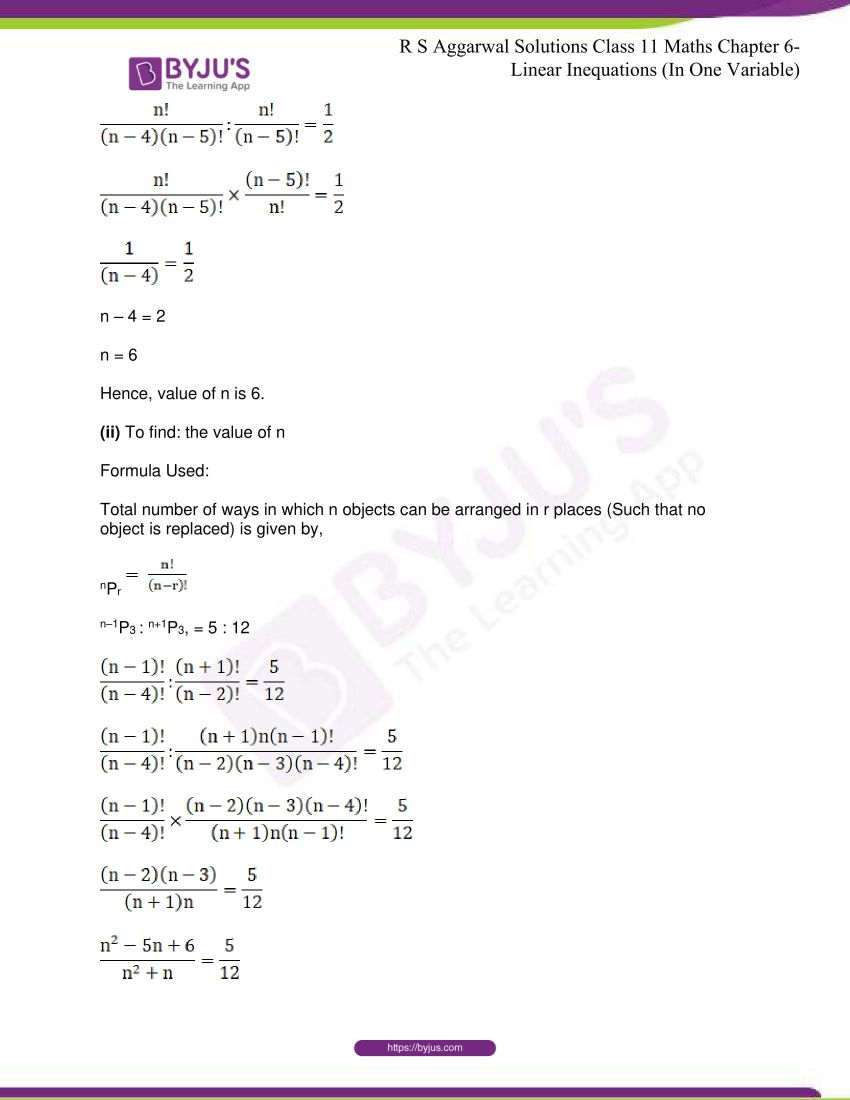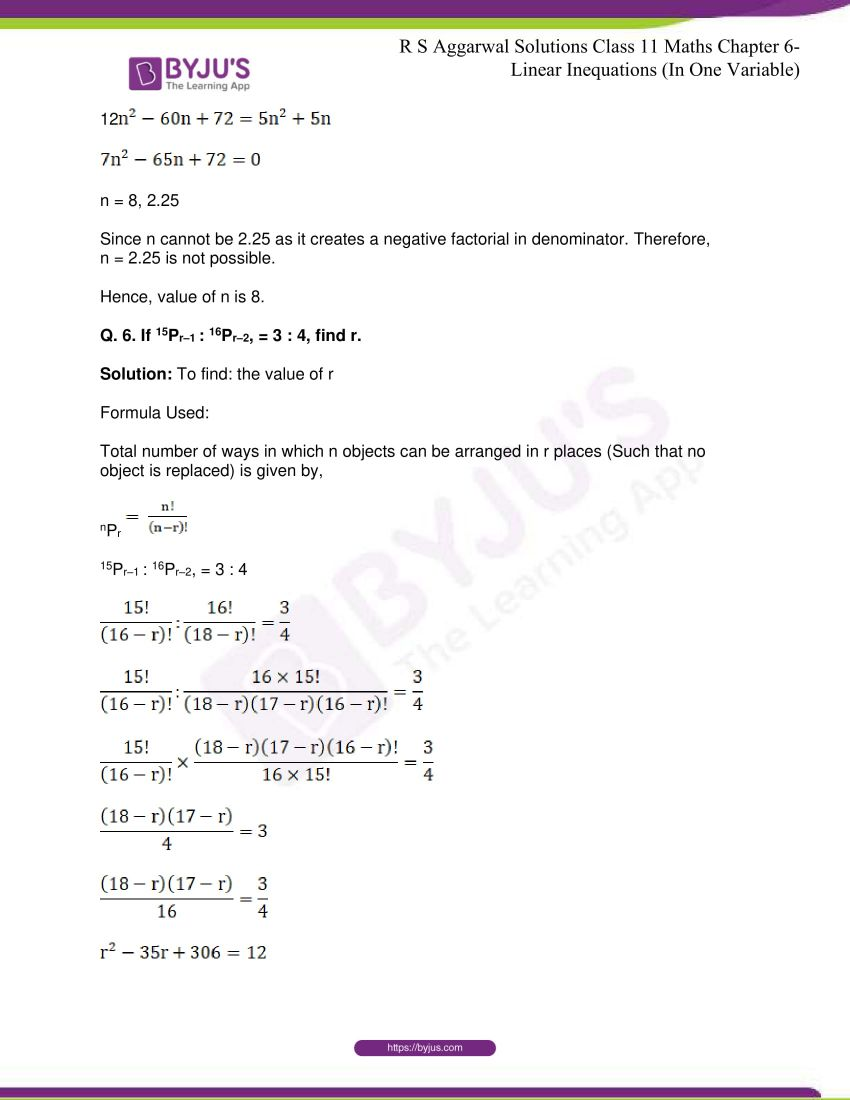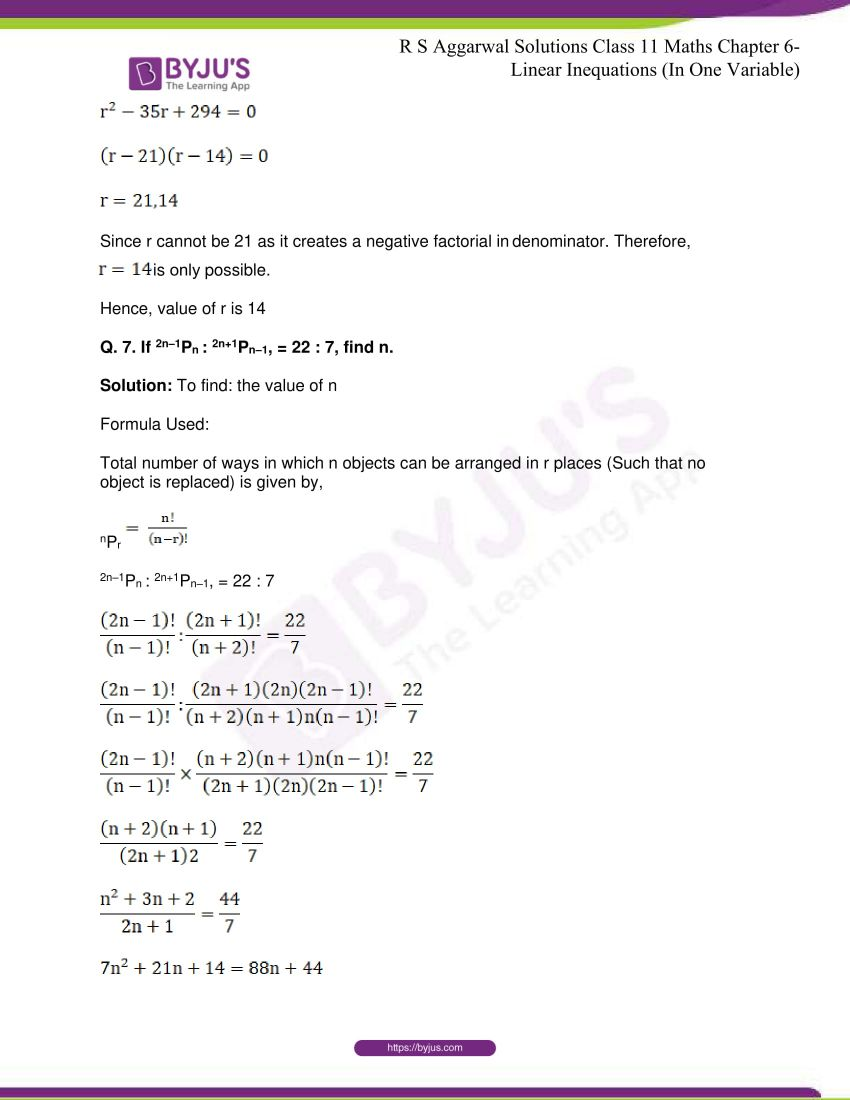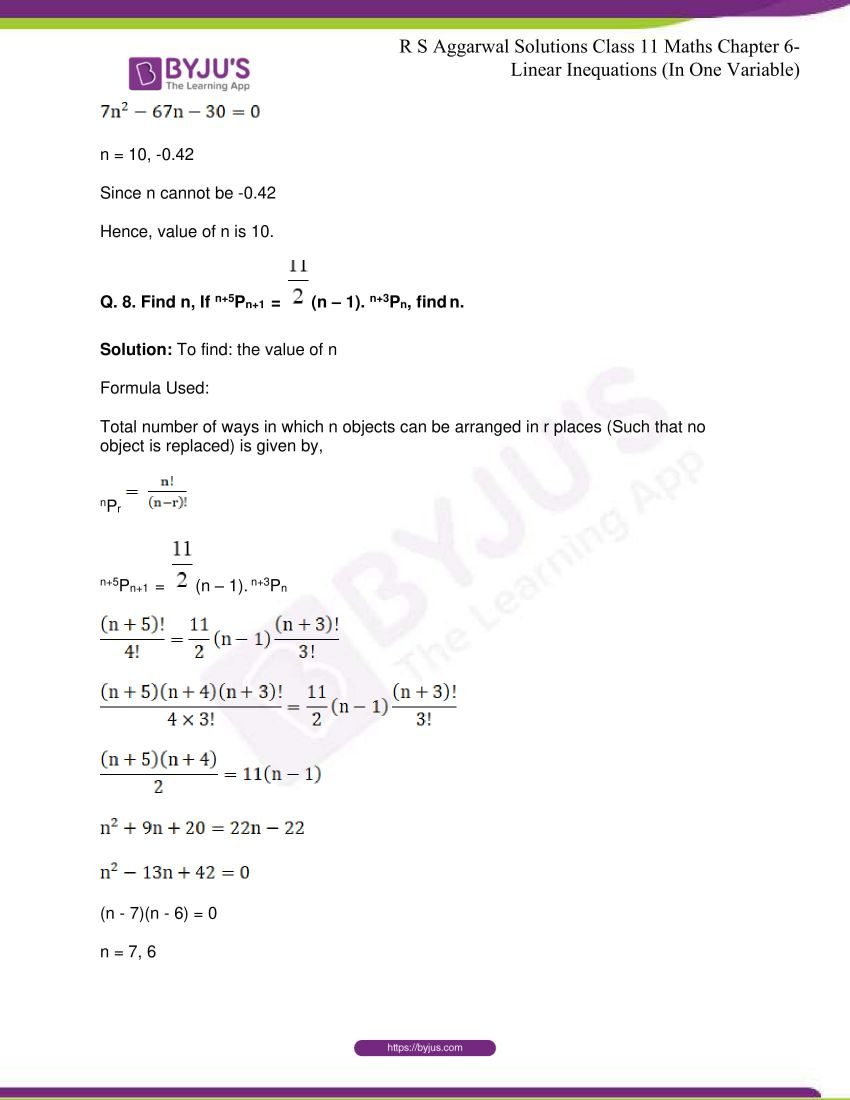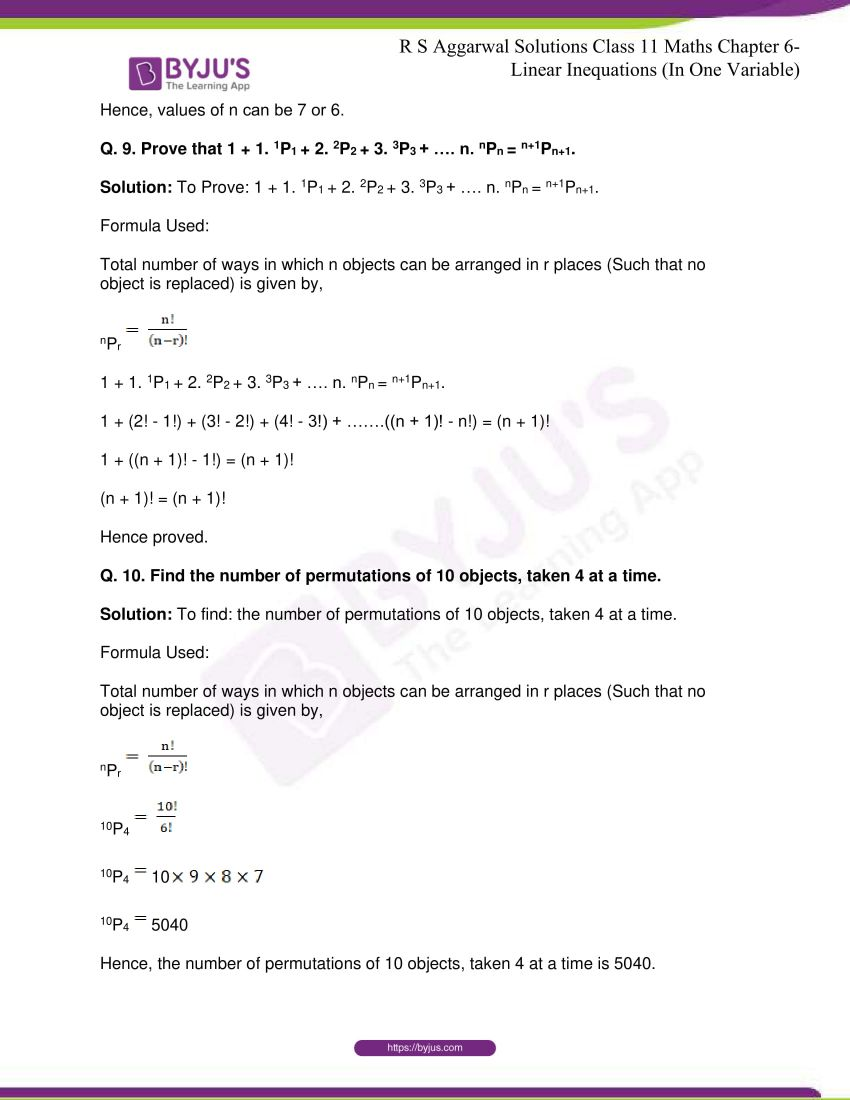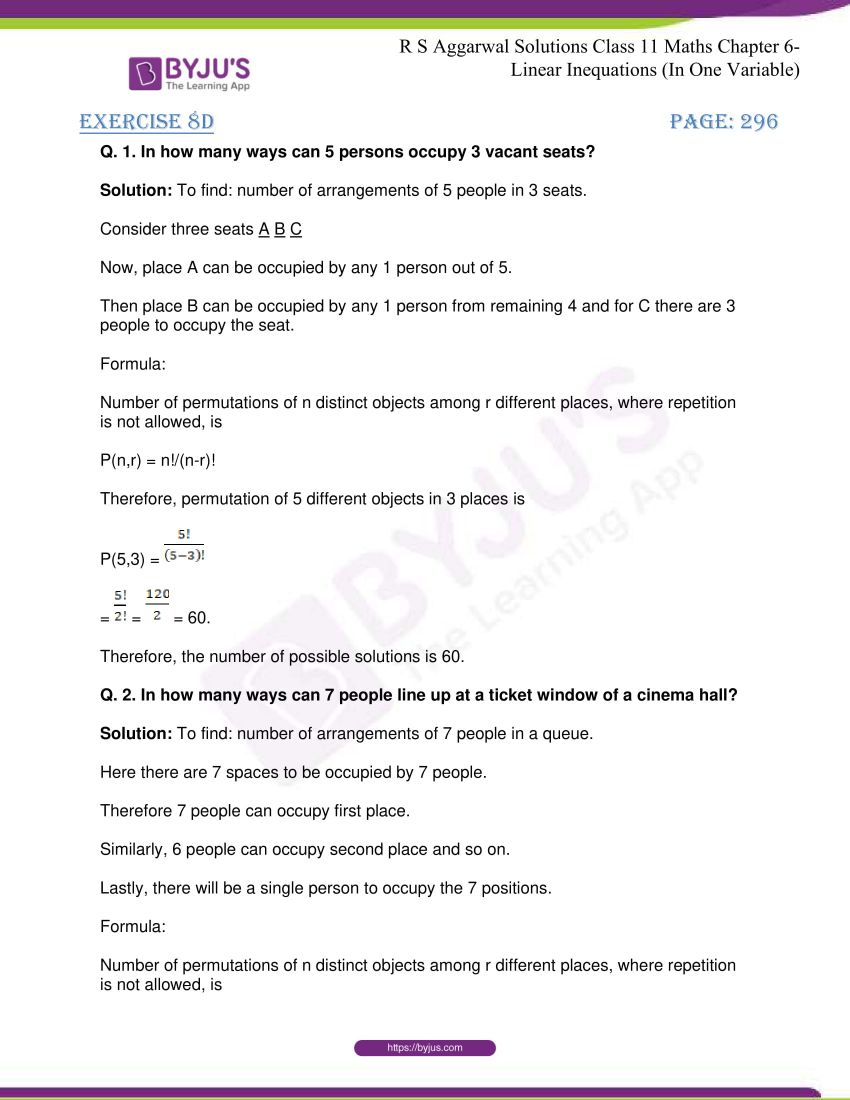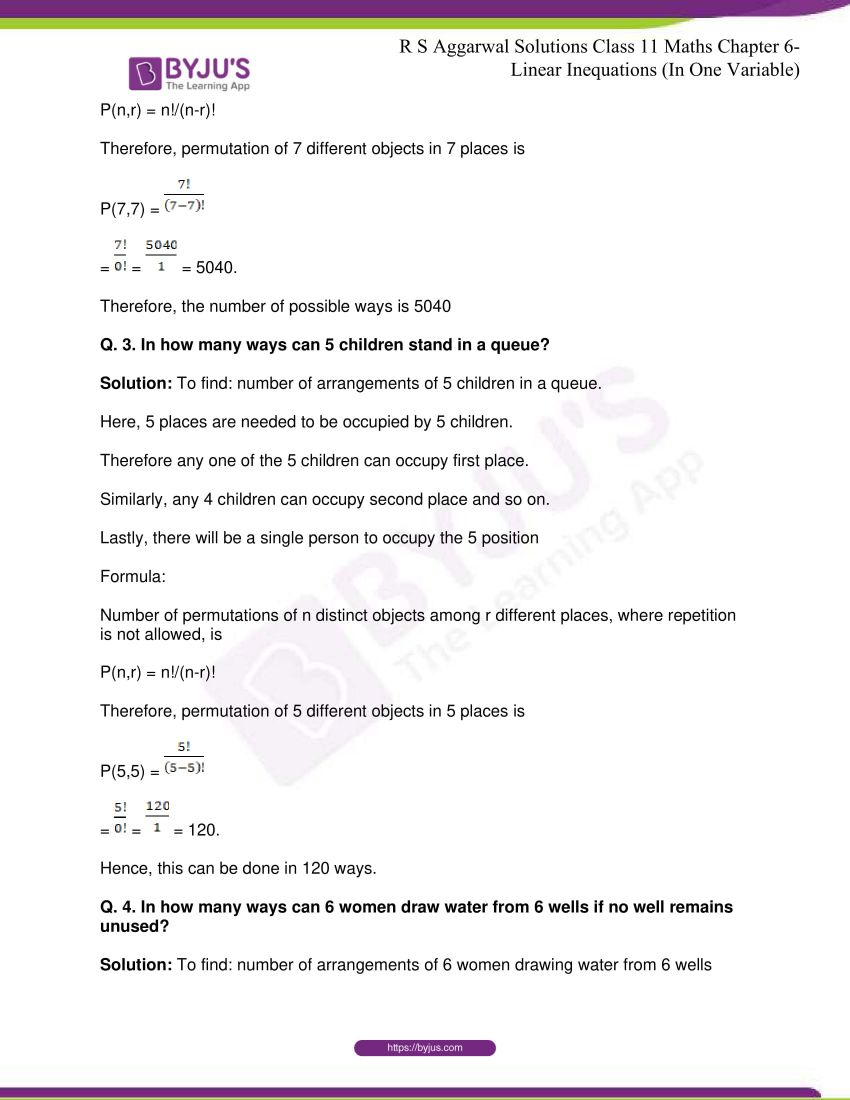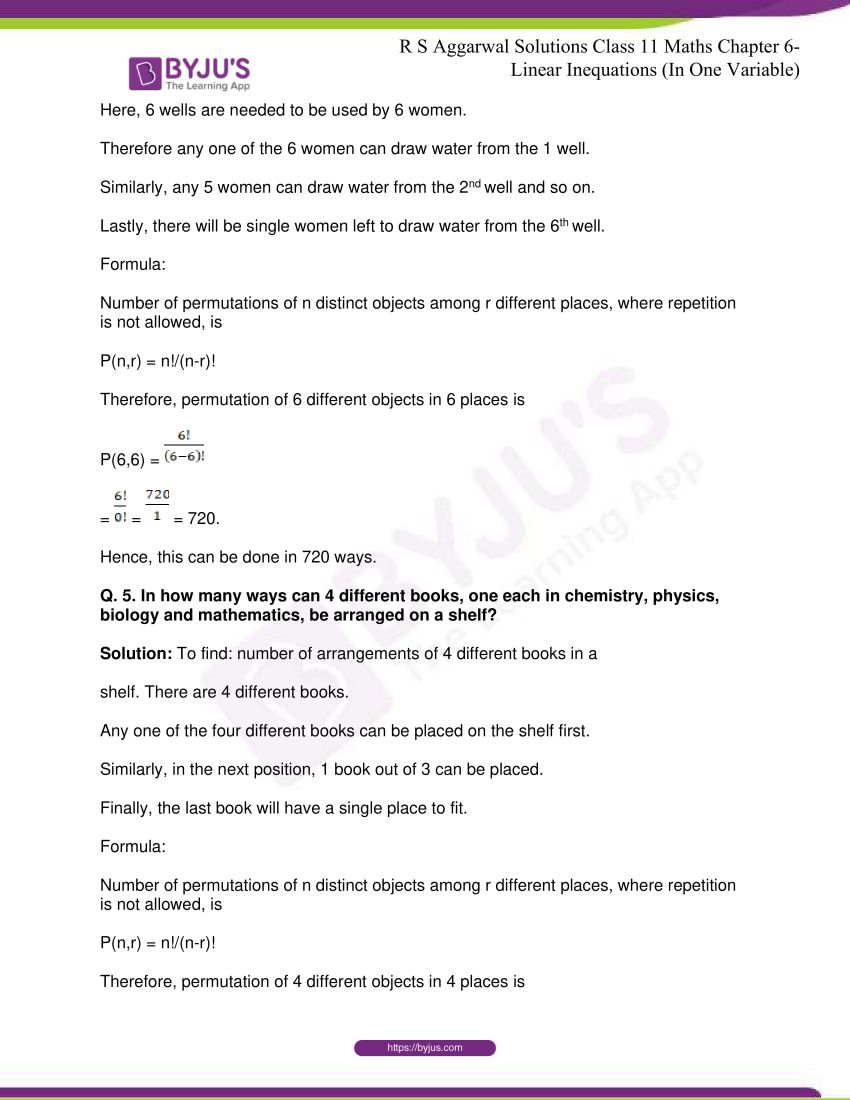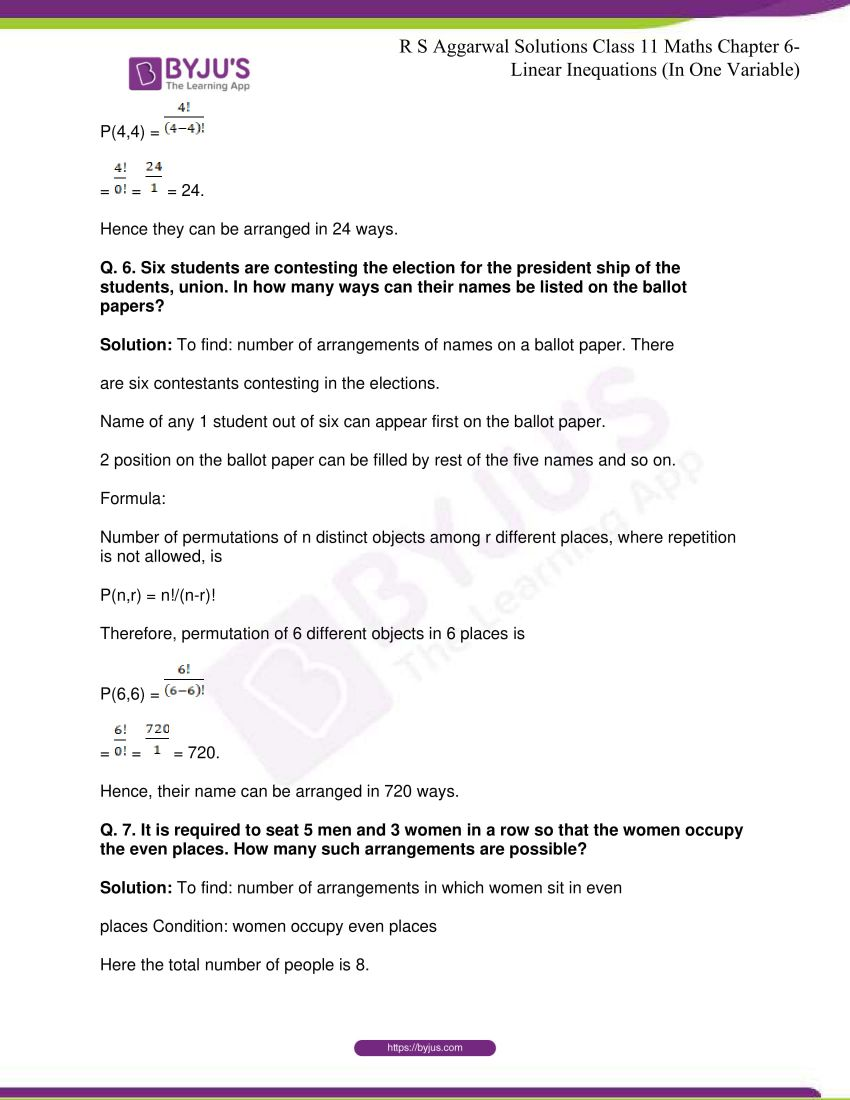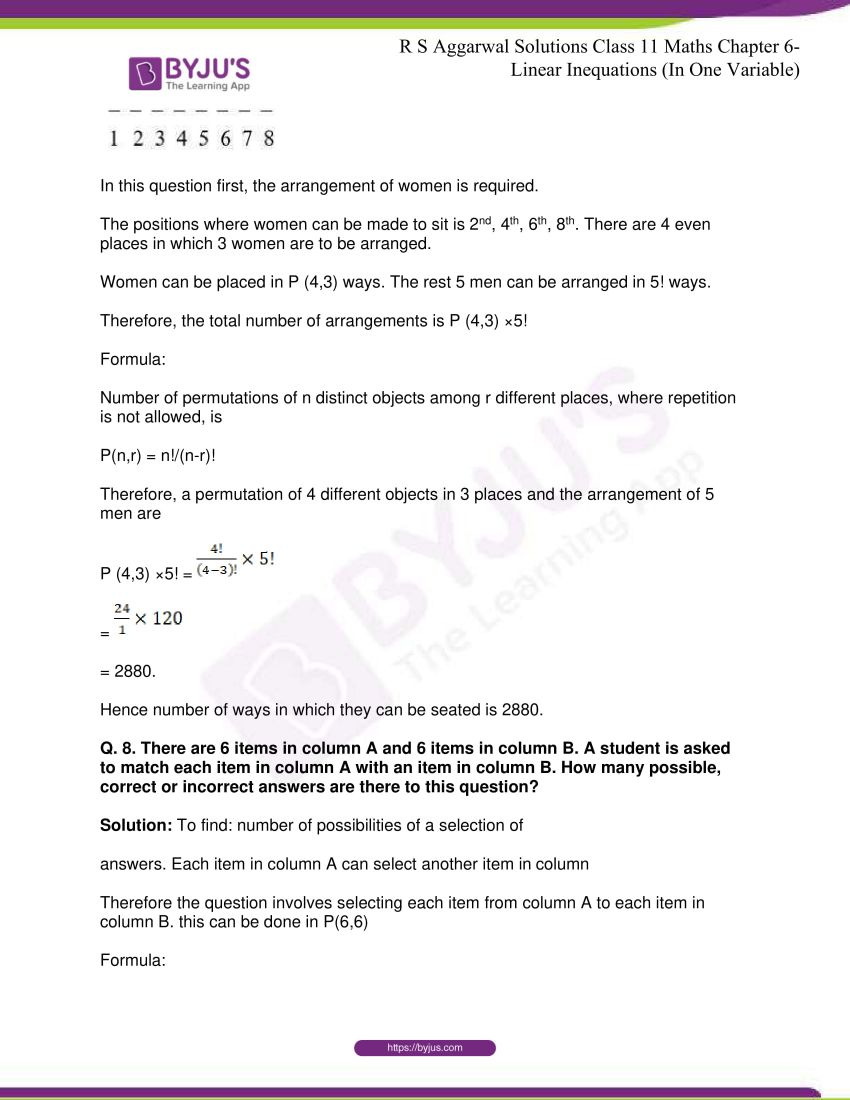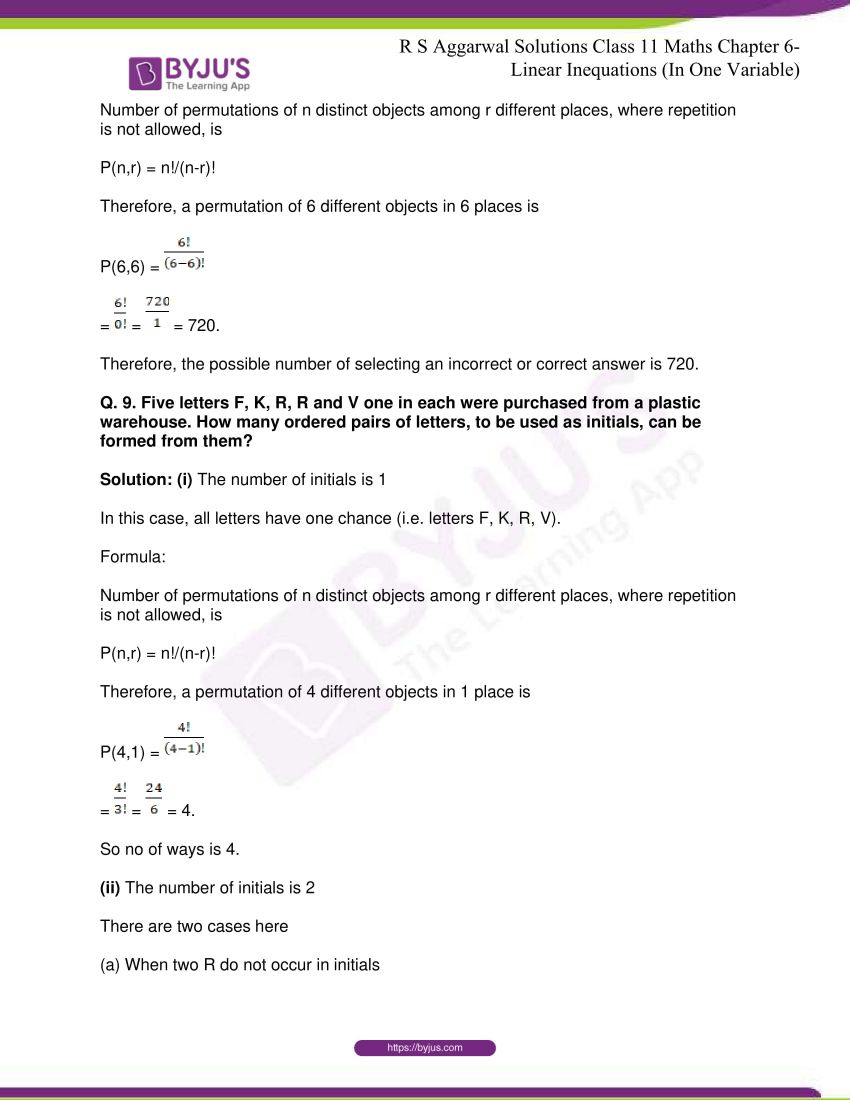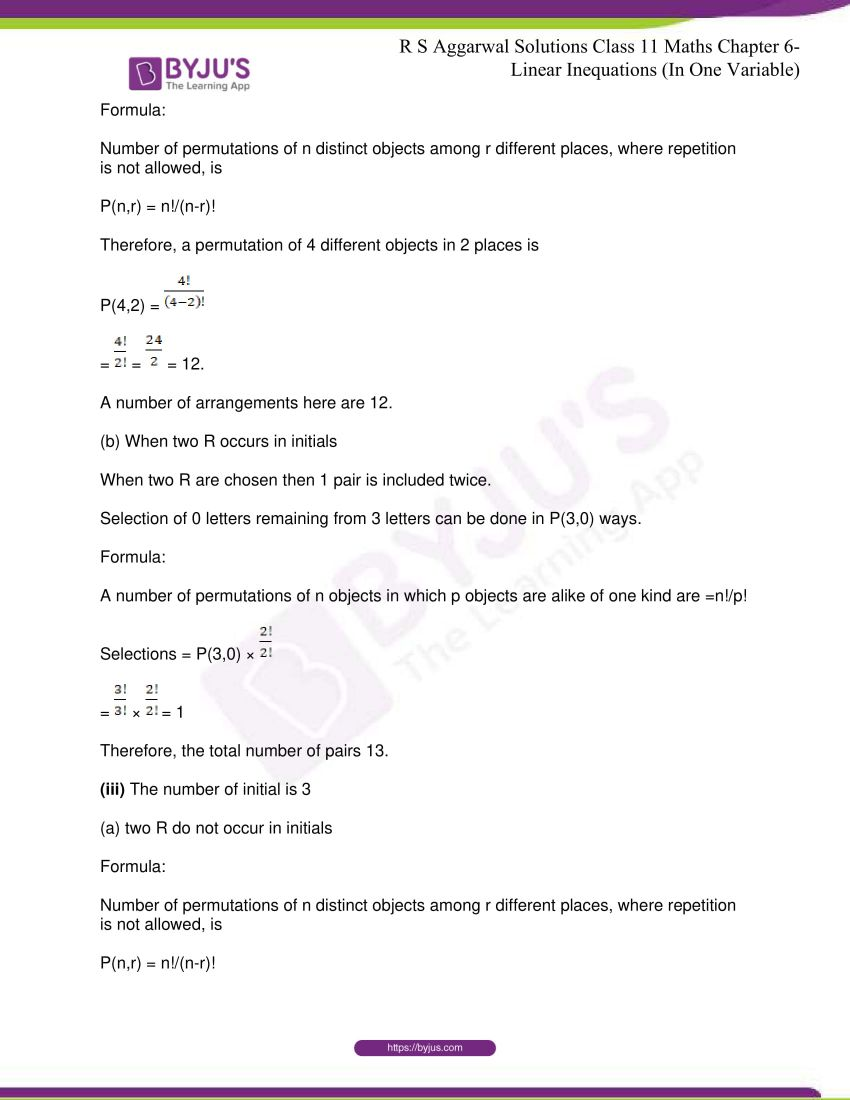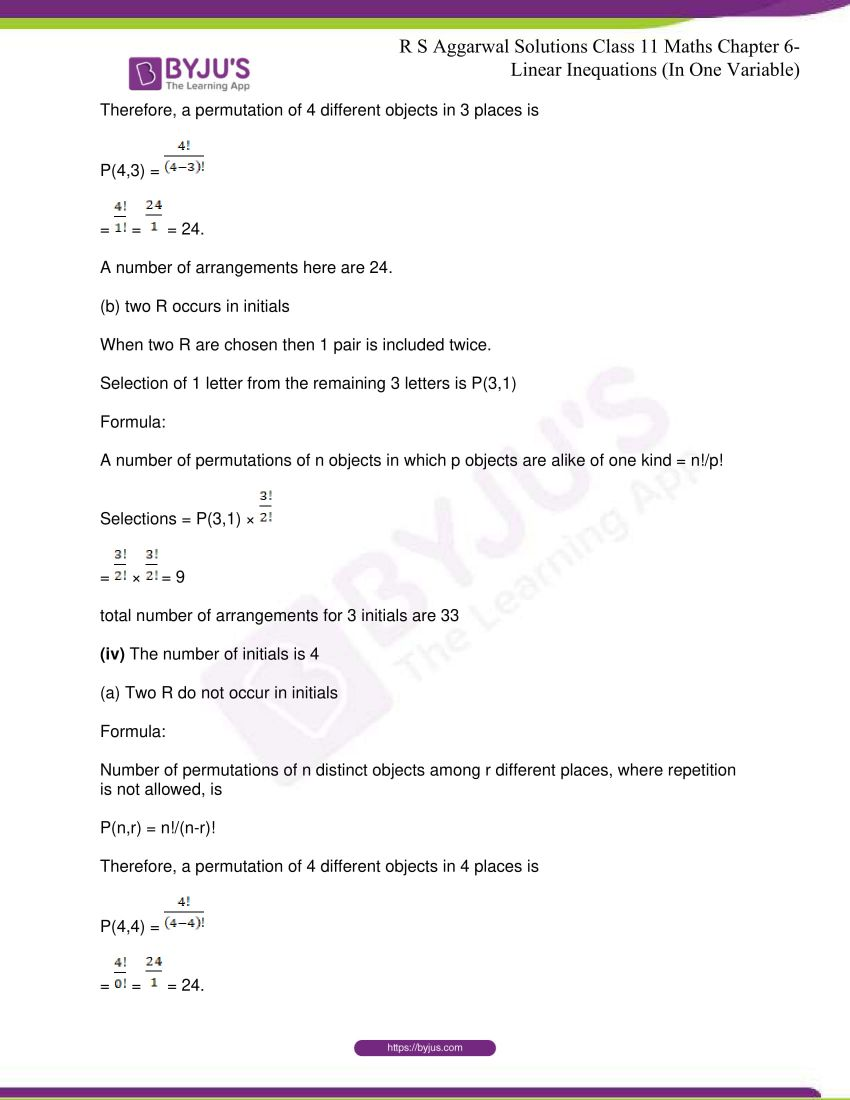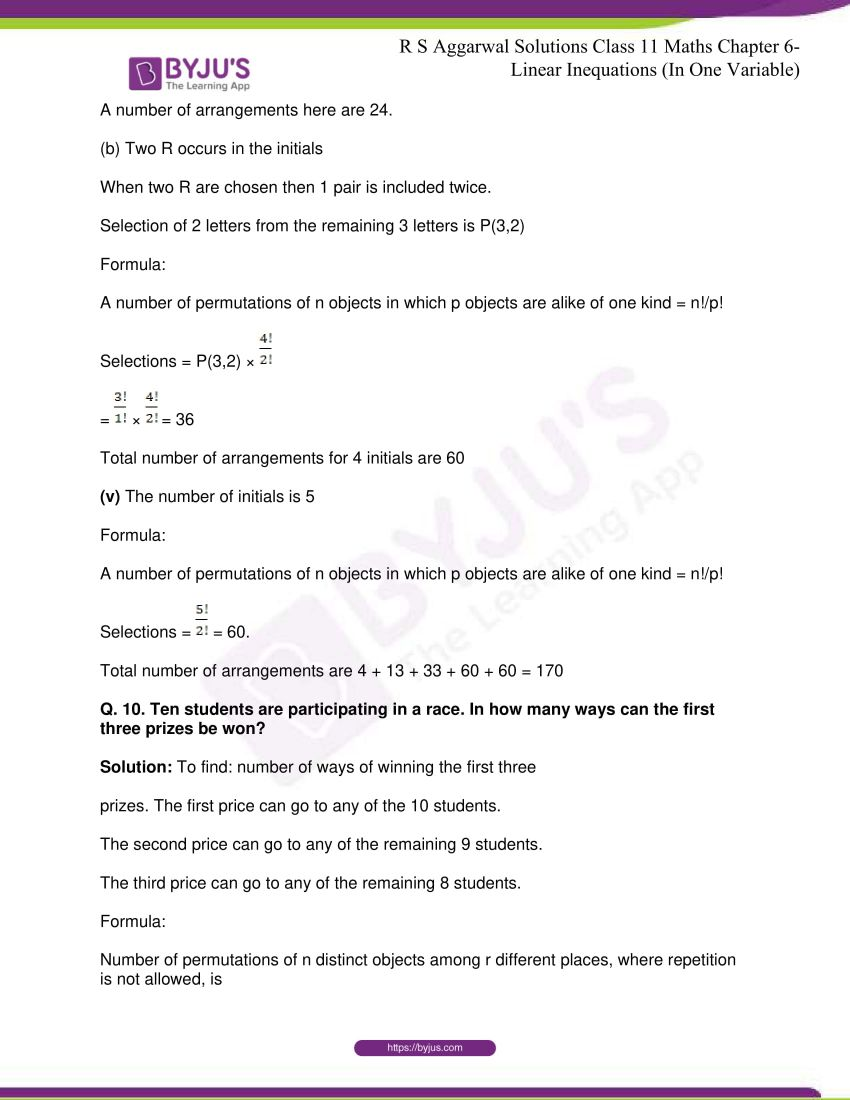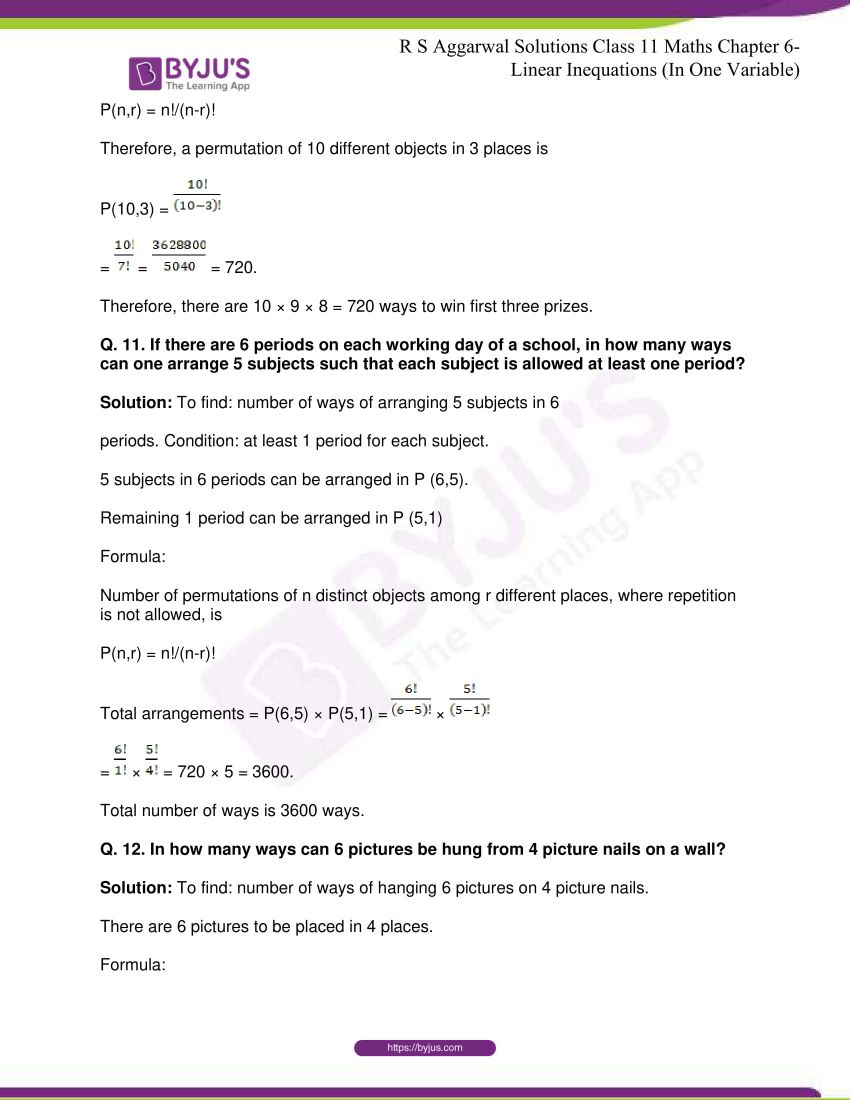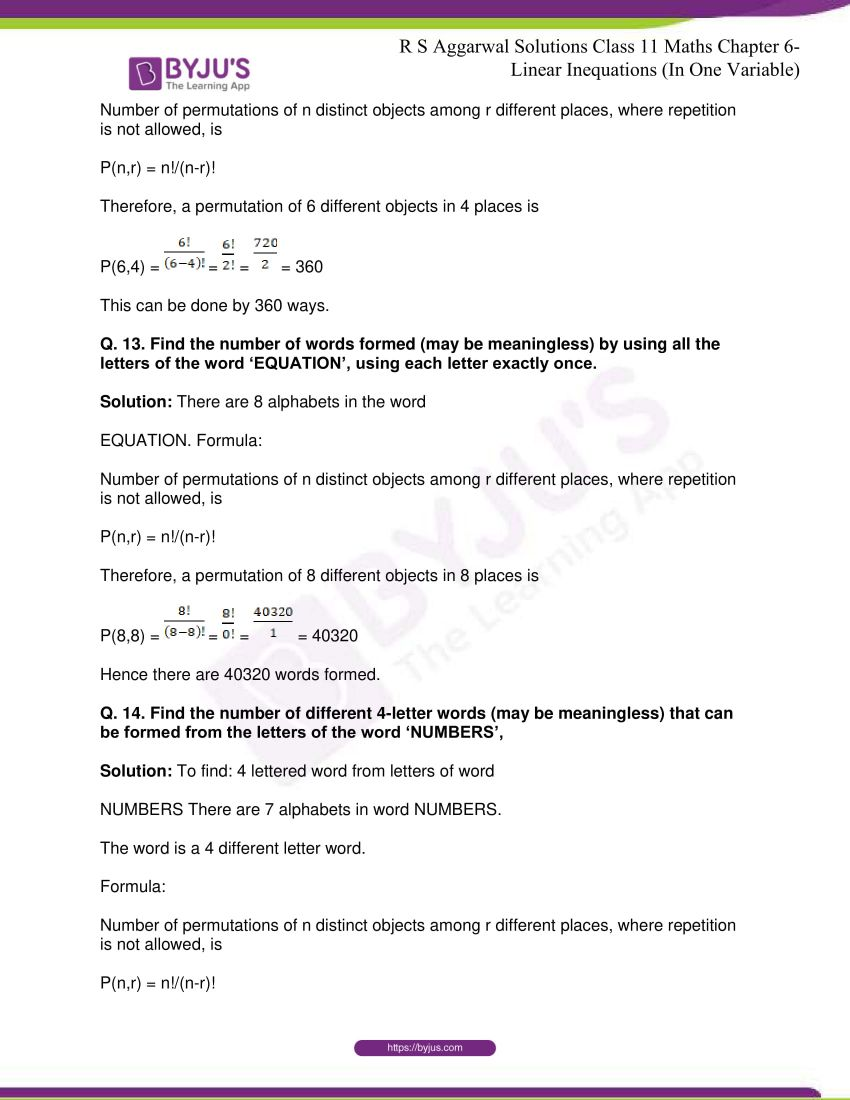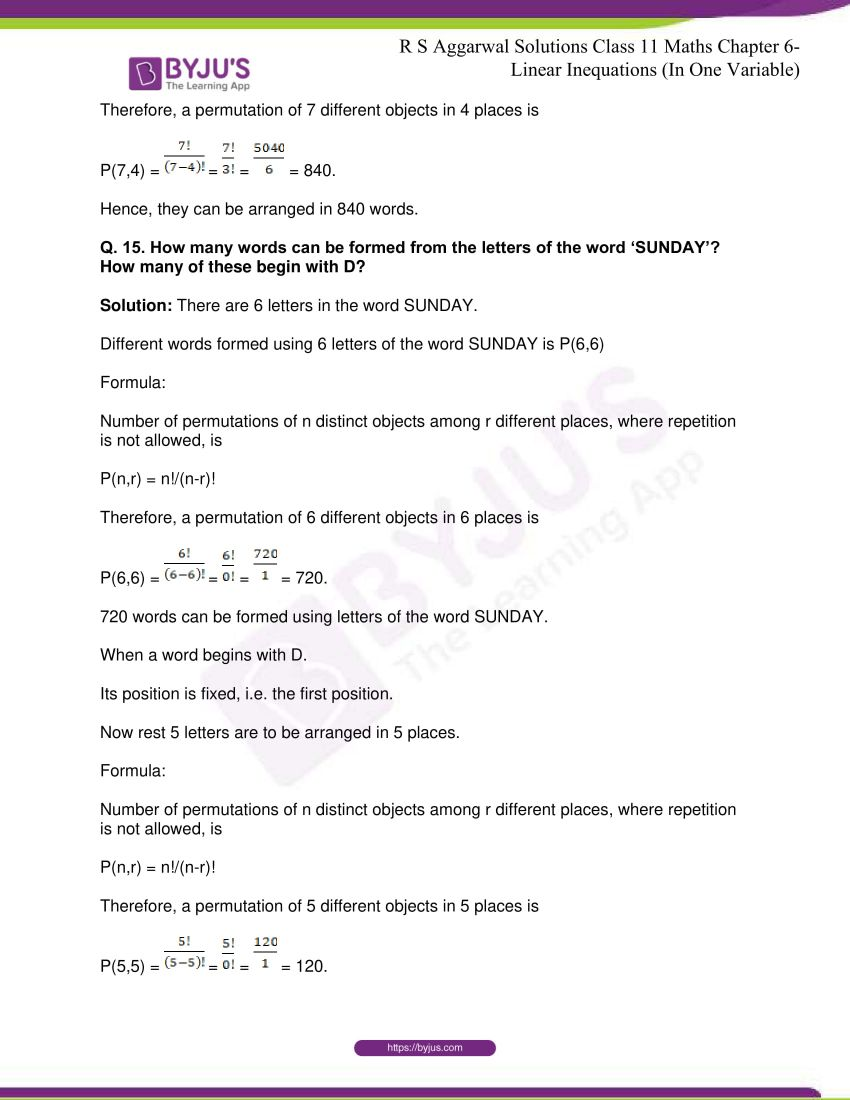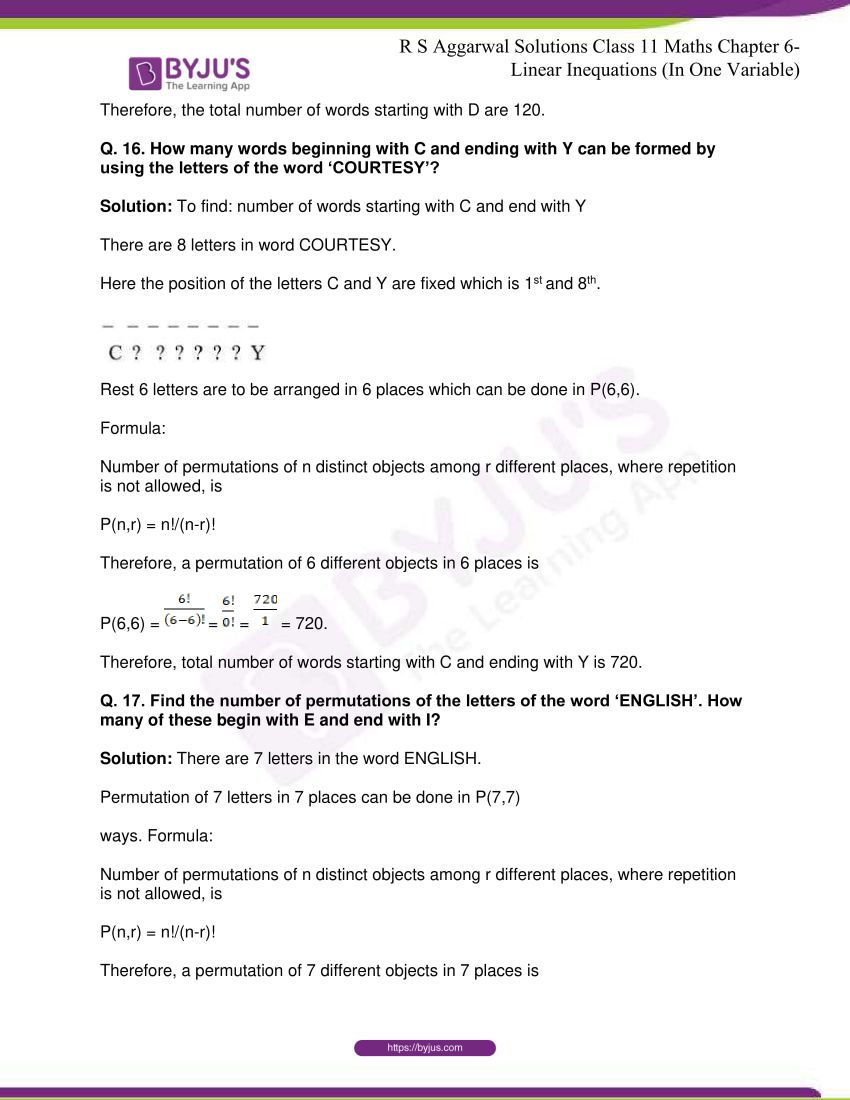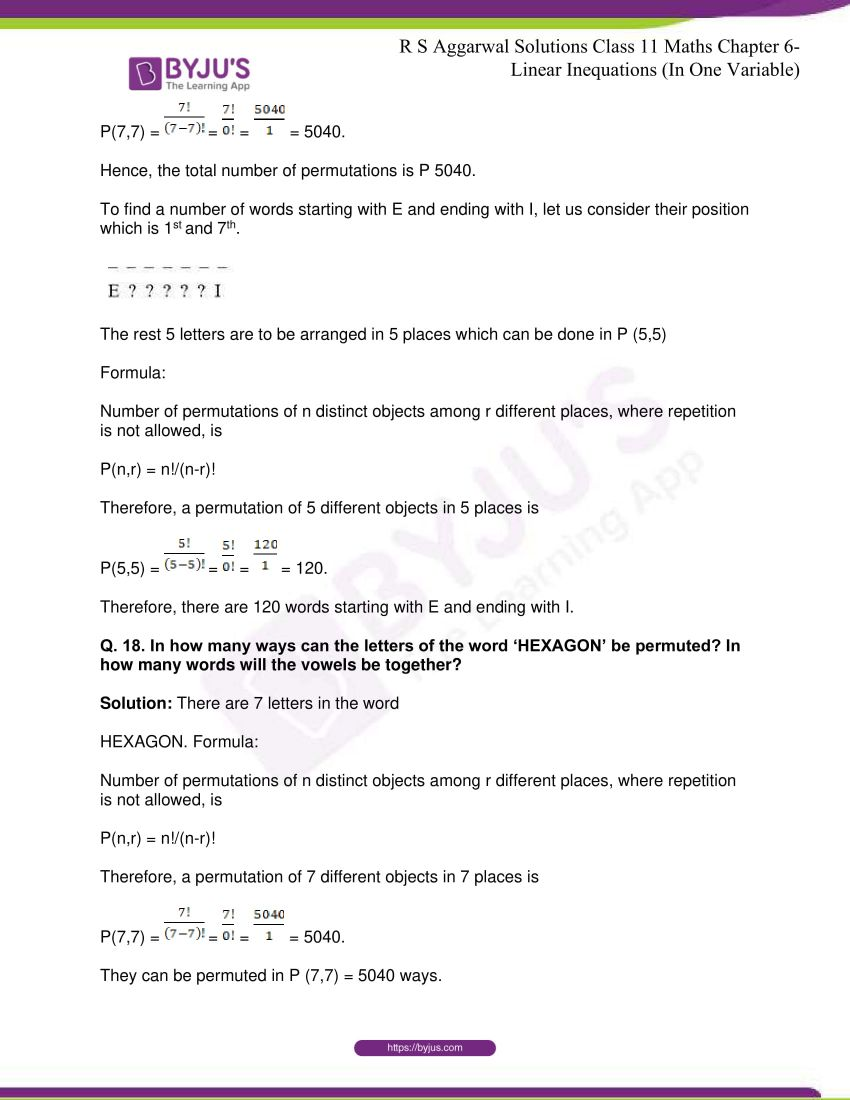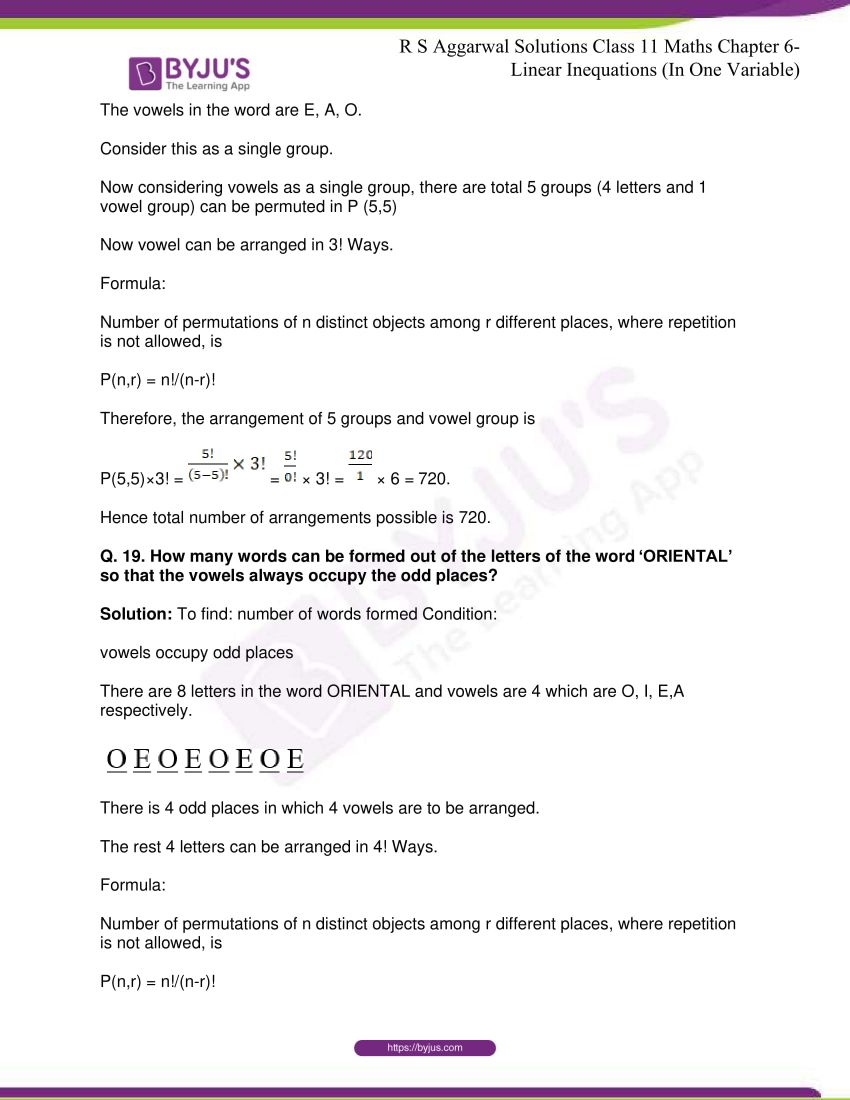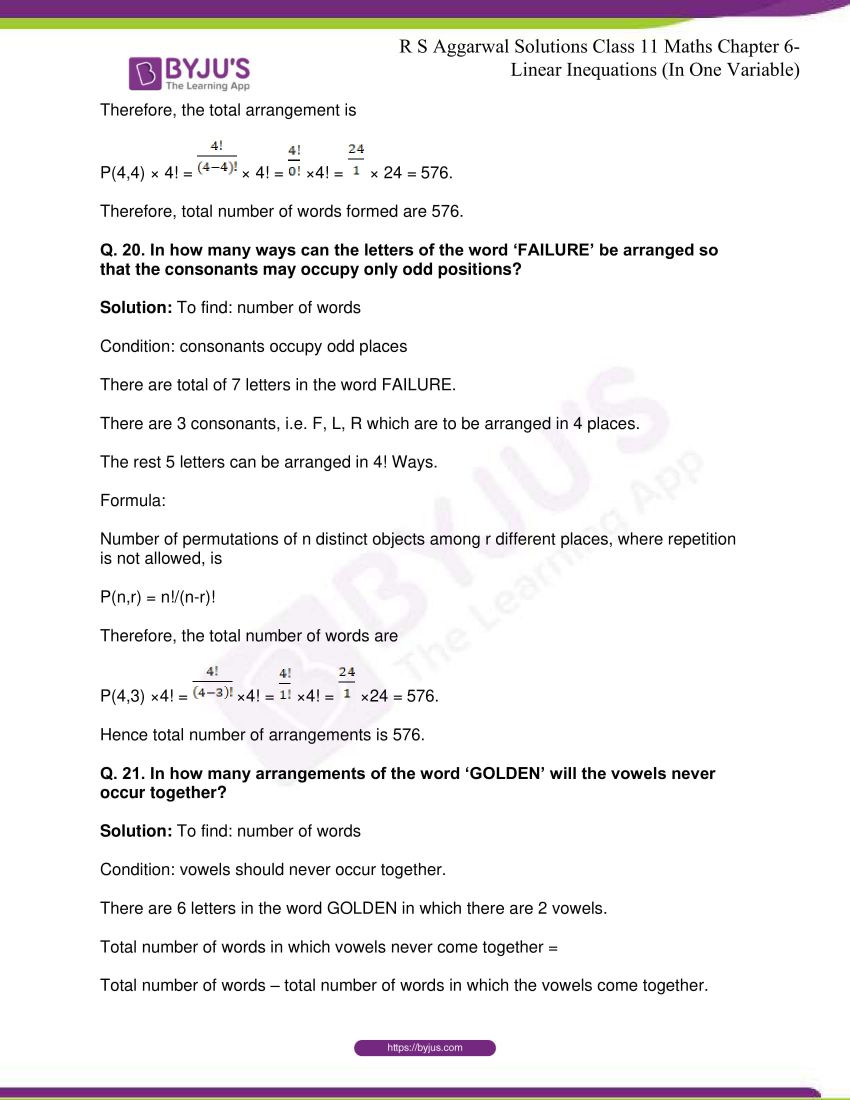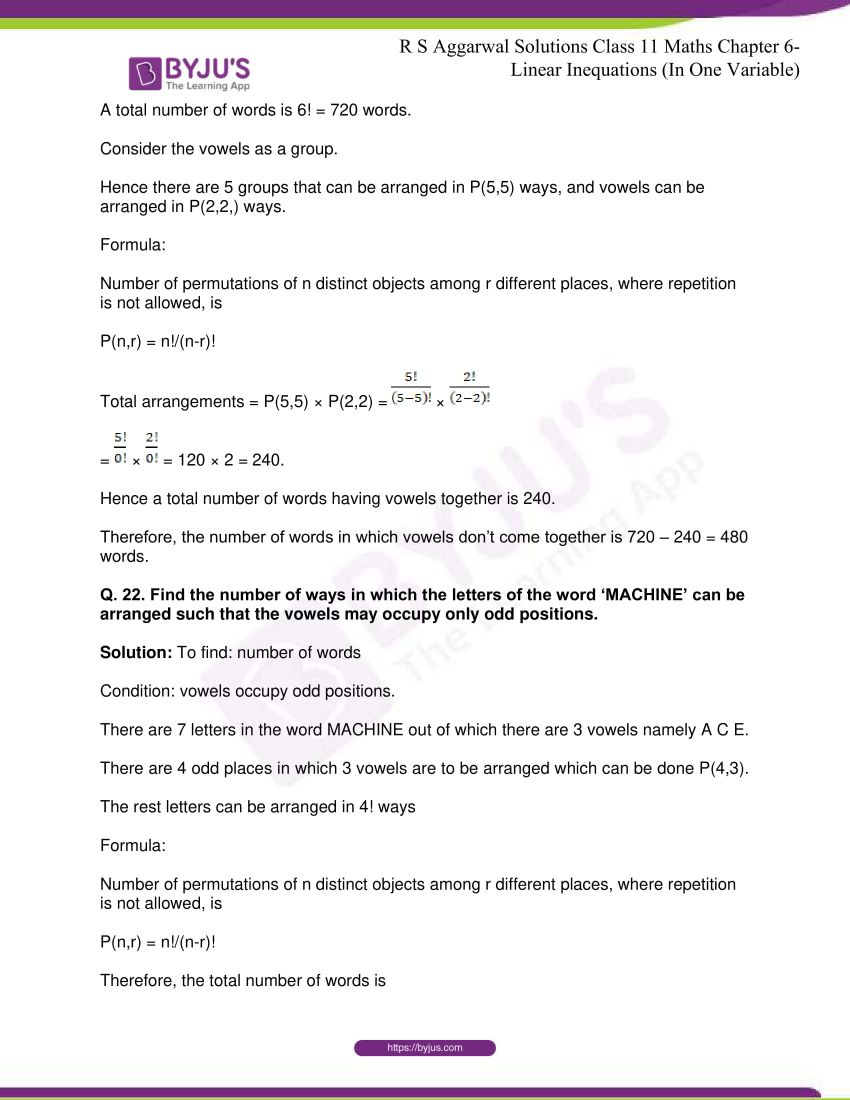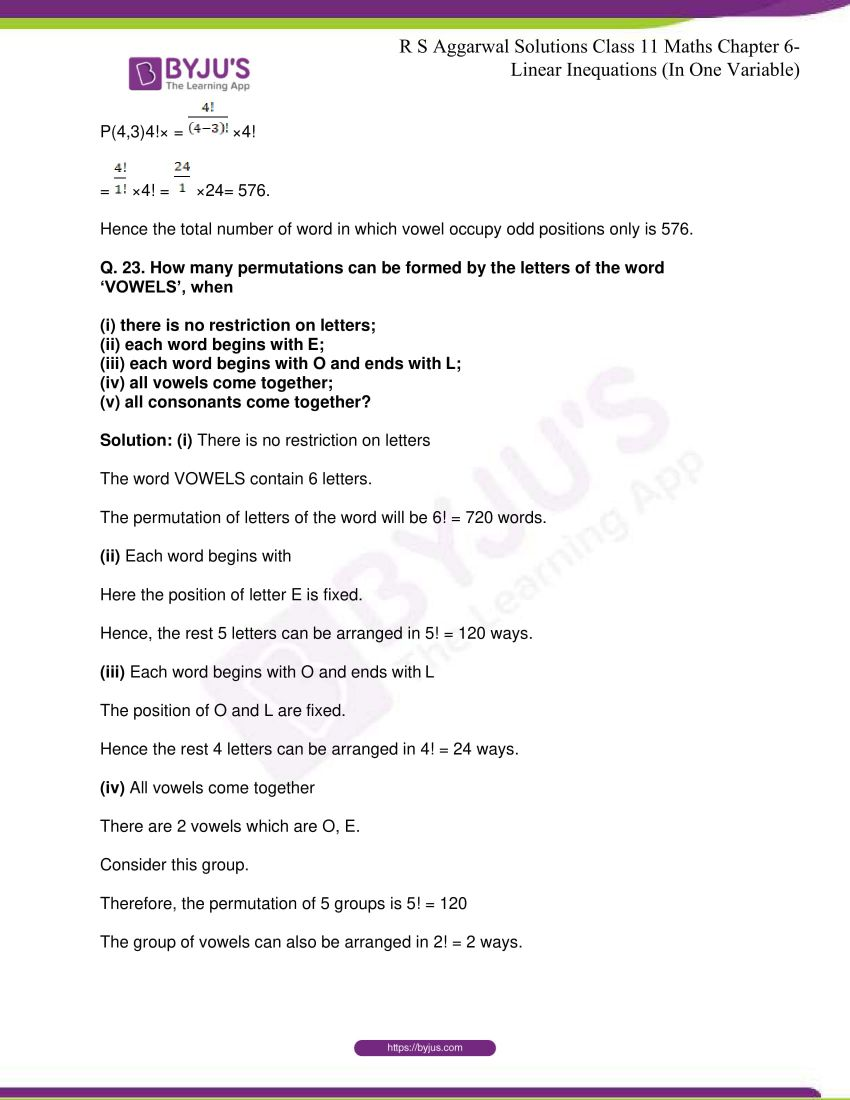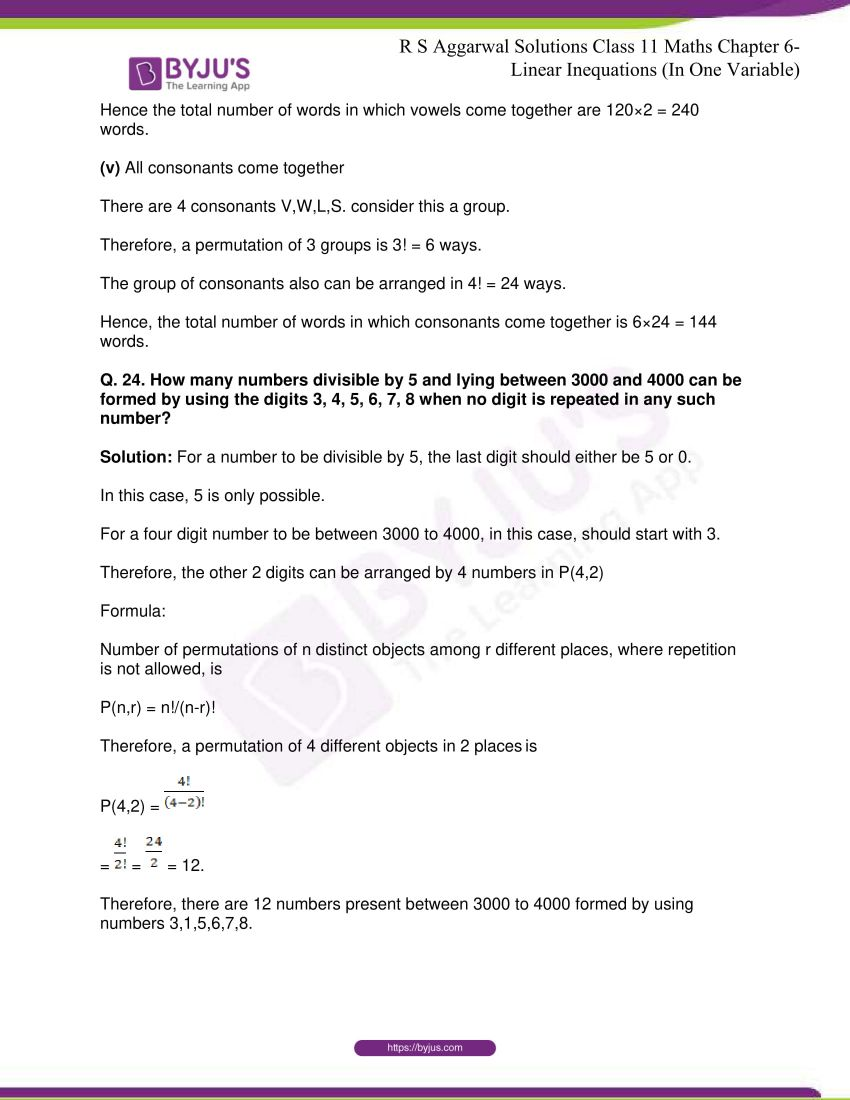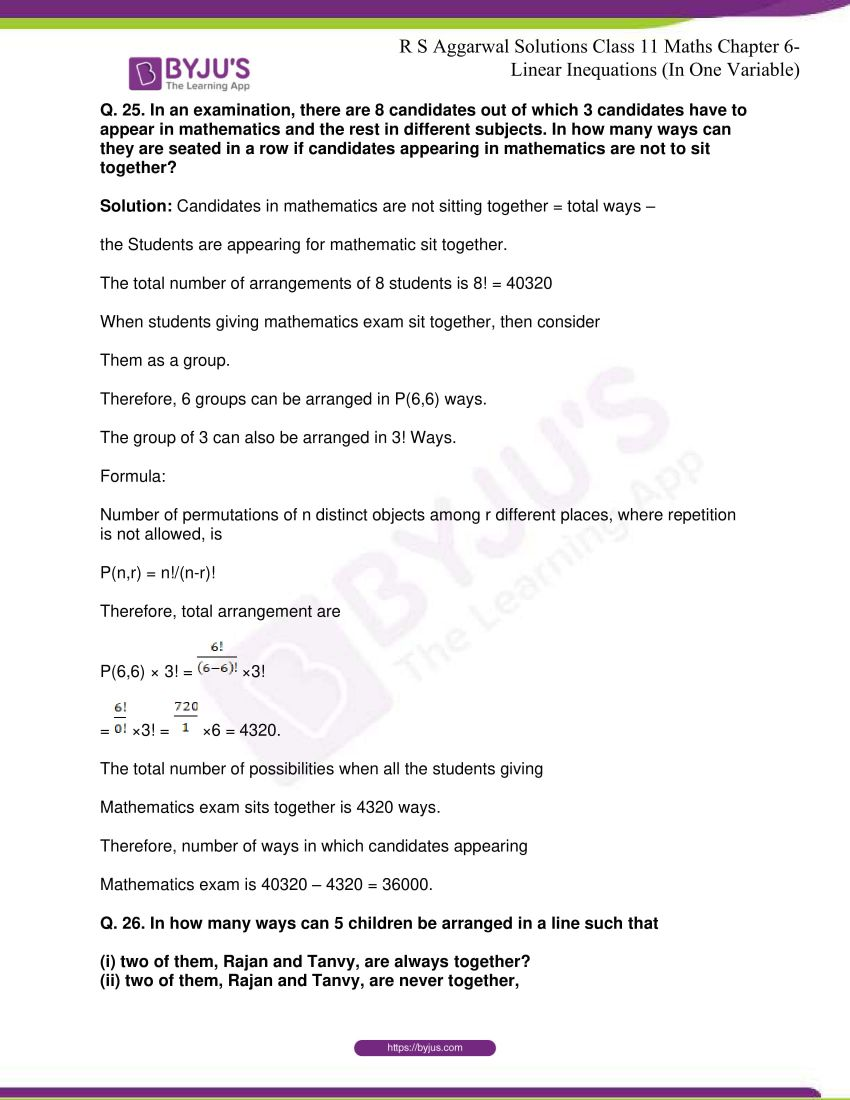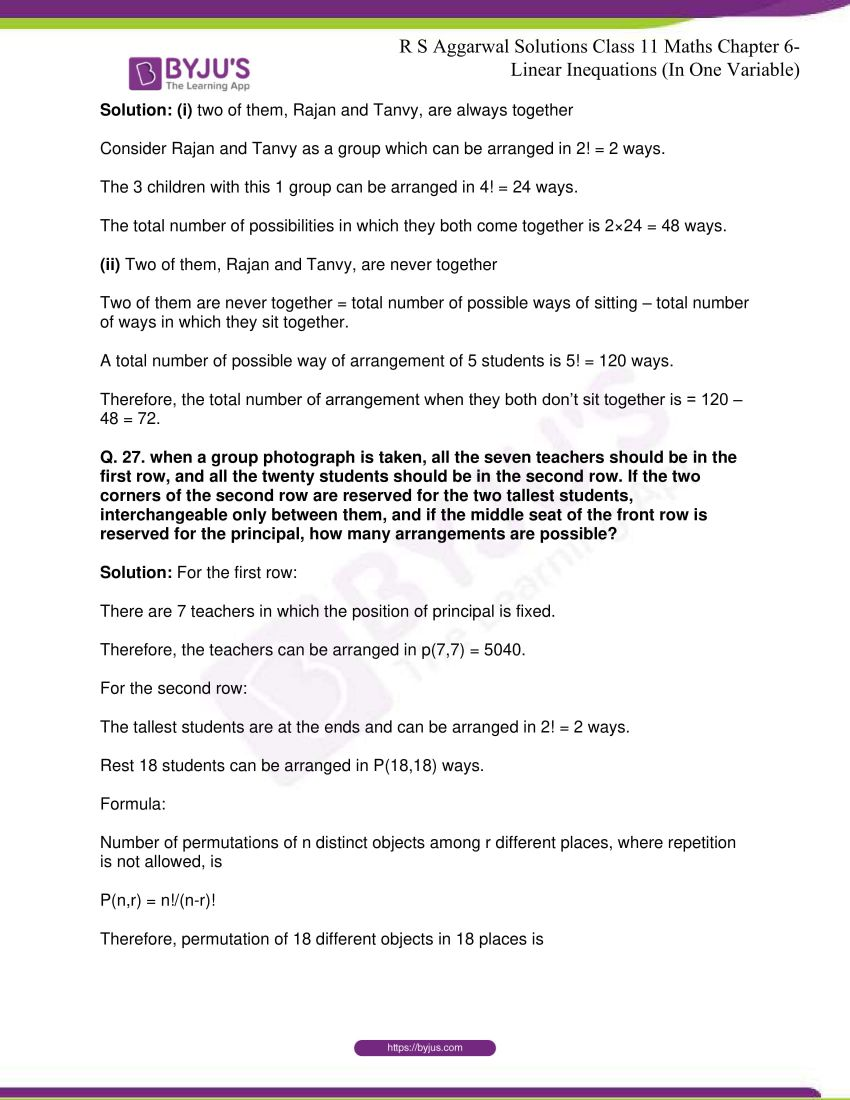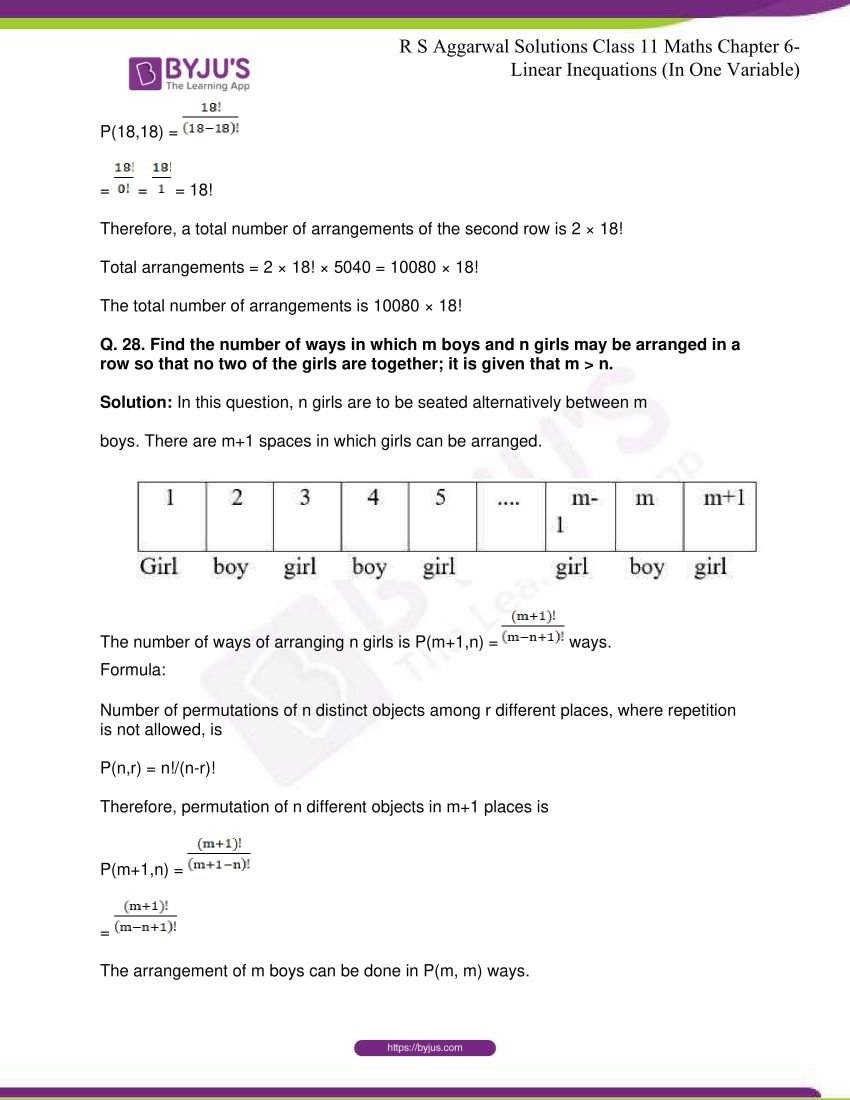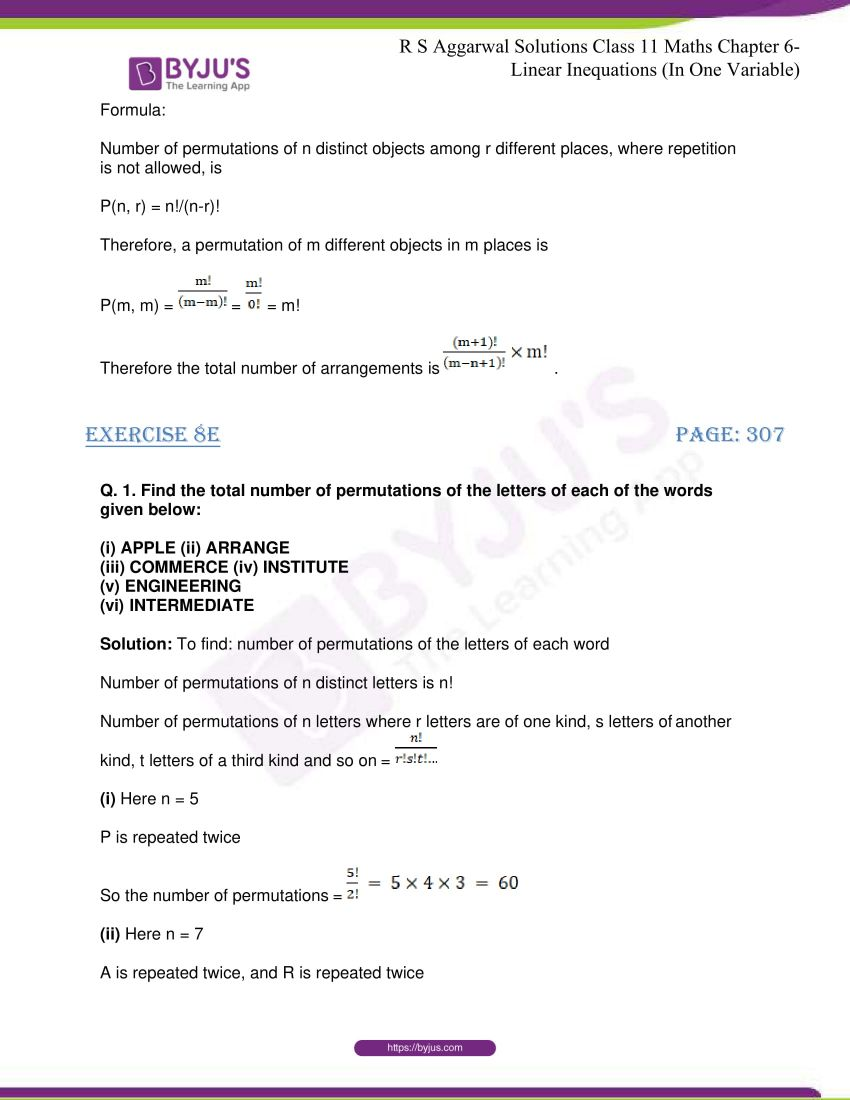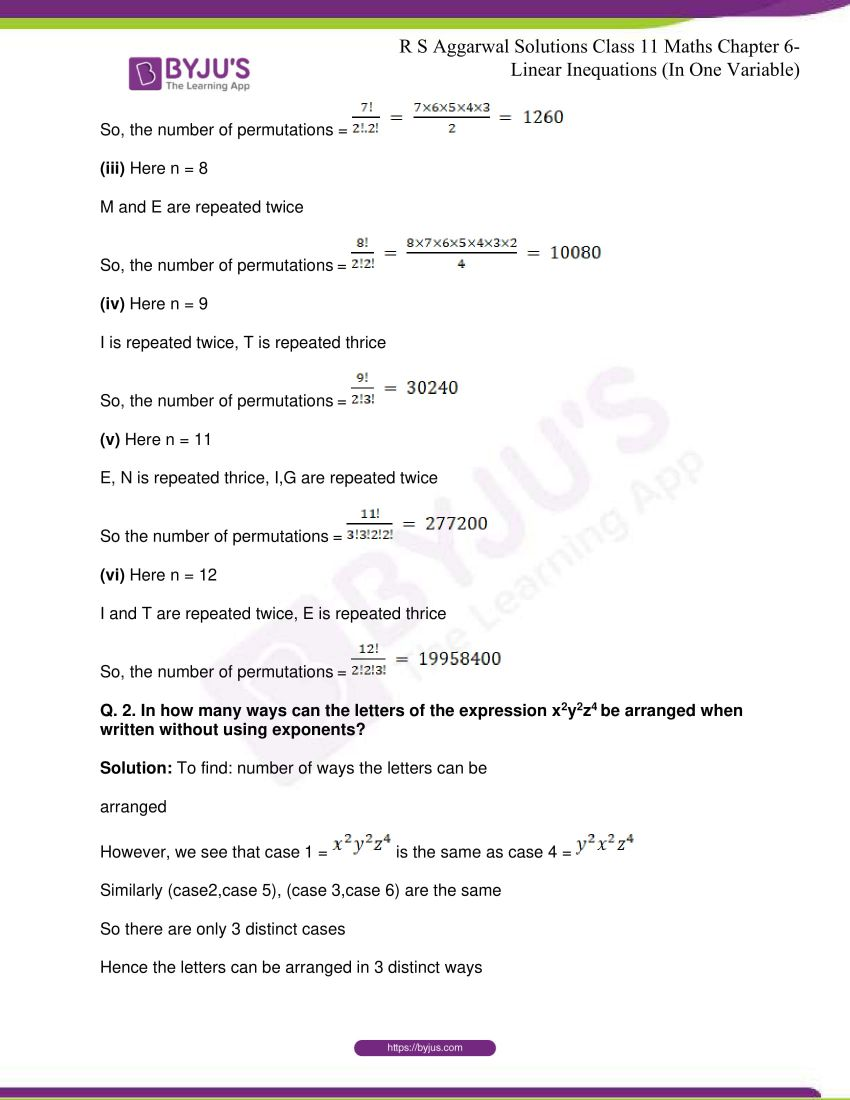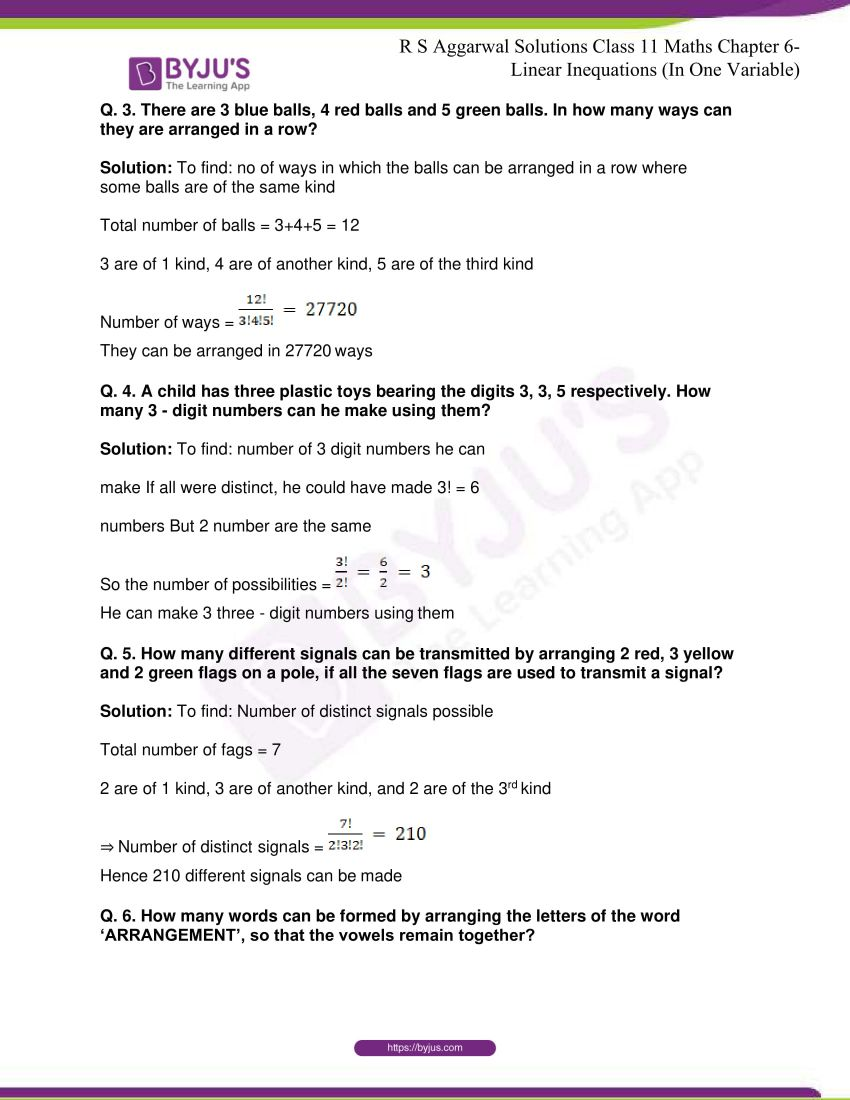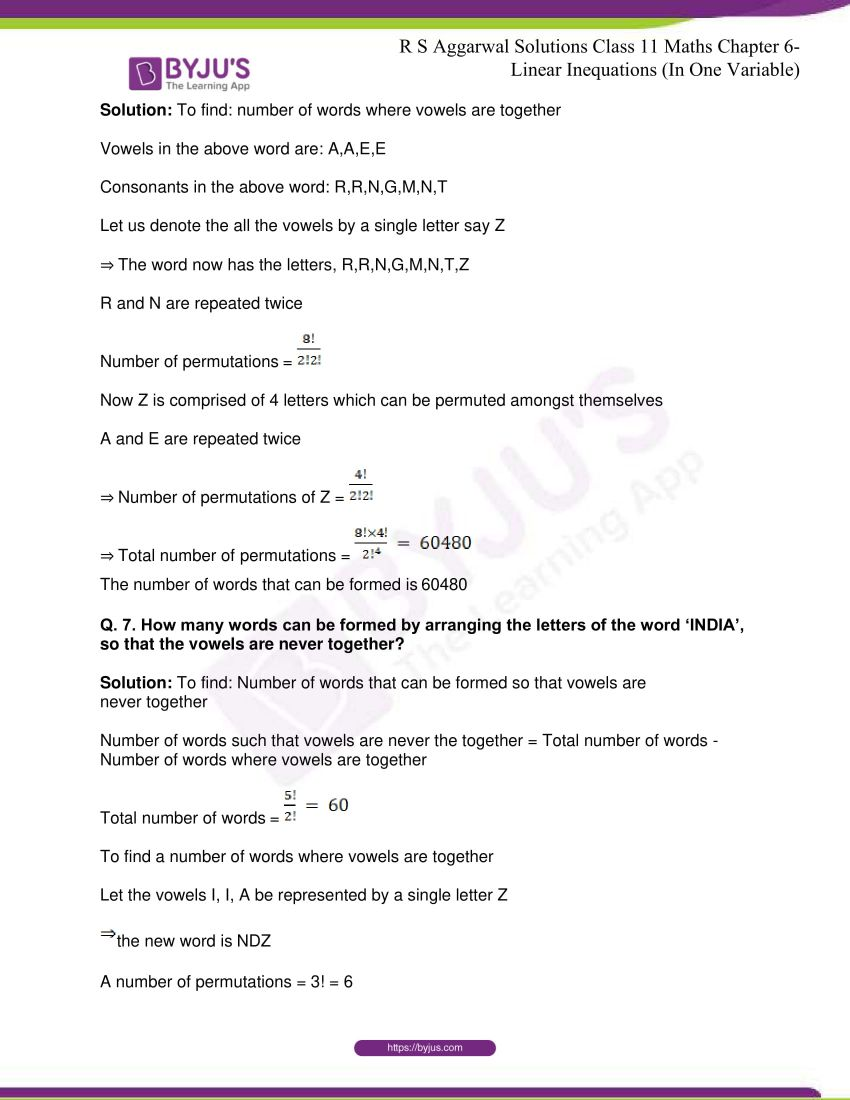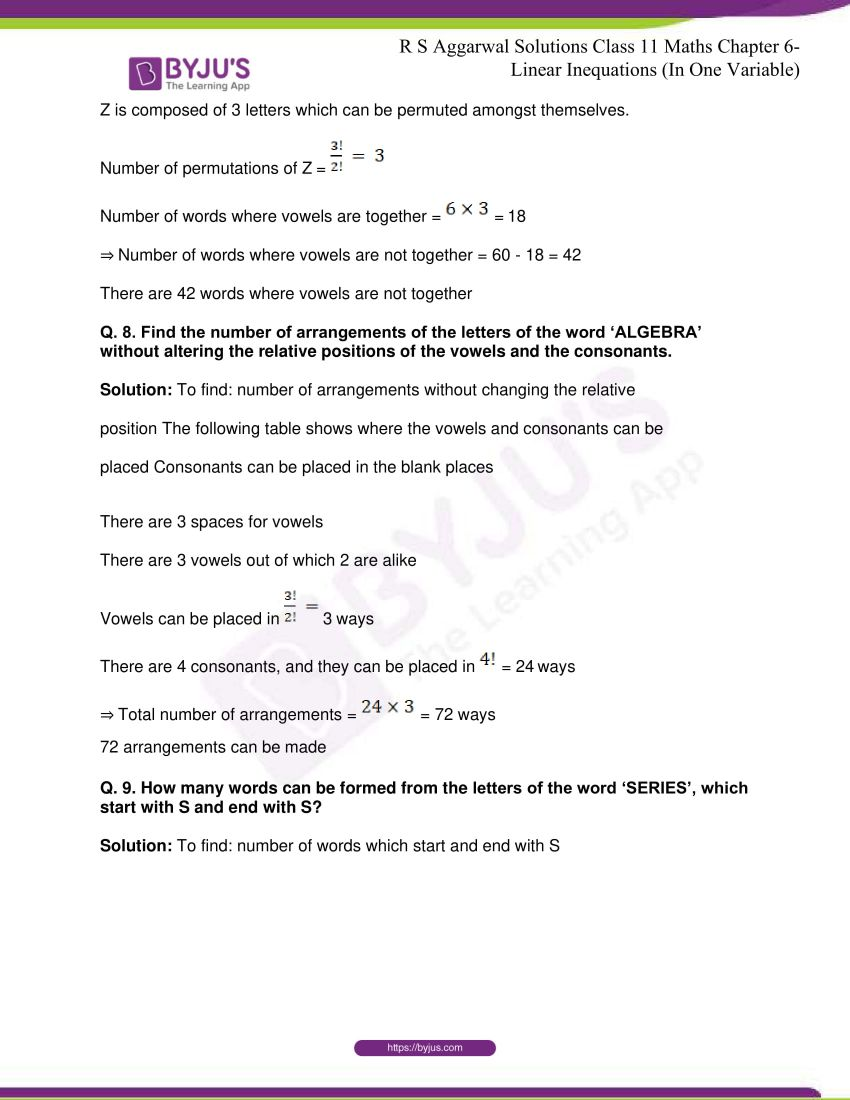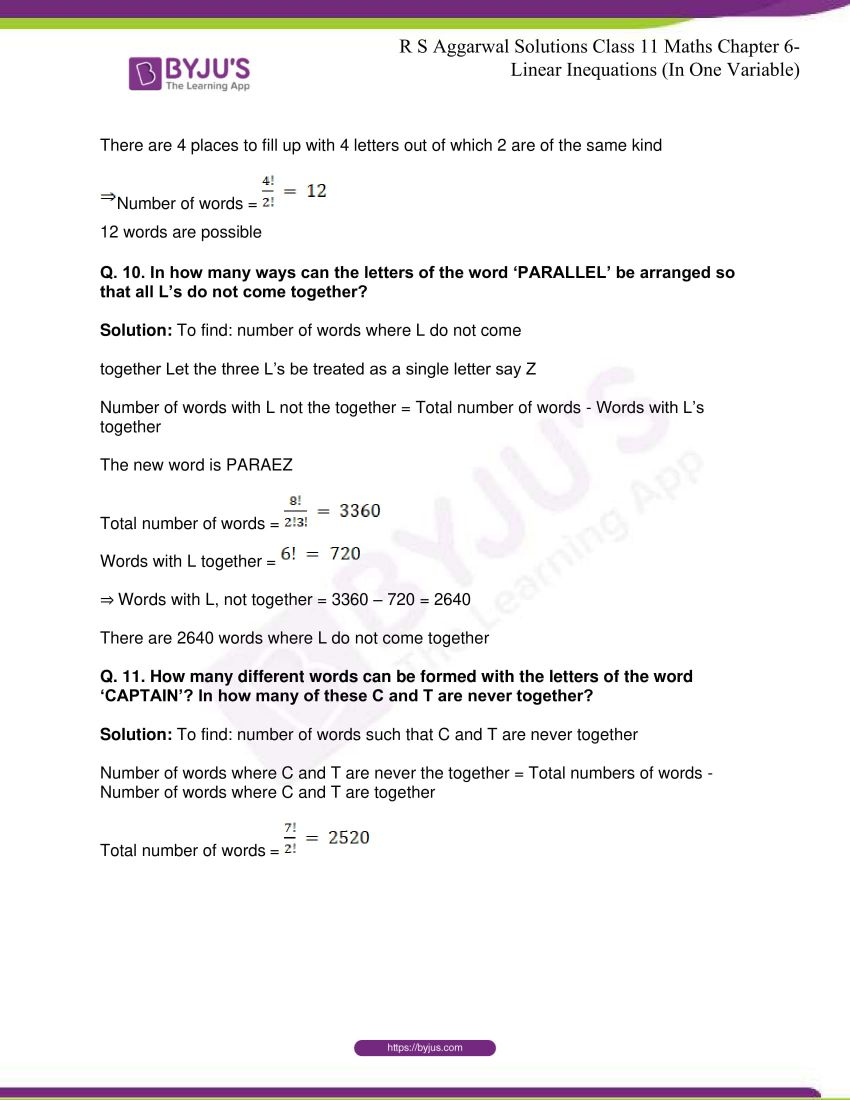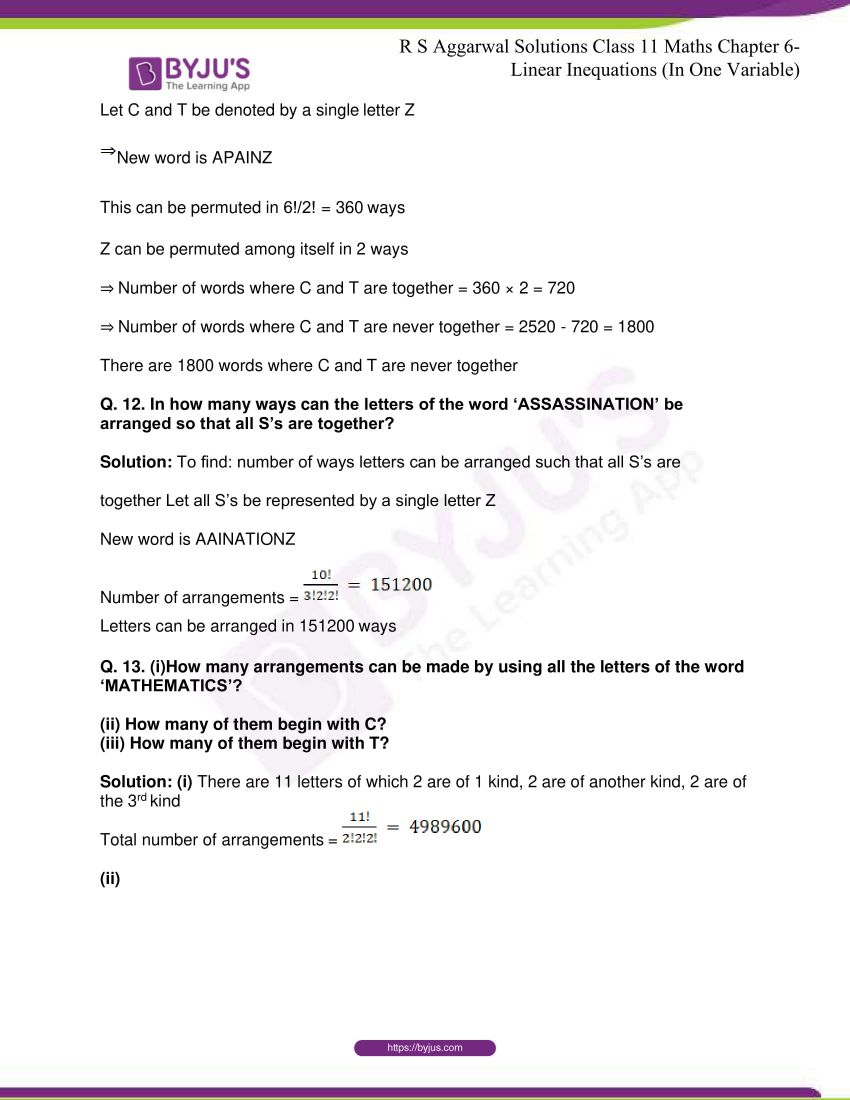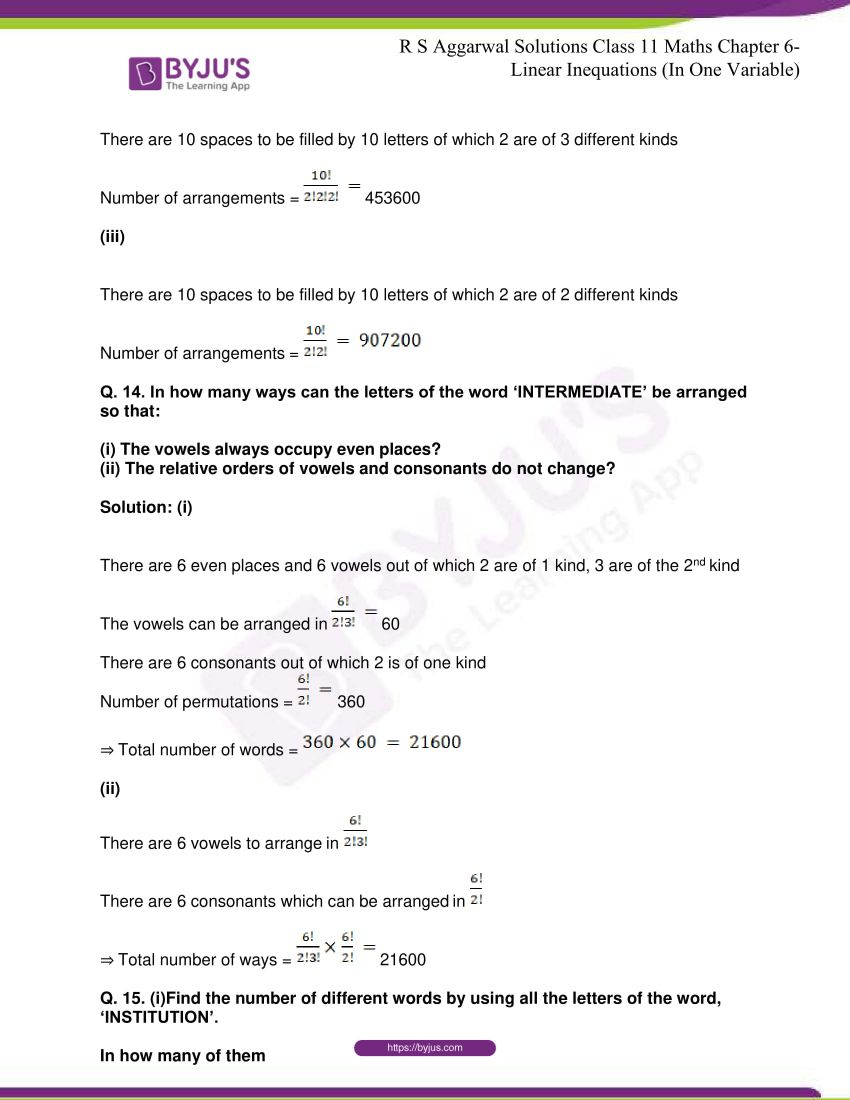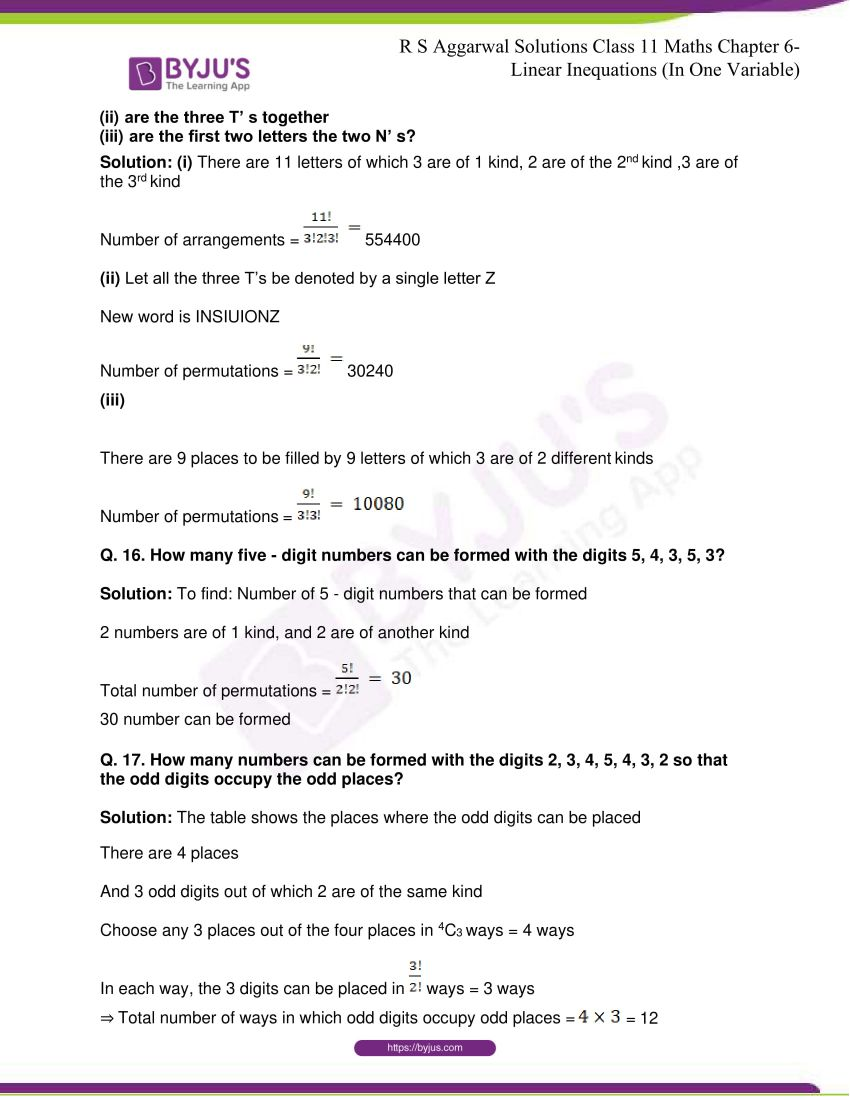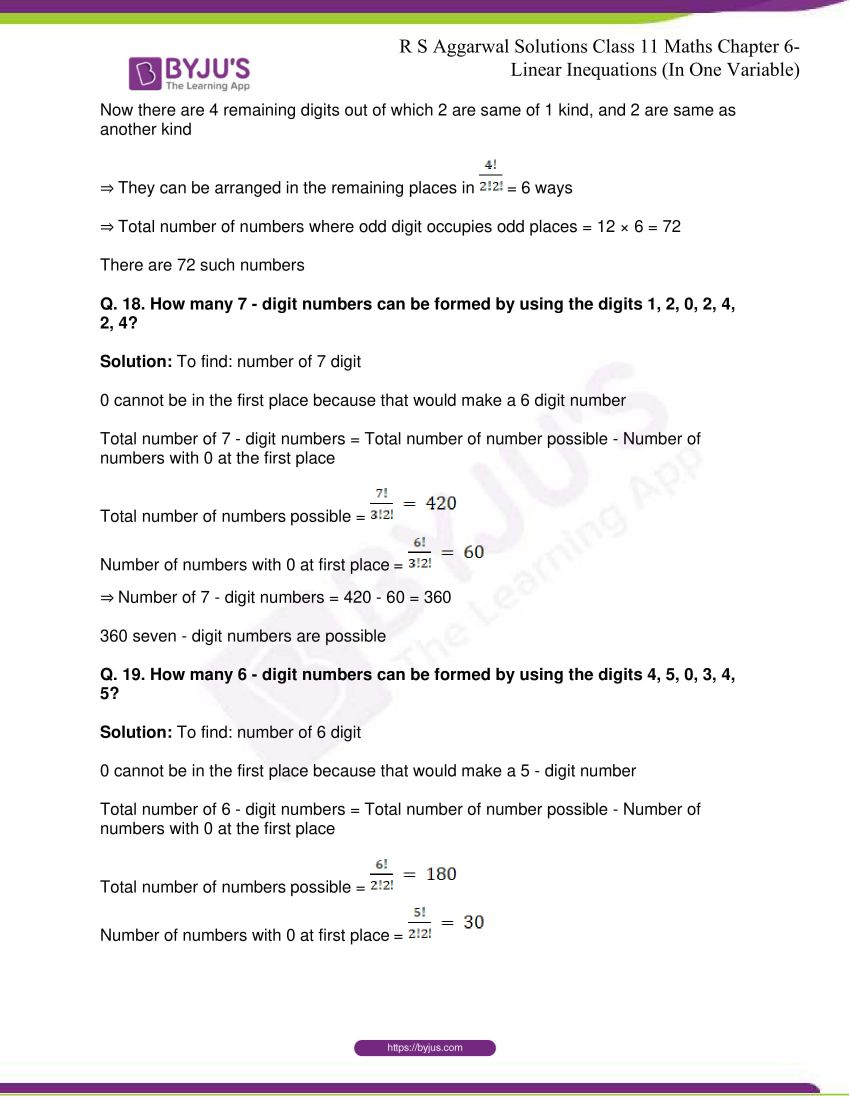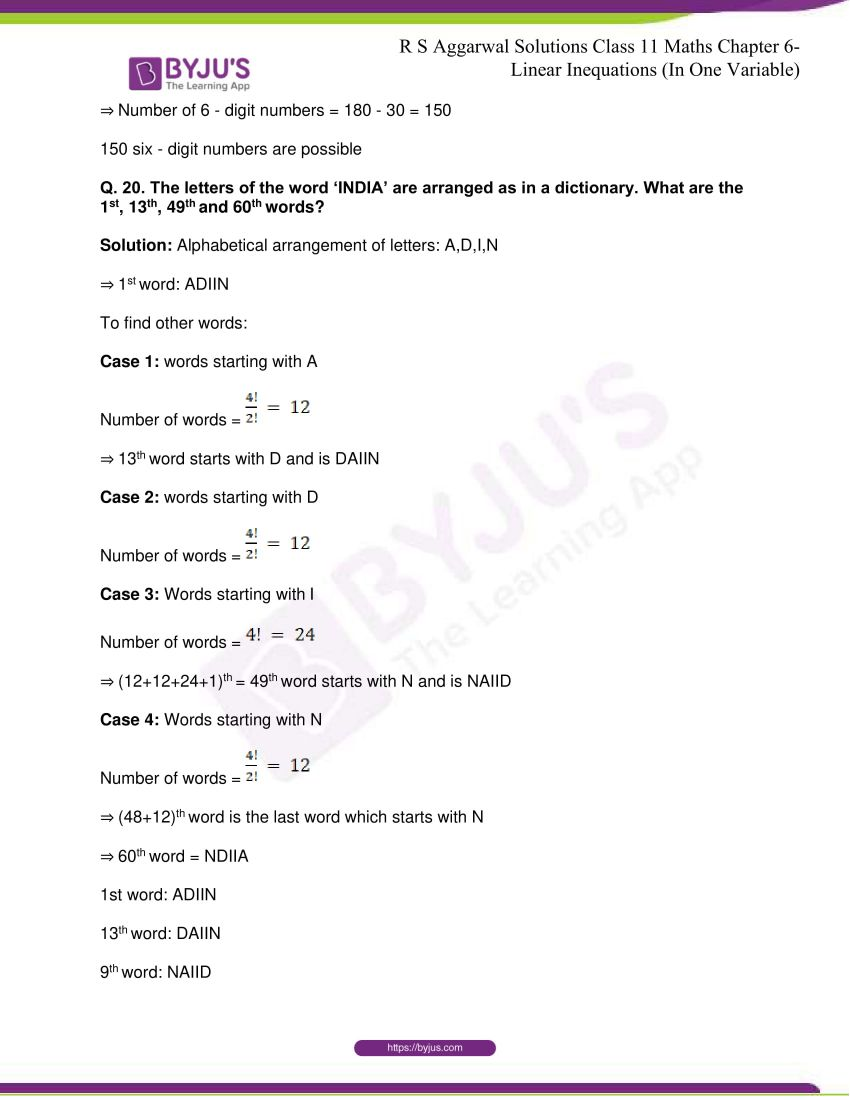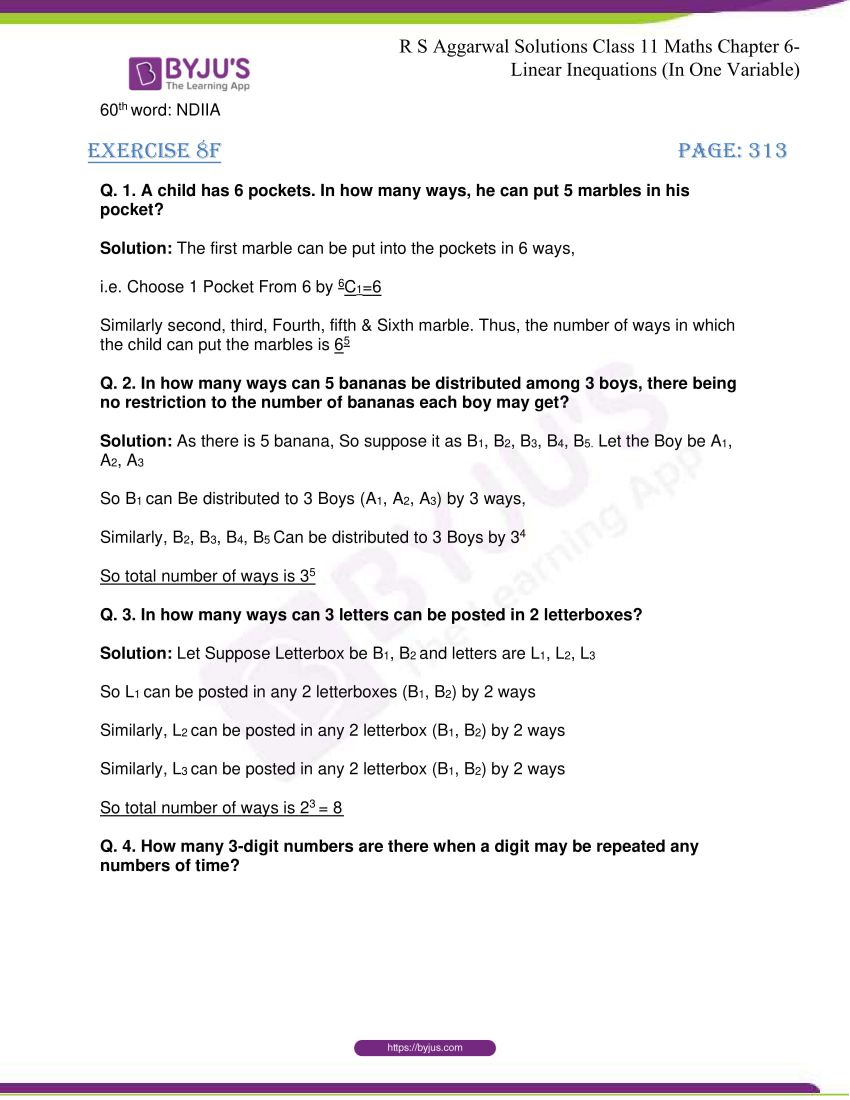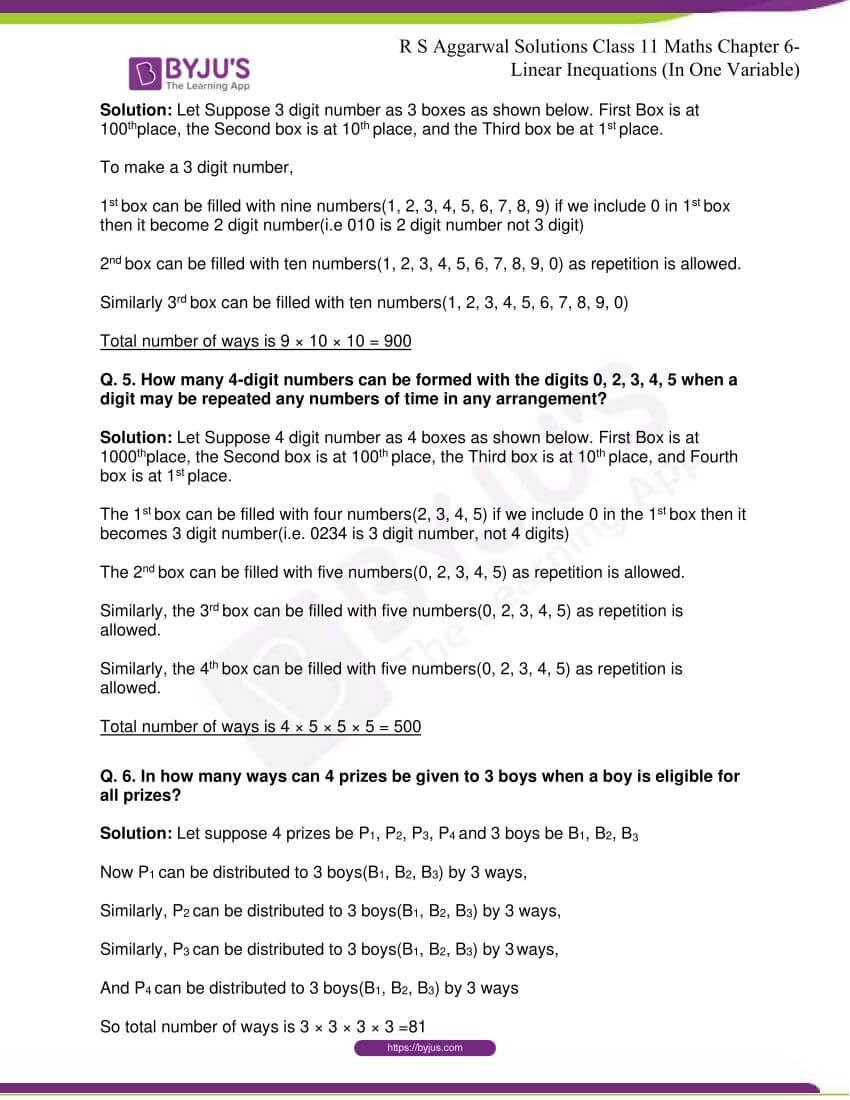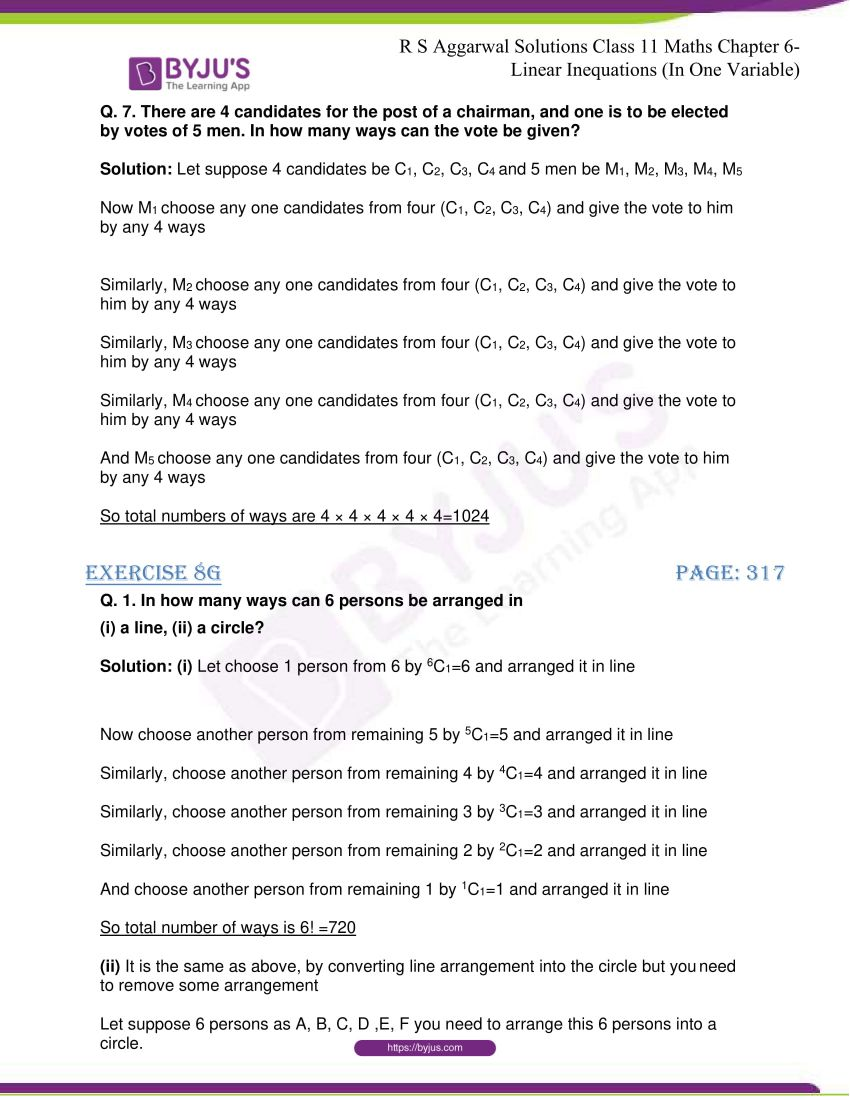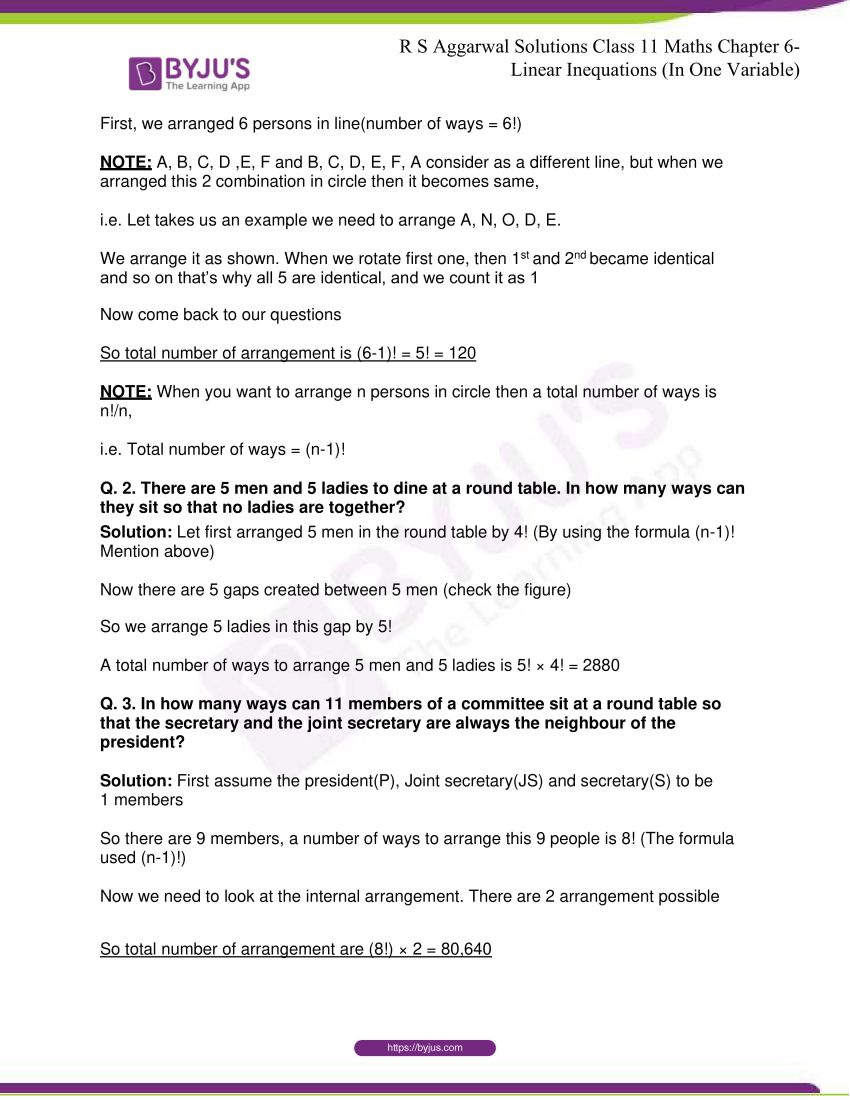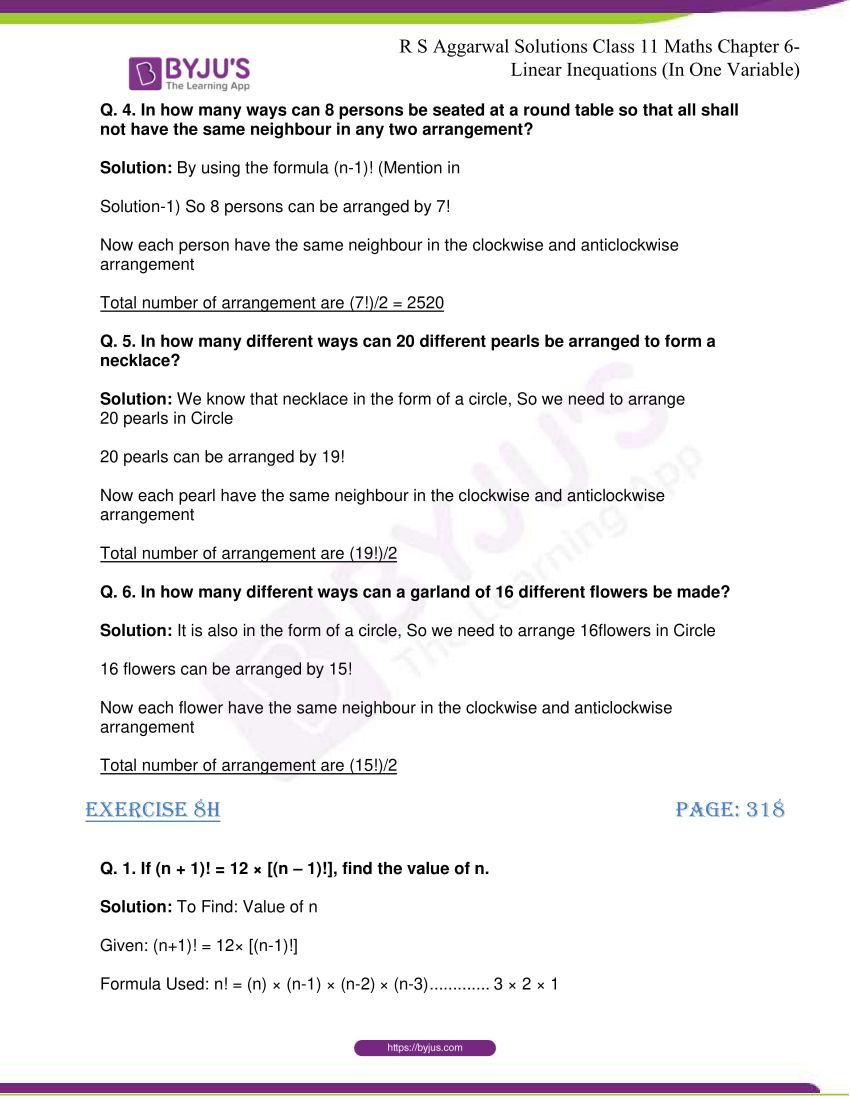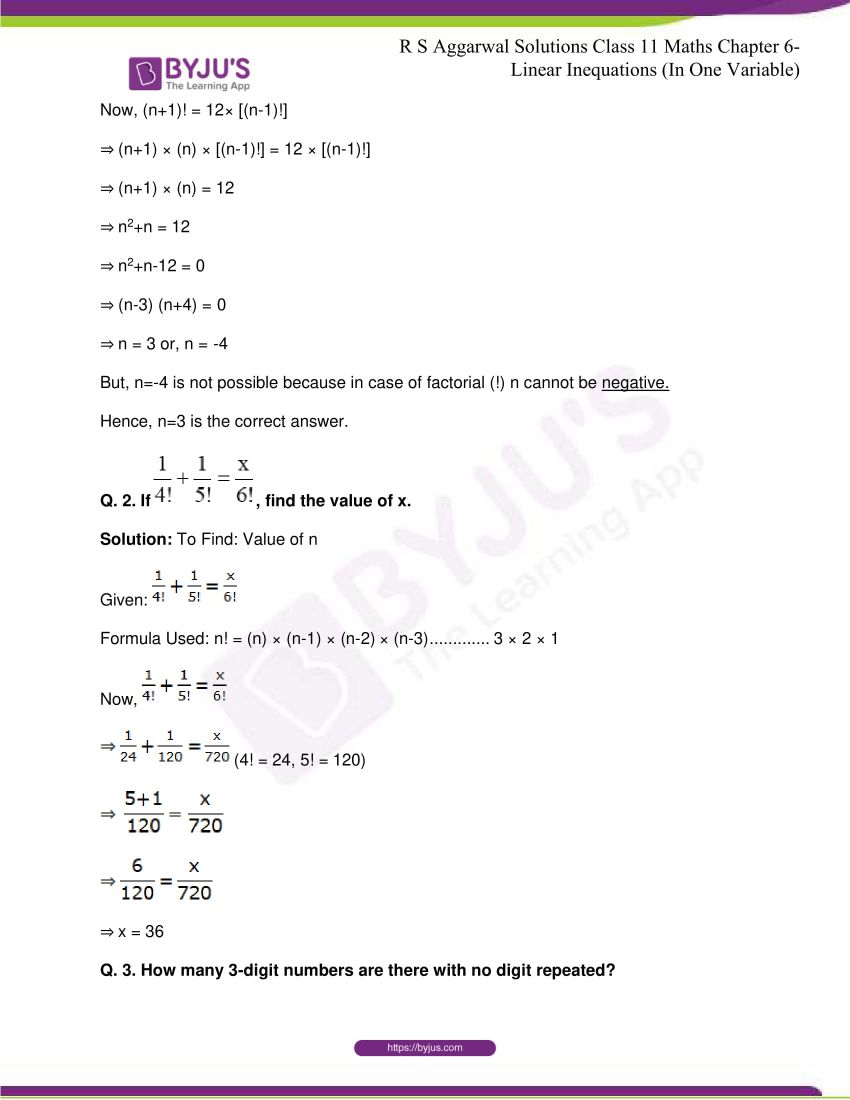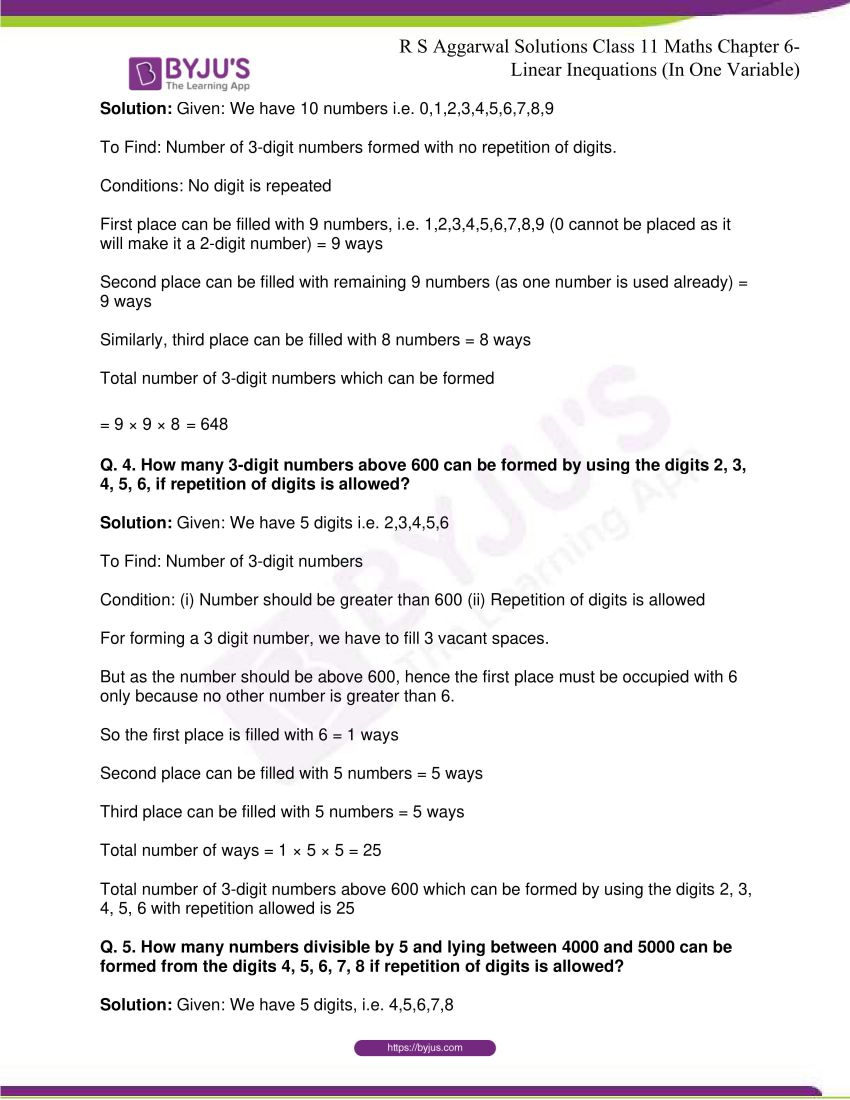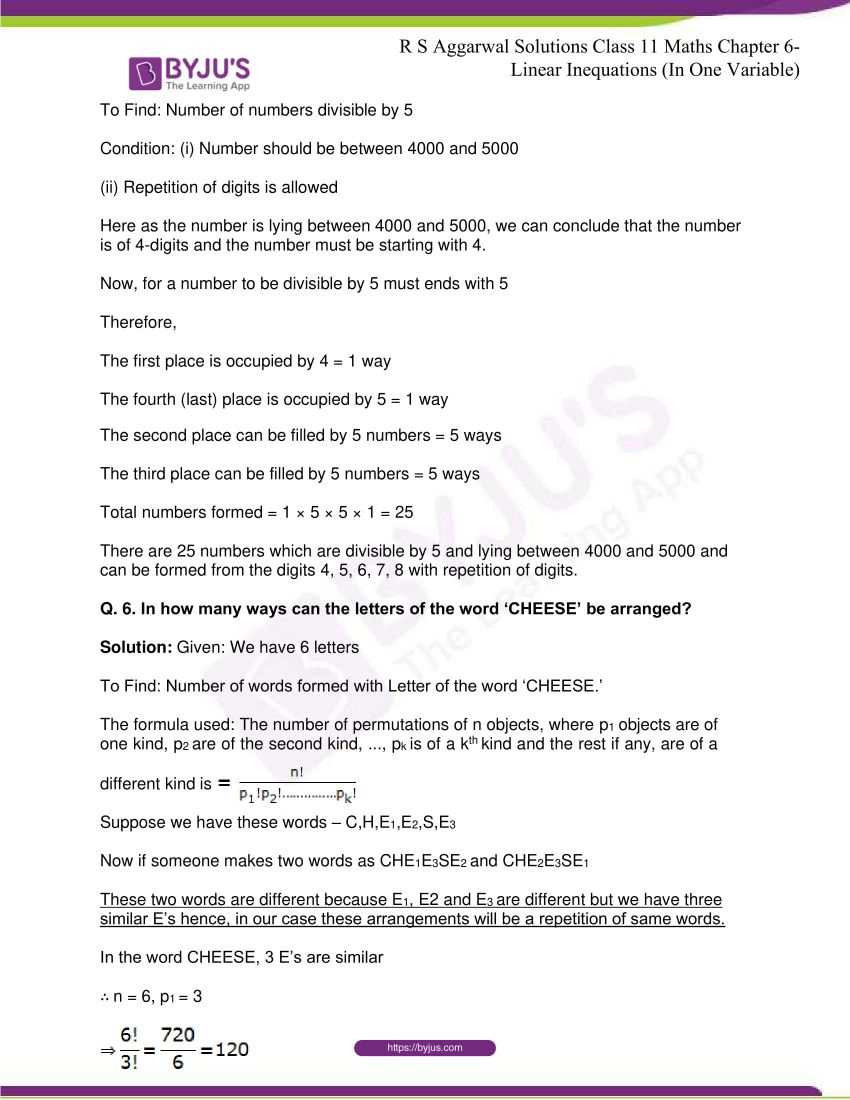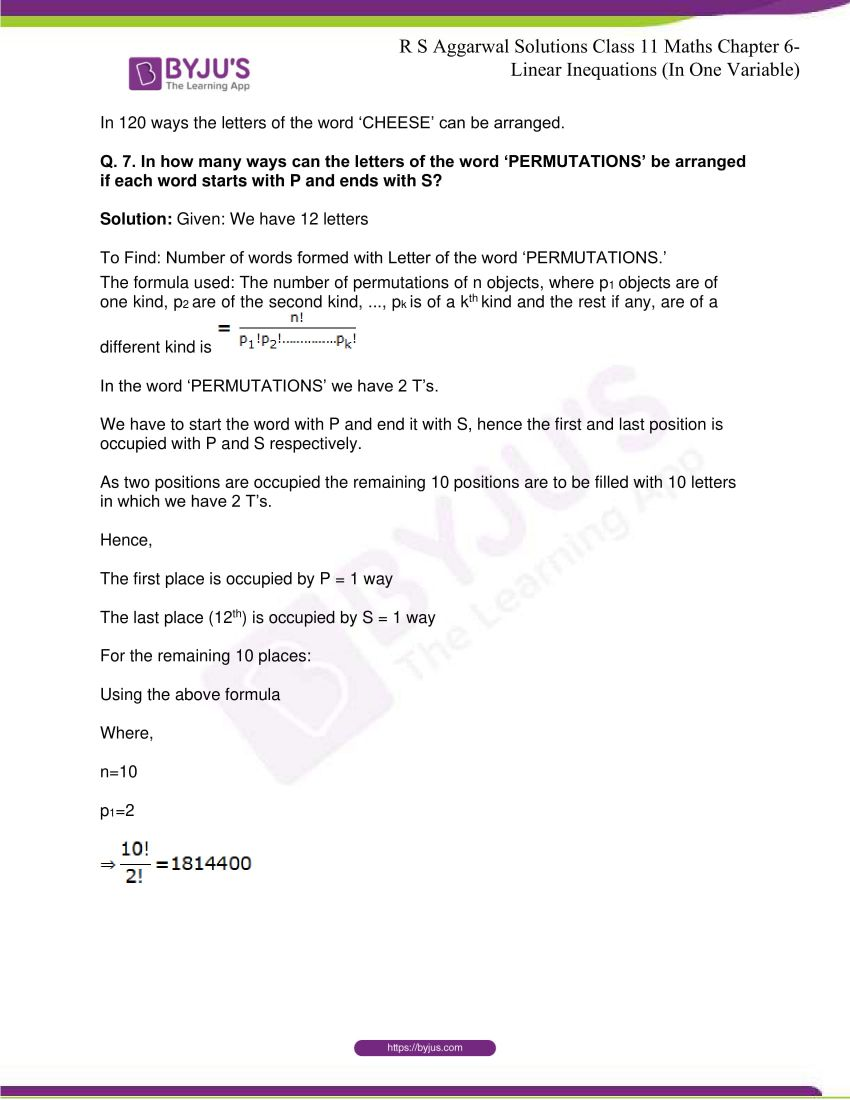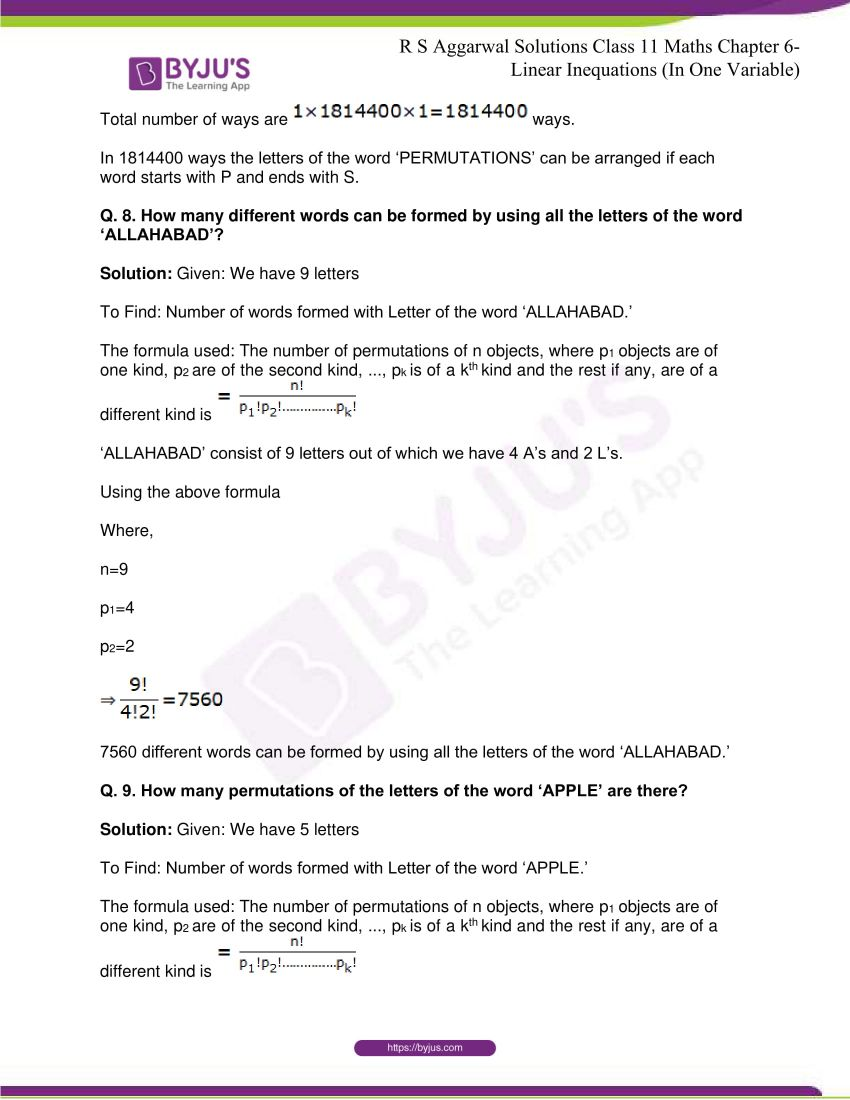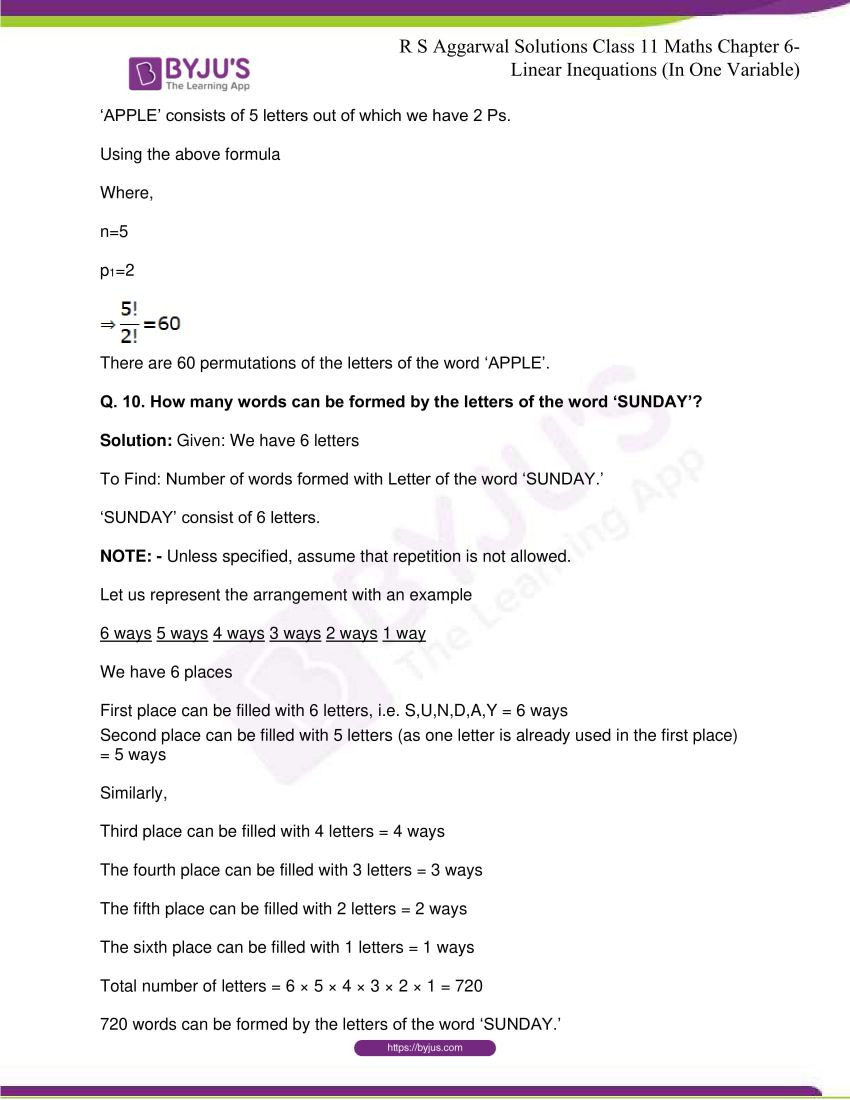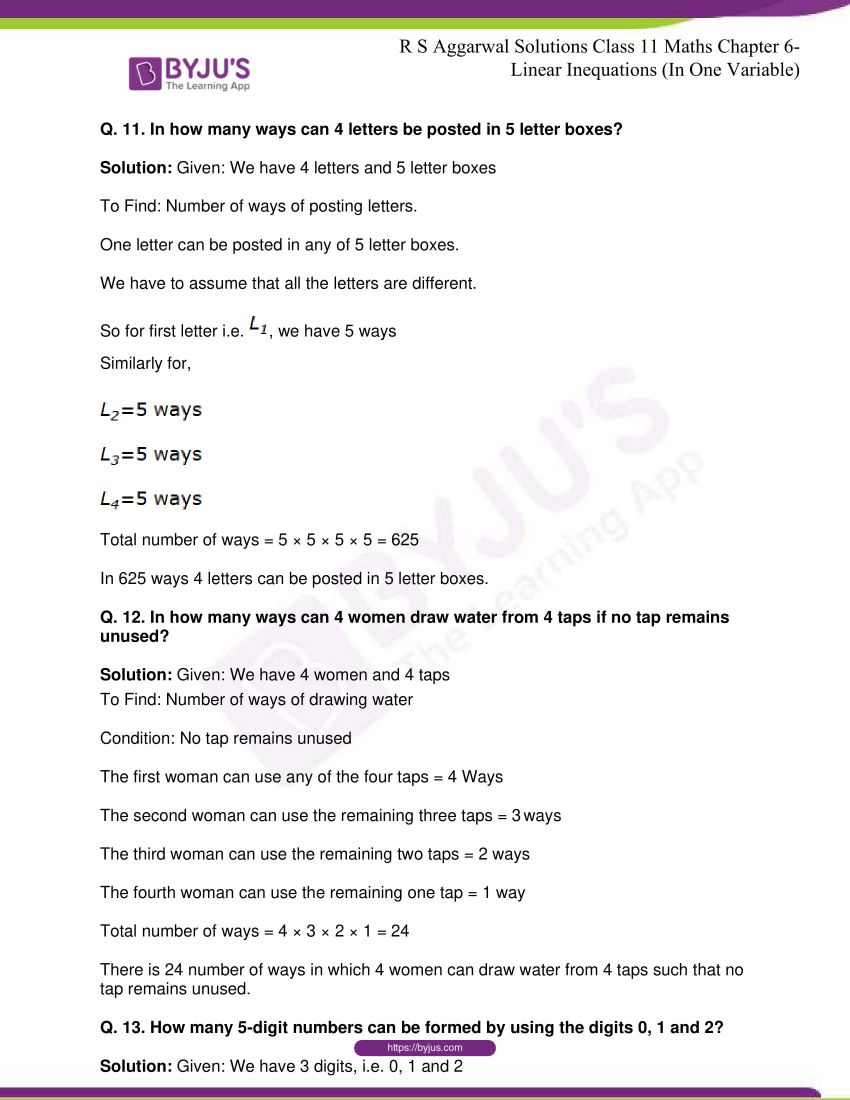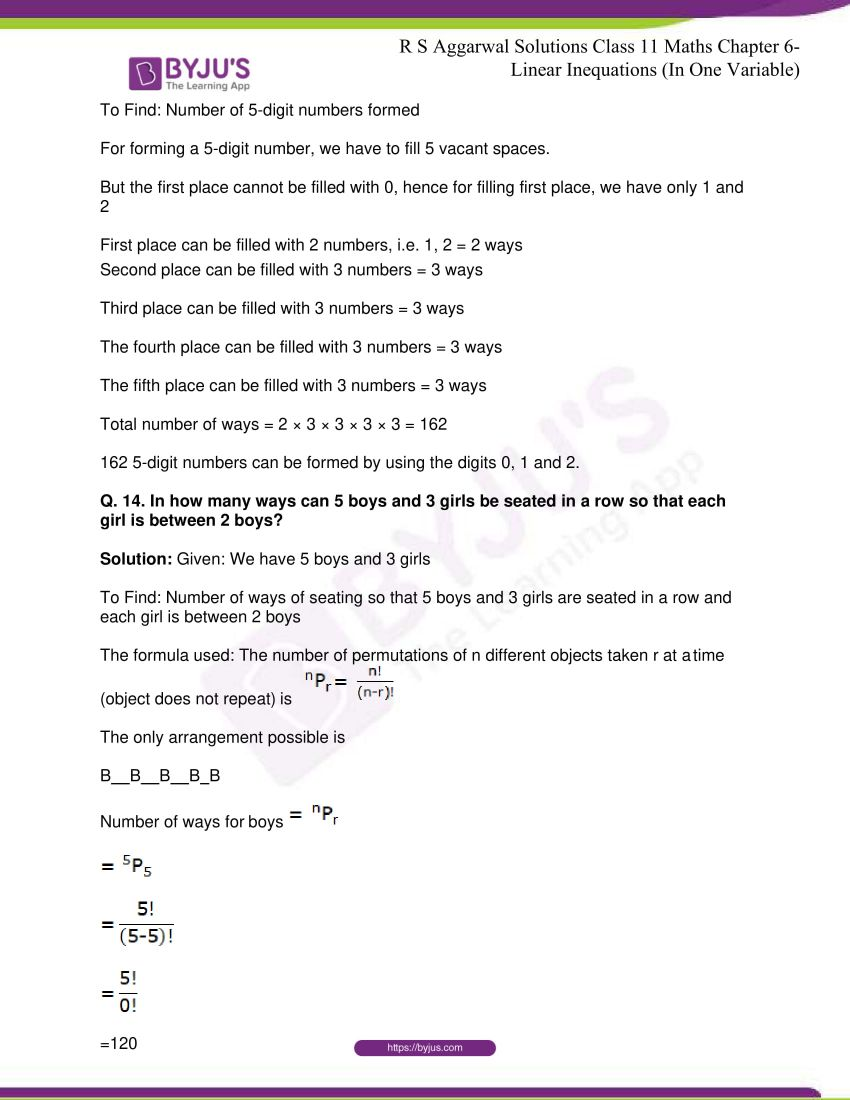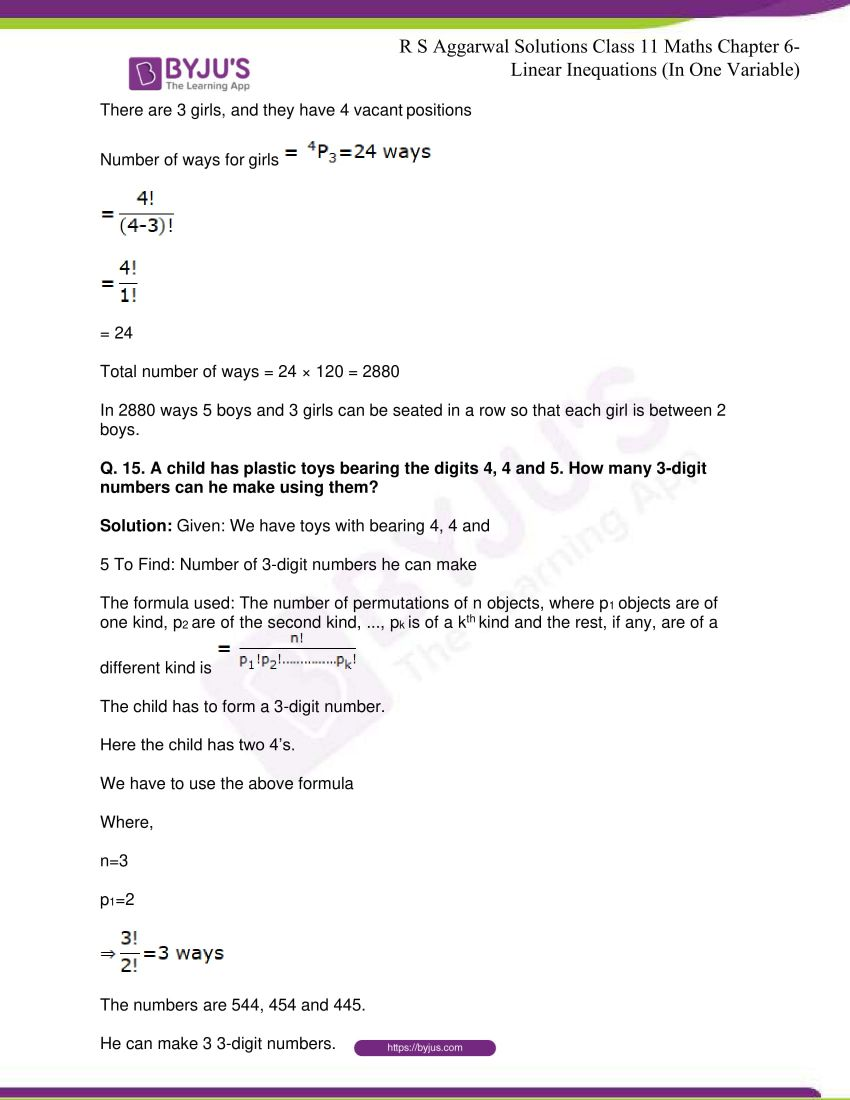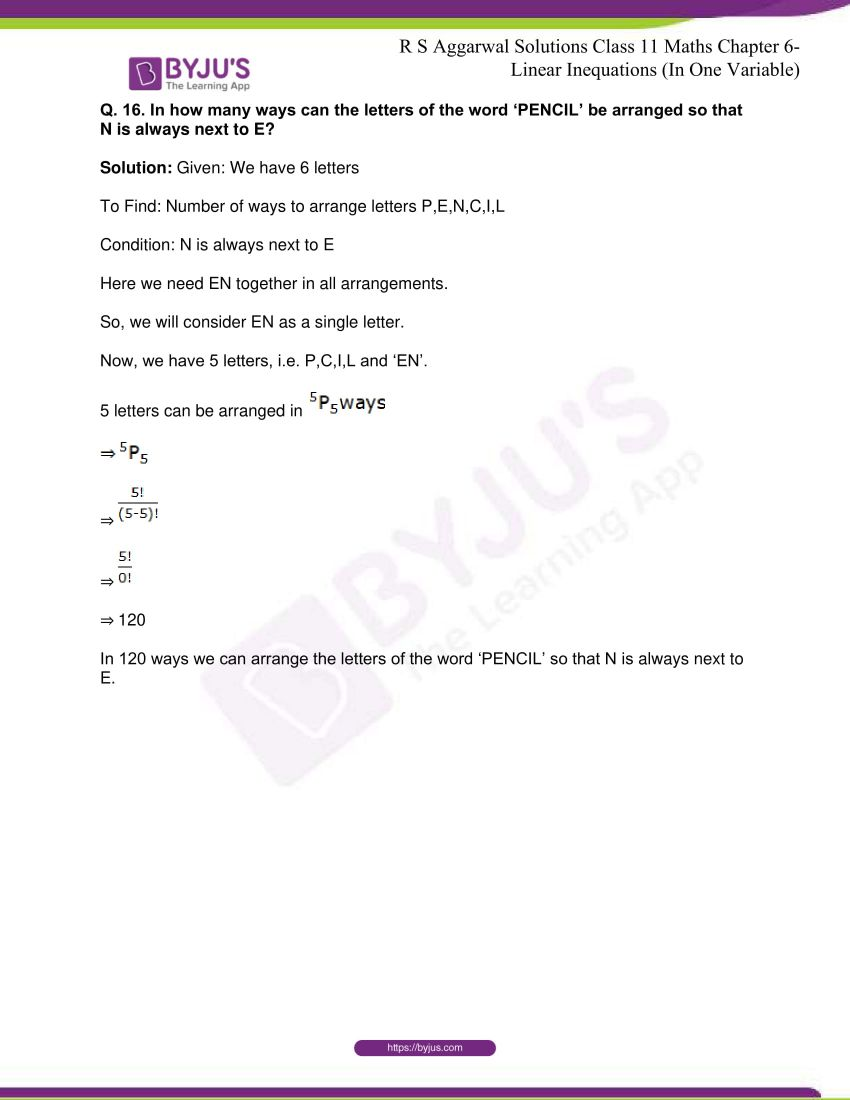## R S Aggarwal Solutions for Class 11 Maths Chapter 8 – Permutations

Chapter 8, Permutations, has 8 exercises and the R S Aggarwal Solutions given here contains the answers to all the questions present in these exercises. Let us have a look at some of the topics that are being discussed in this chapter.

8.1 The Factorial

8.2 Fundamental Principles of Counting

8.3 Permutations

8.4 Word Problems on Permutations

8.5 Permutations of Objects Not All Different

8.6 Permutations with Repetitions

8.7 Circular Permutations

## R S Aggarwal Solutions for Class 11 Maths Chapter 8-Permutations

The different arrangements which can be made out of a given number of things by taking some or all at a time, are called permutation. Read and learn Chapter 8 of R S Aggarwal textbook to learn more about Permutations, along with the concepts covered in it. Solve the R S Aggarwal Solutions for Class 11 effectively to score high in the examination.